# Concise Selina Solutions for Class 9 Maths Chapter 10- Isosceles Triangle

Selina Solutions for Class 9 Maths Chapter 10 Isosceles Triangle are provided here. Class 9 is an important phase in a student’s life. It is crucial to understand the concepts taught in Class 9 as these concepts are continued in Class 10. To score good marks in Class 9 Mathematics examination, it is advised to solve questions provided in each exercise across all the chapters in the book by Selina publication. This Selina solutions for Class 9 Maths helps students in understanding all the concepts in a better way. Download pdf of Class 9 Maths Chapter 10 Selina Solutions from the given link.

### Download PDF of Selina Solutions for Class 9 Maths Chapter 10:-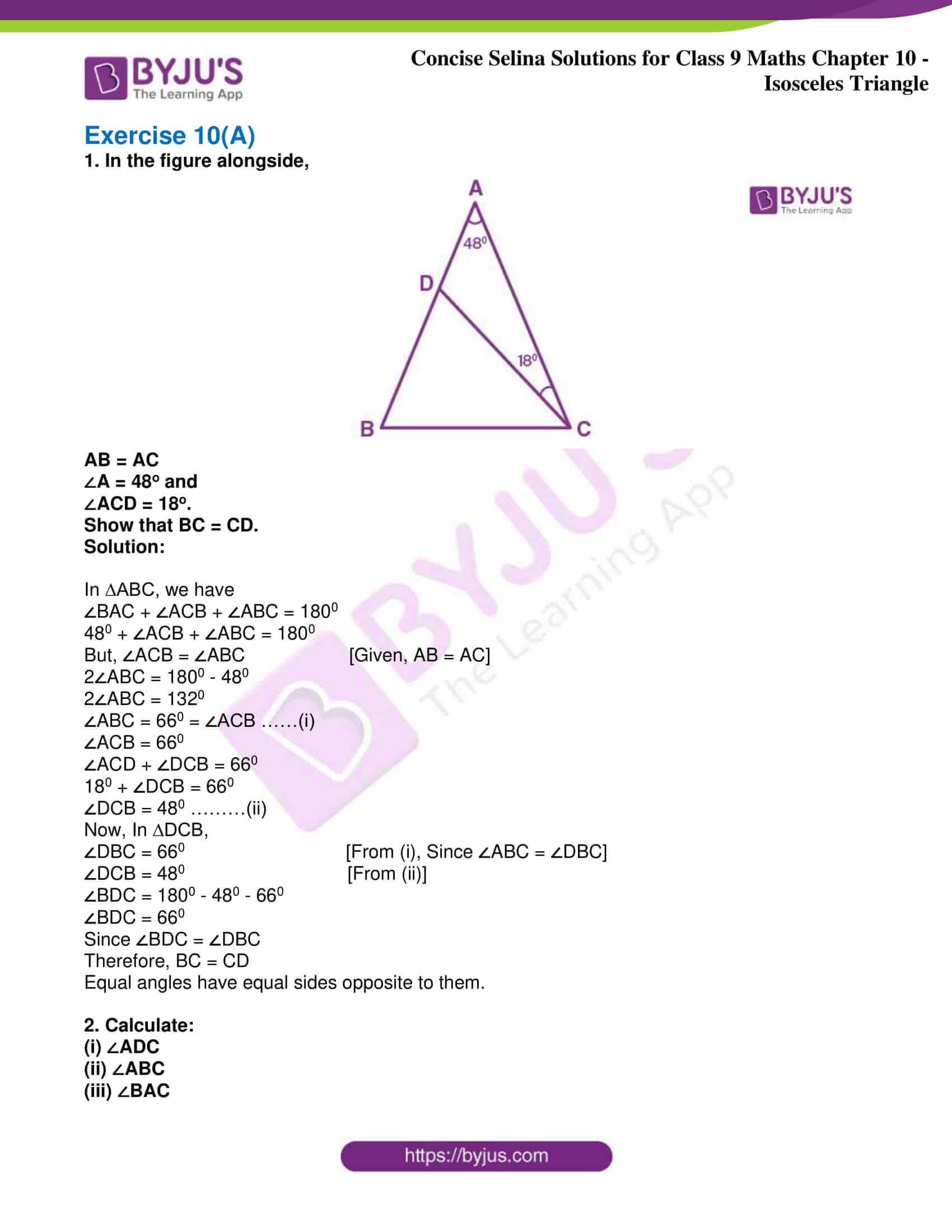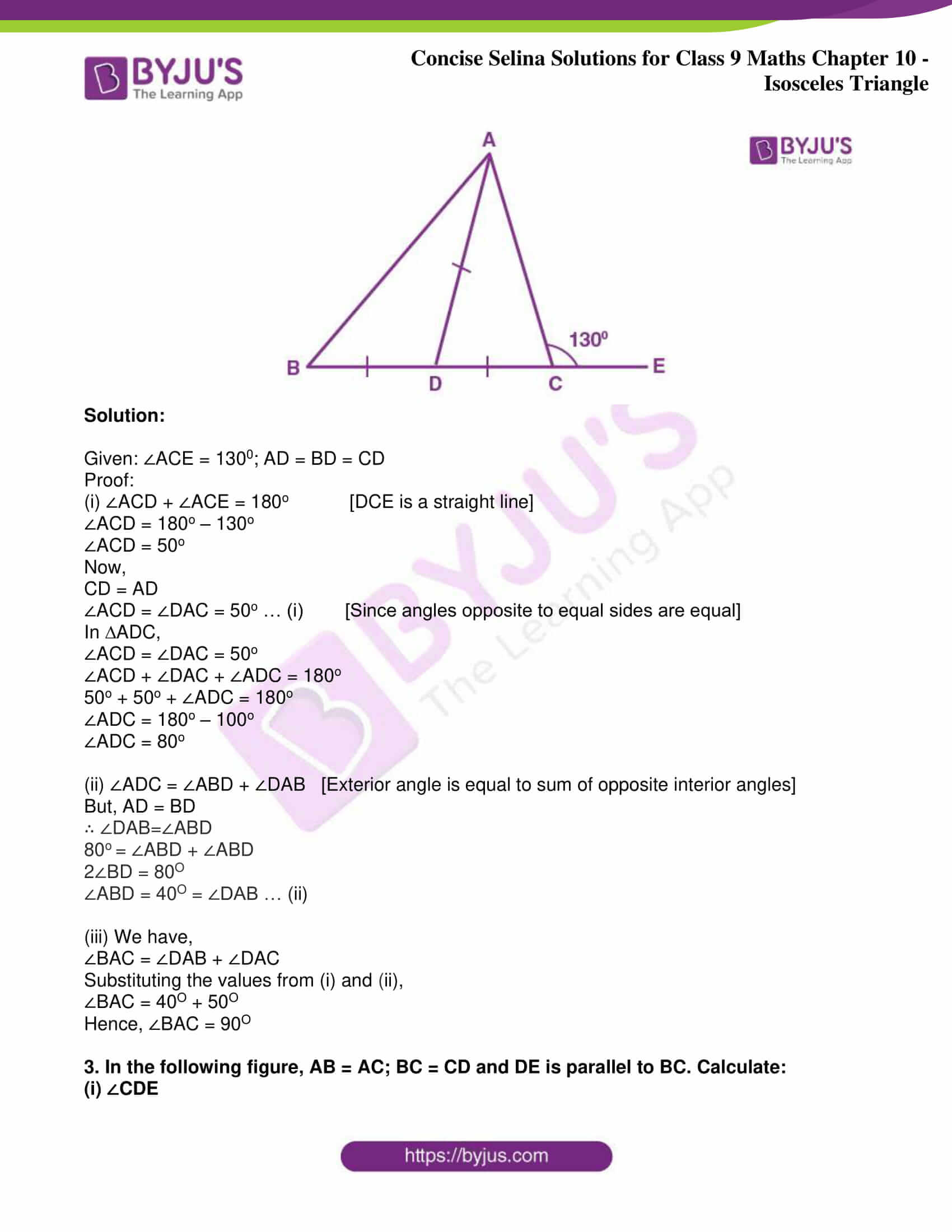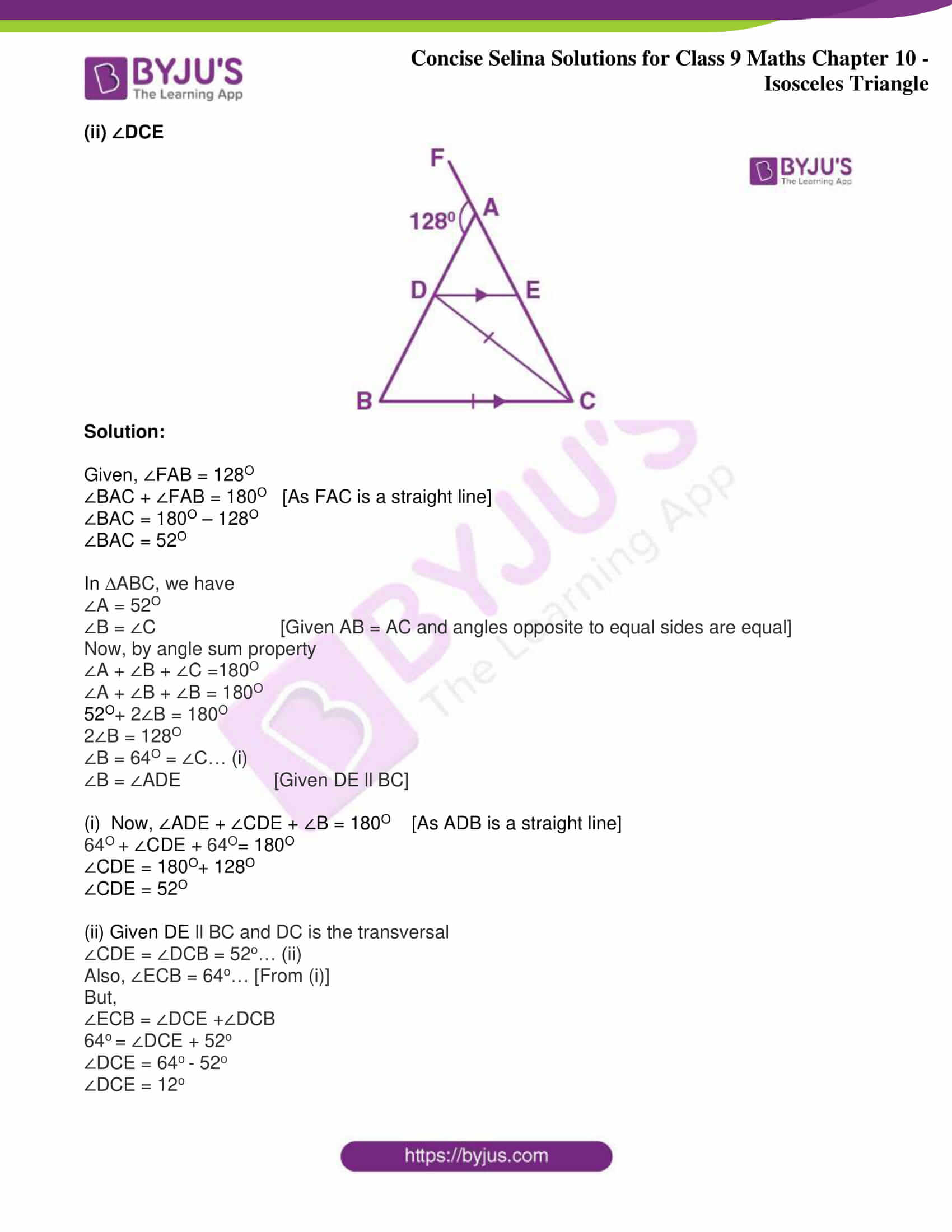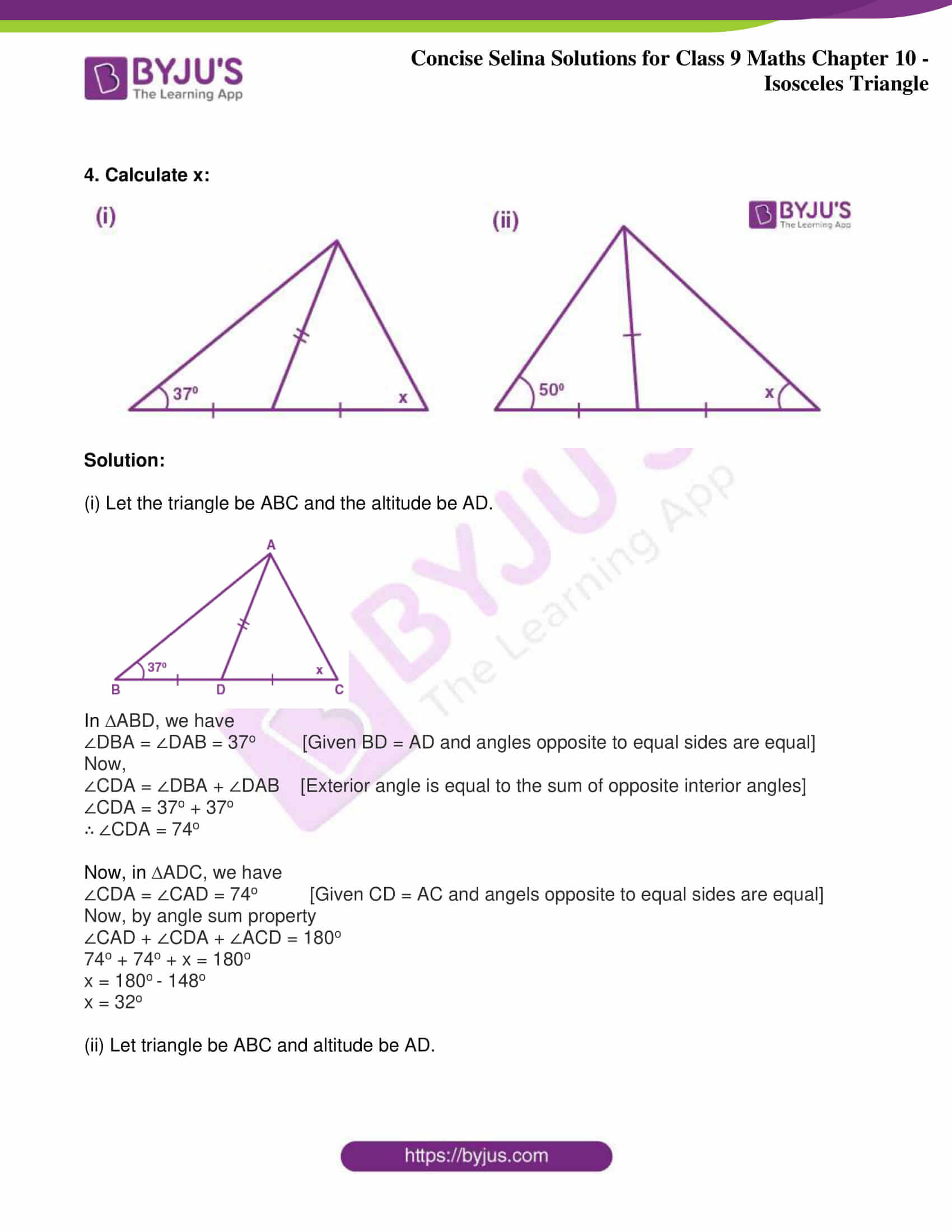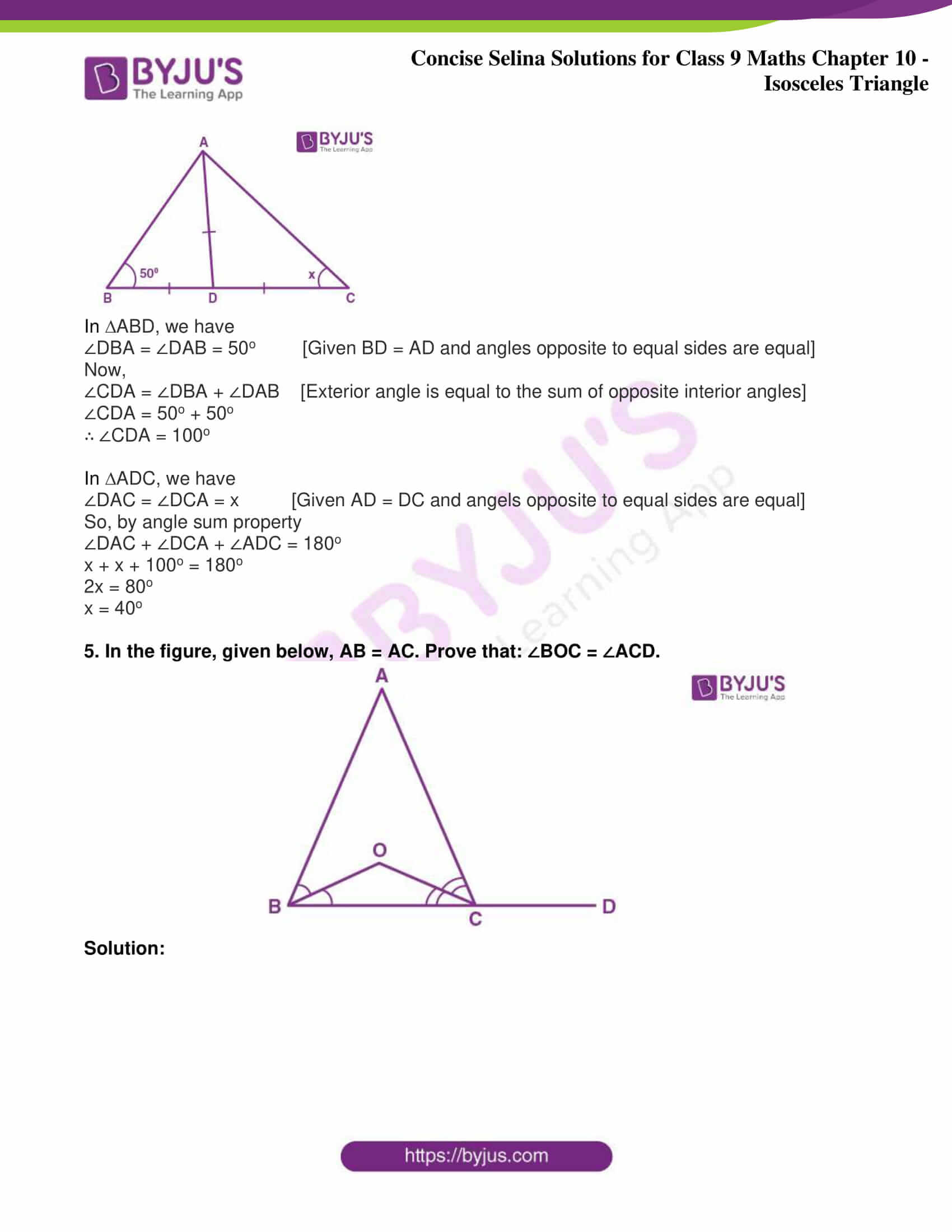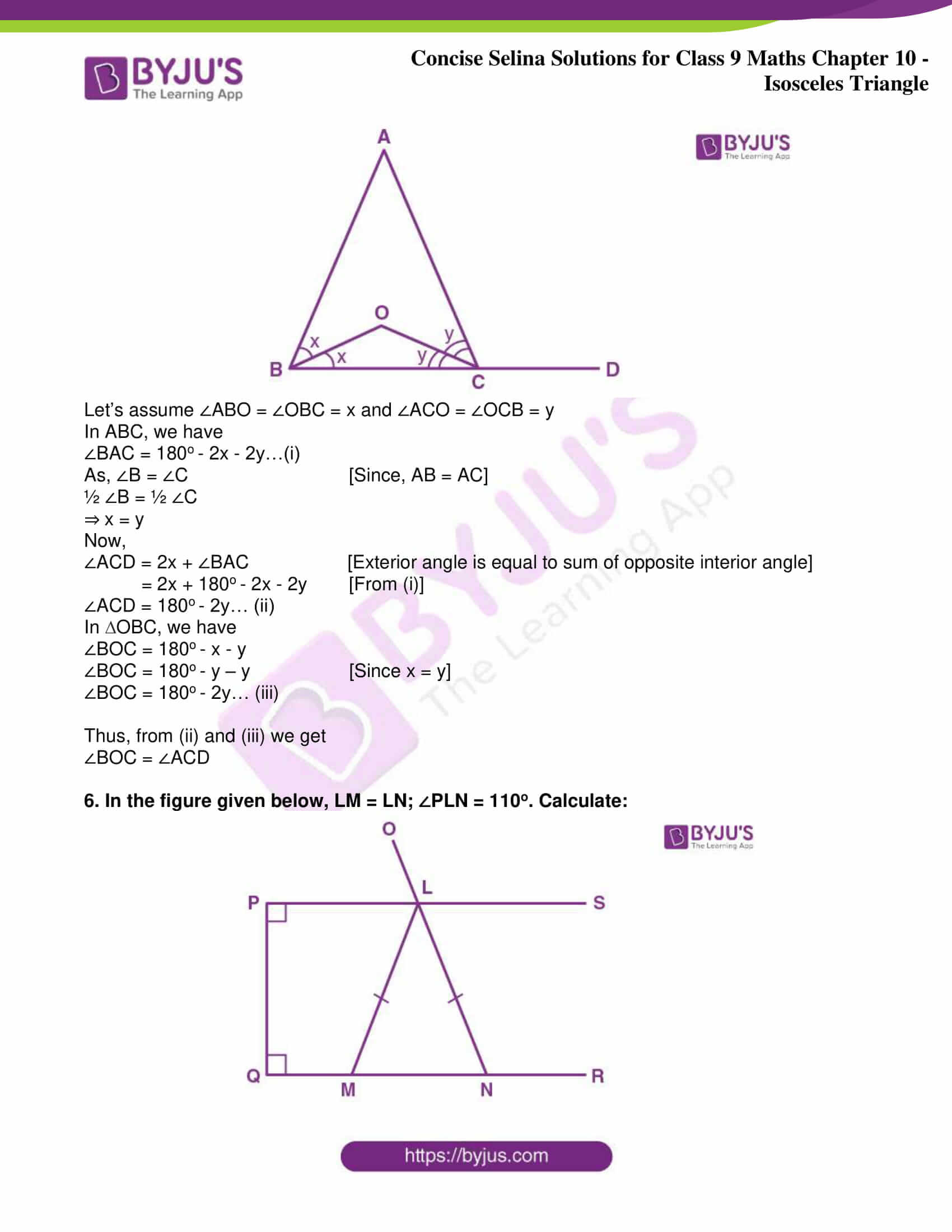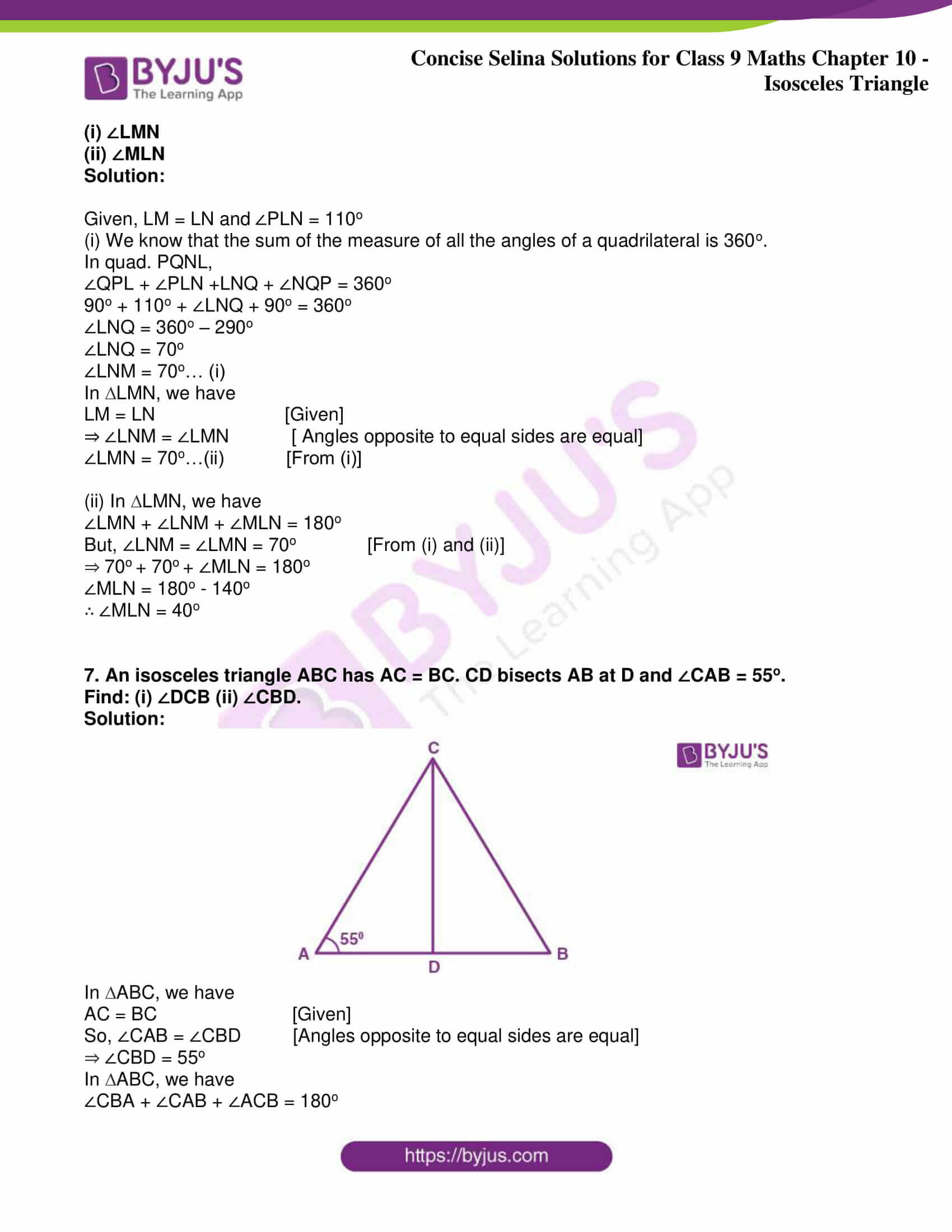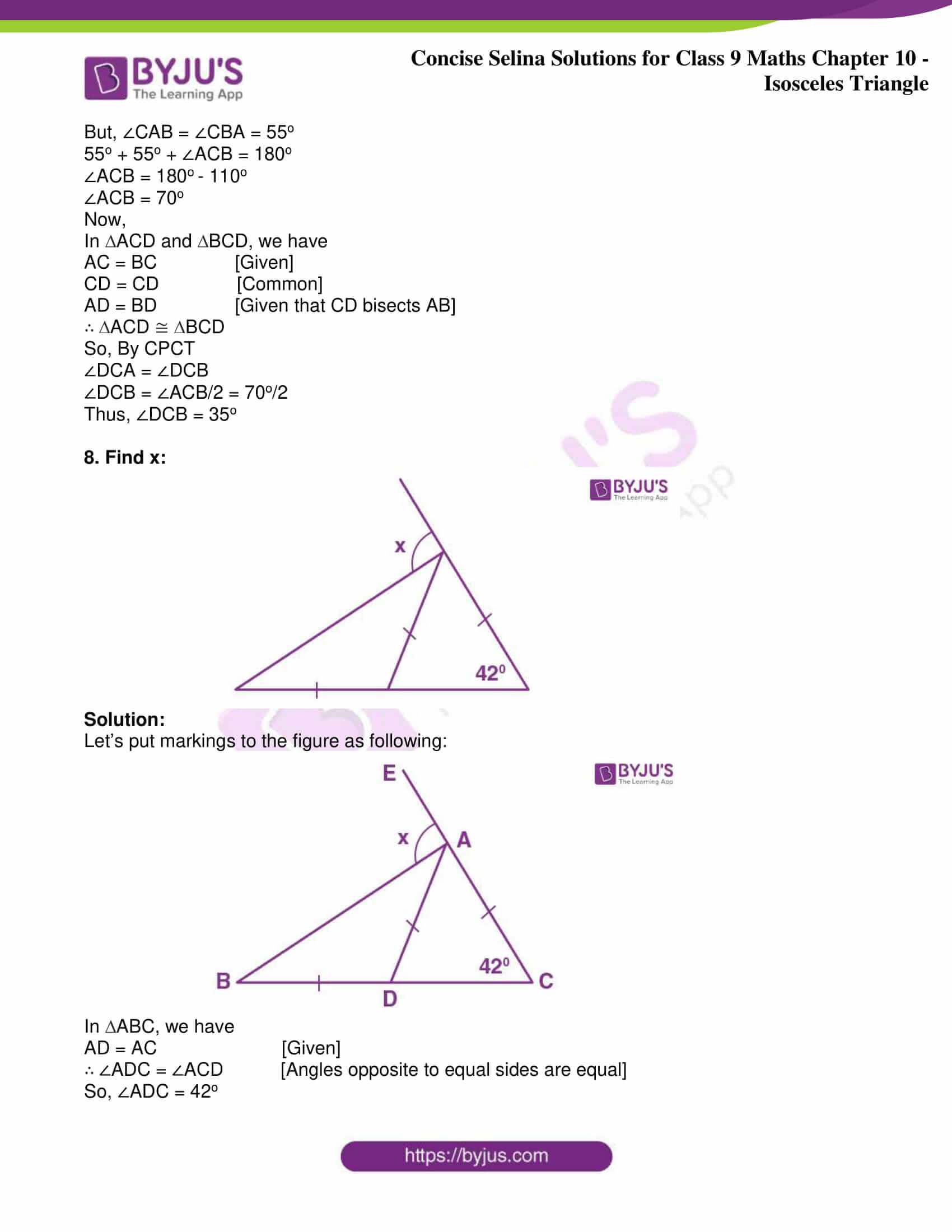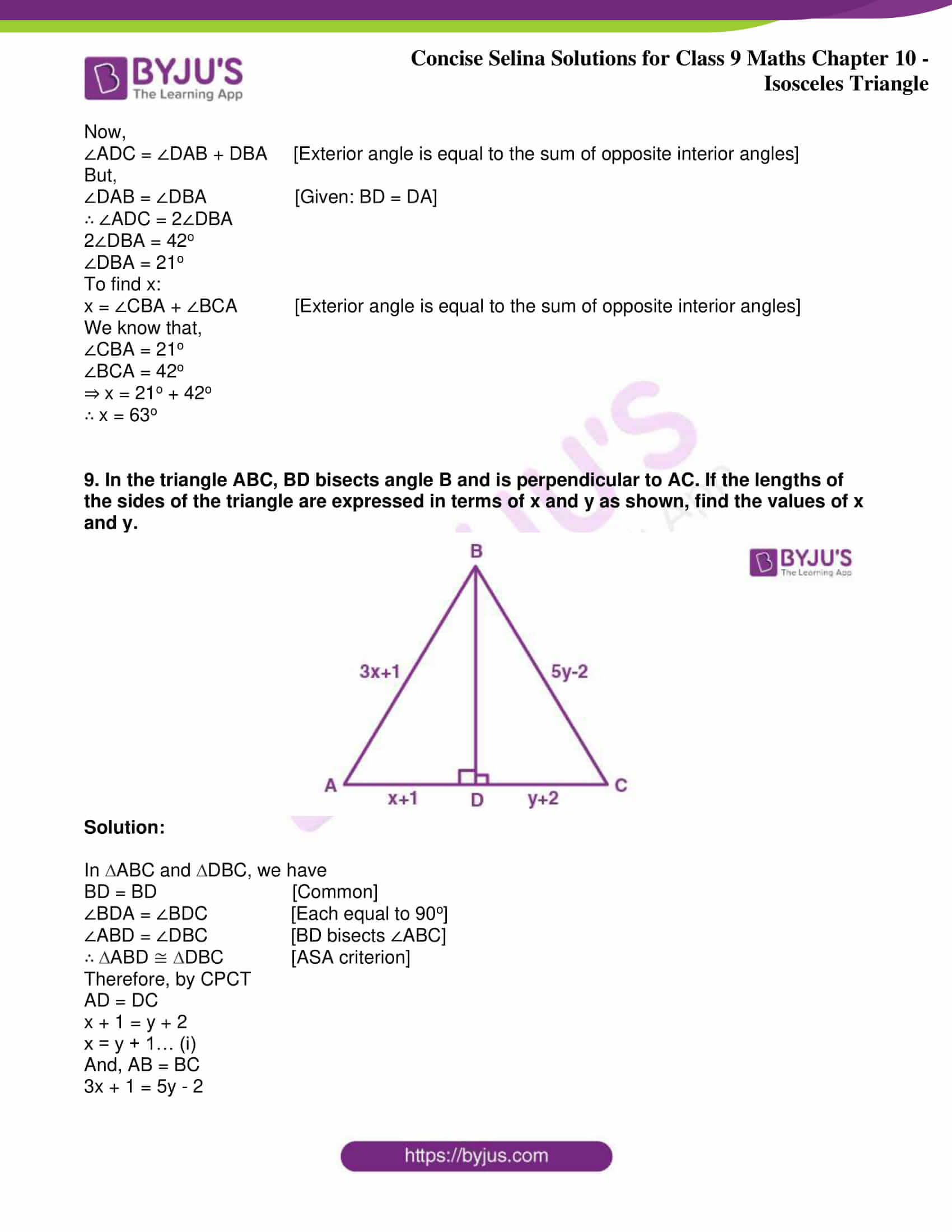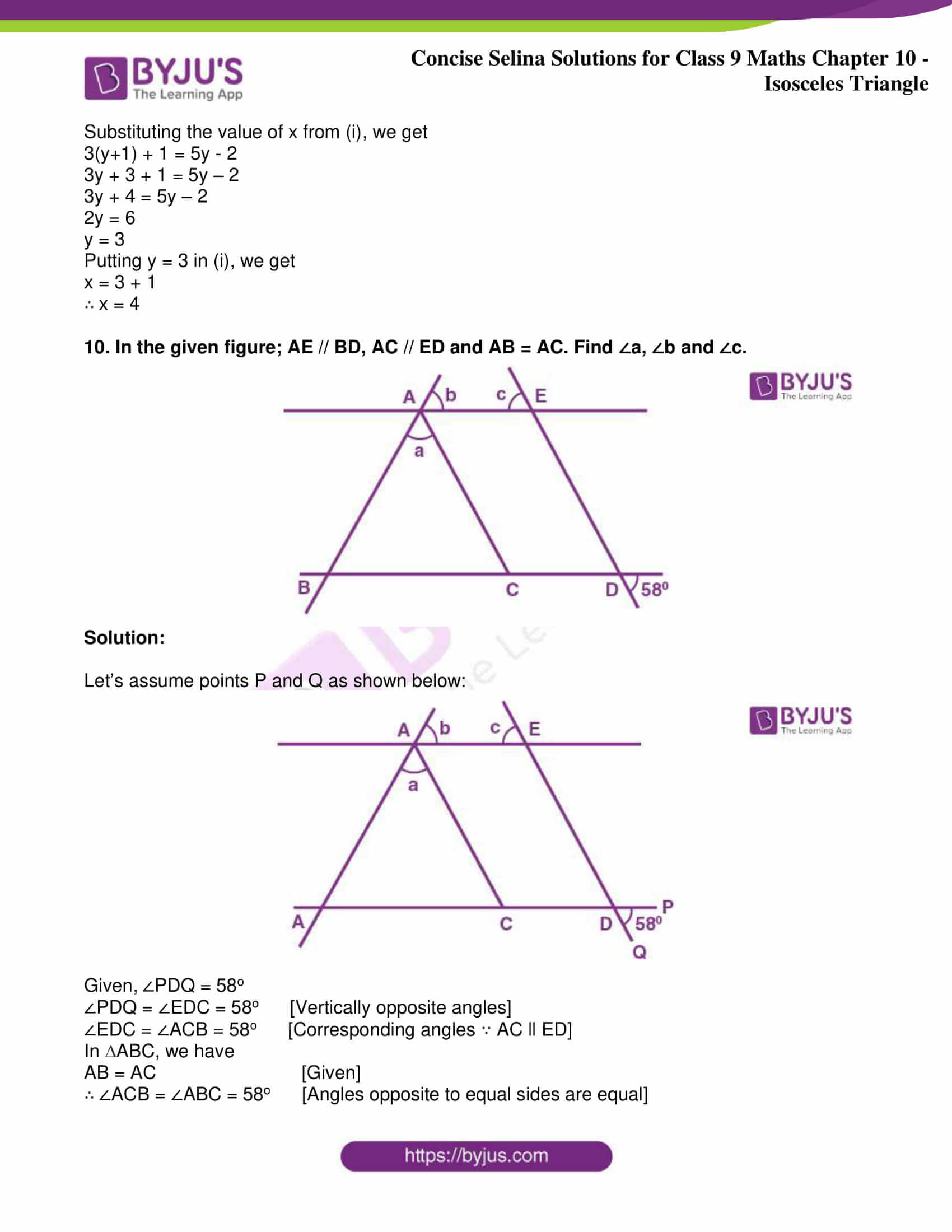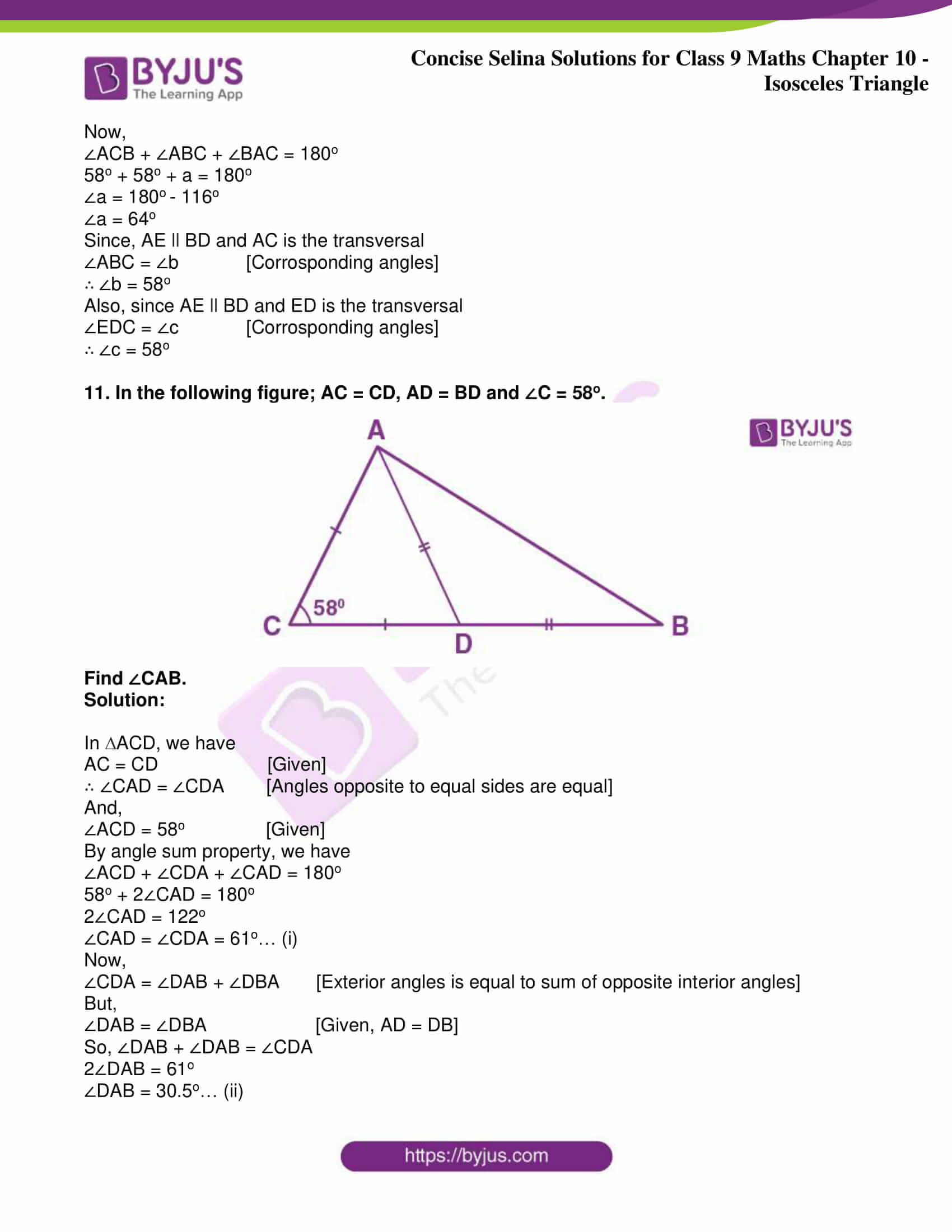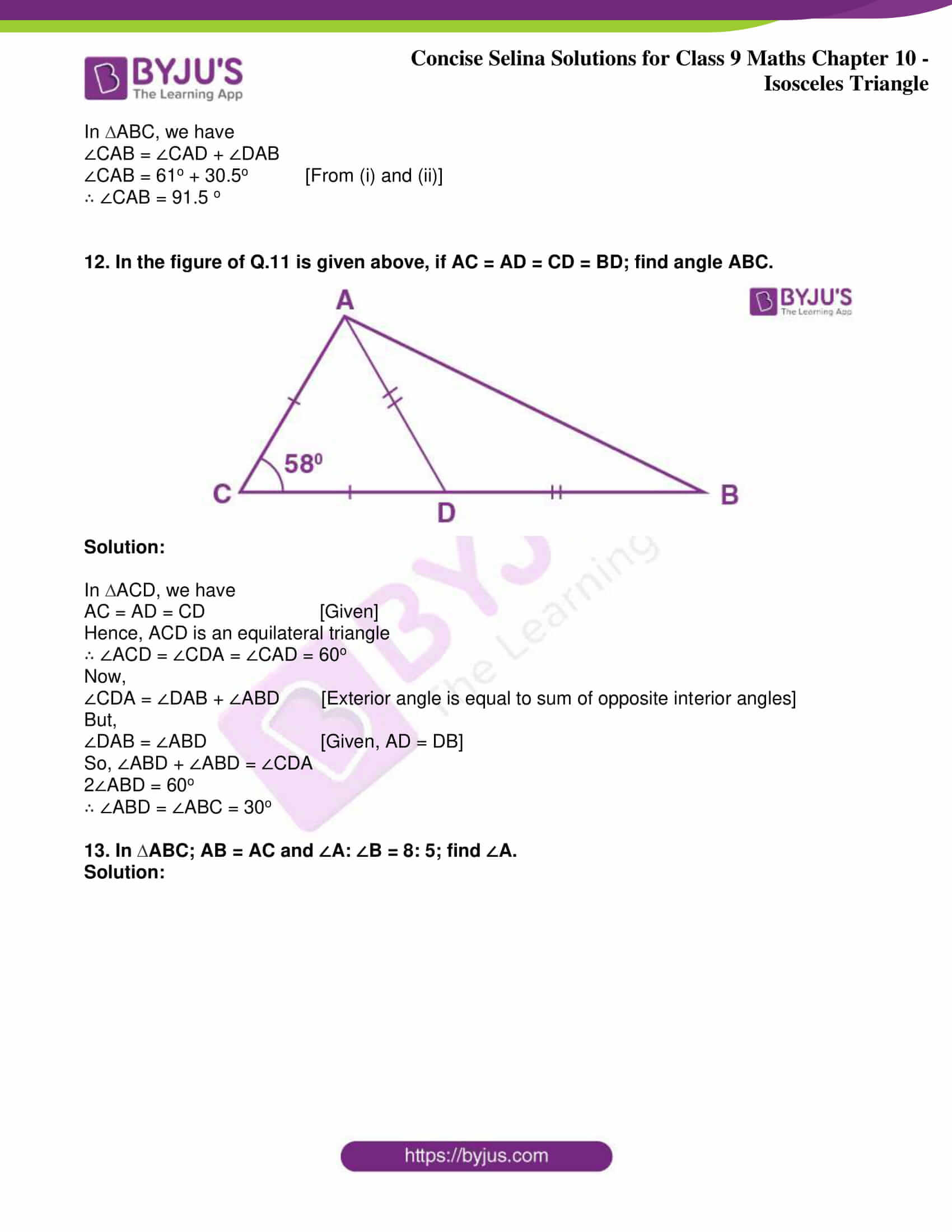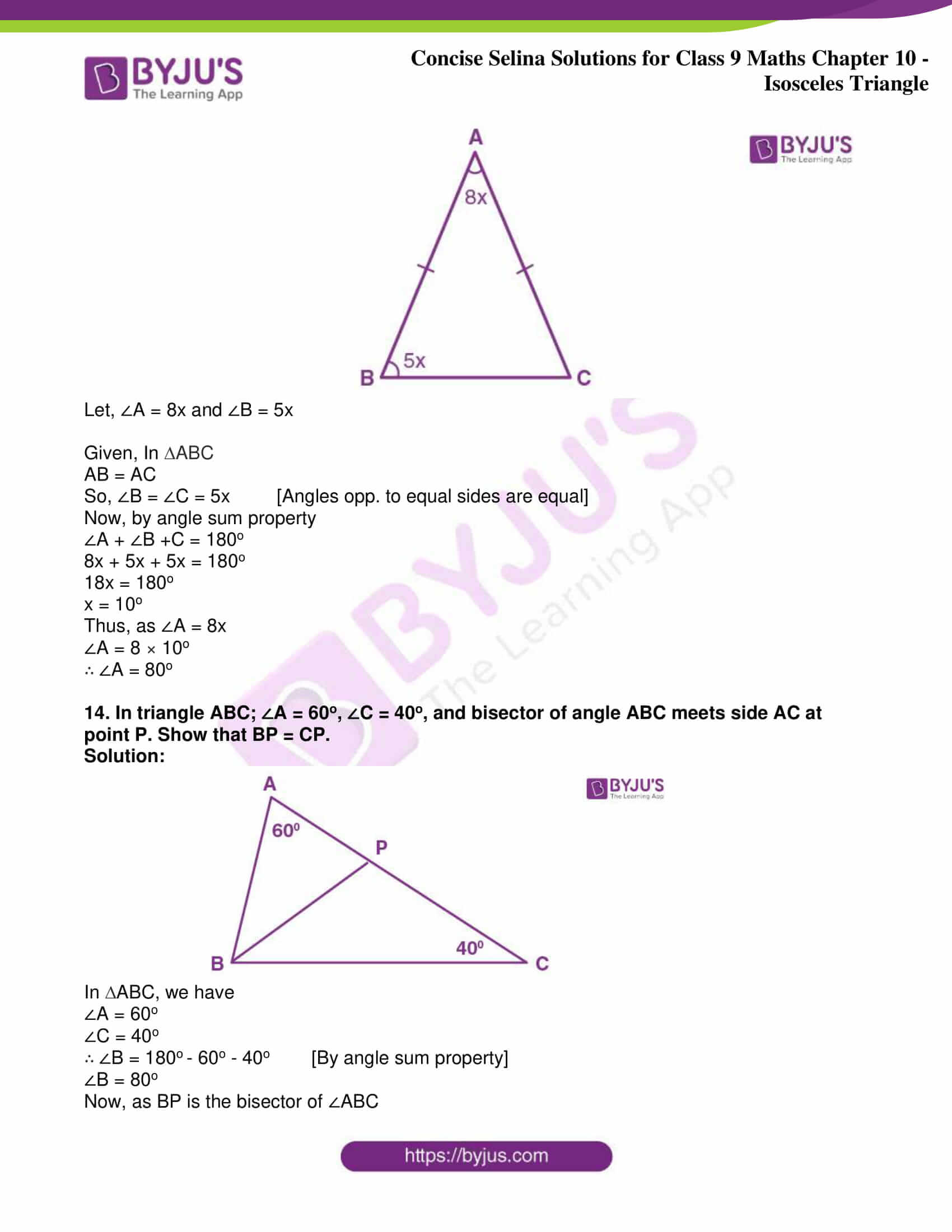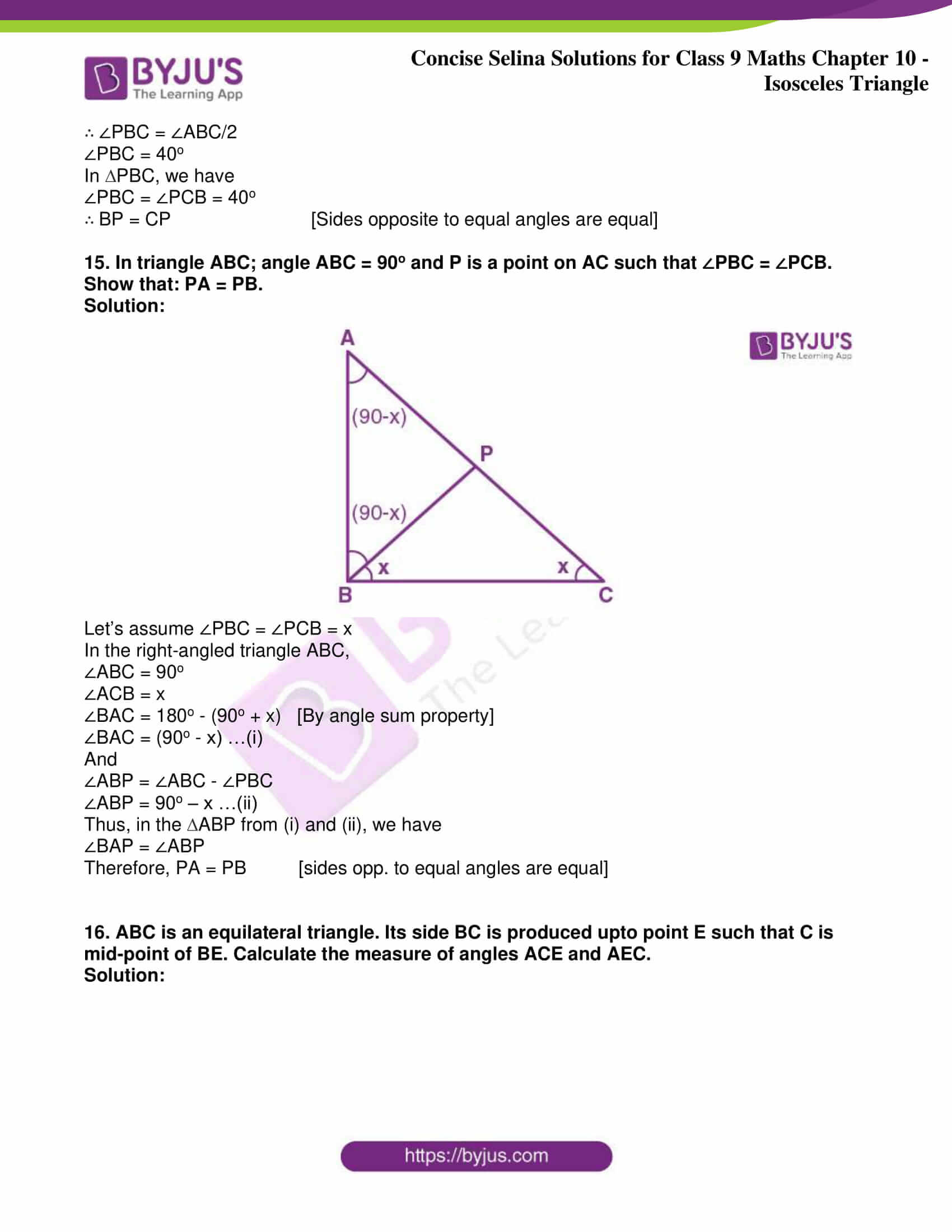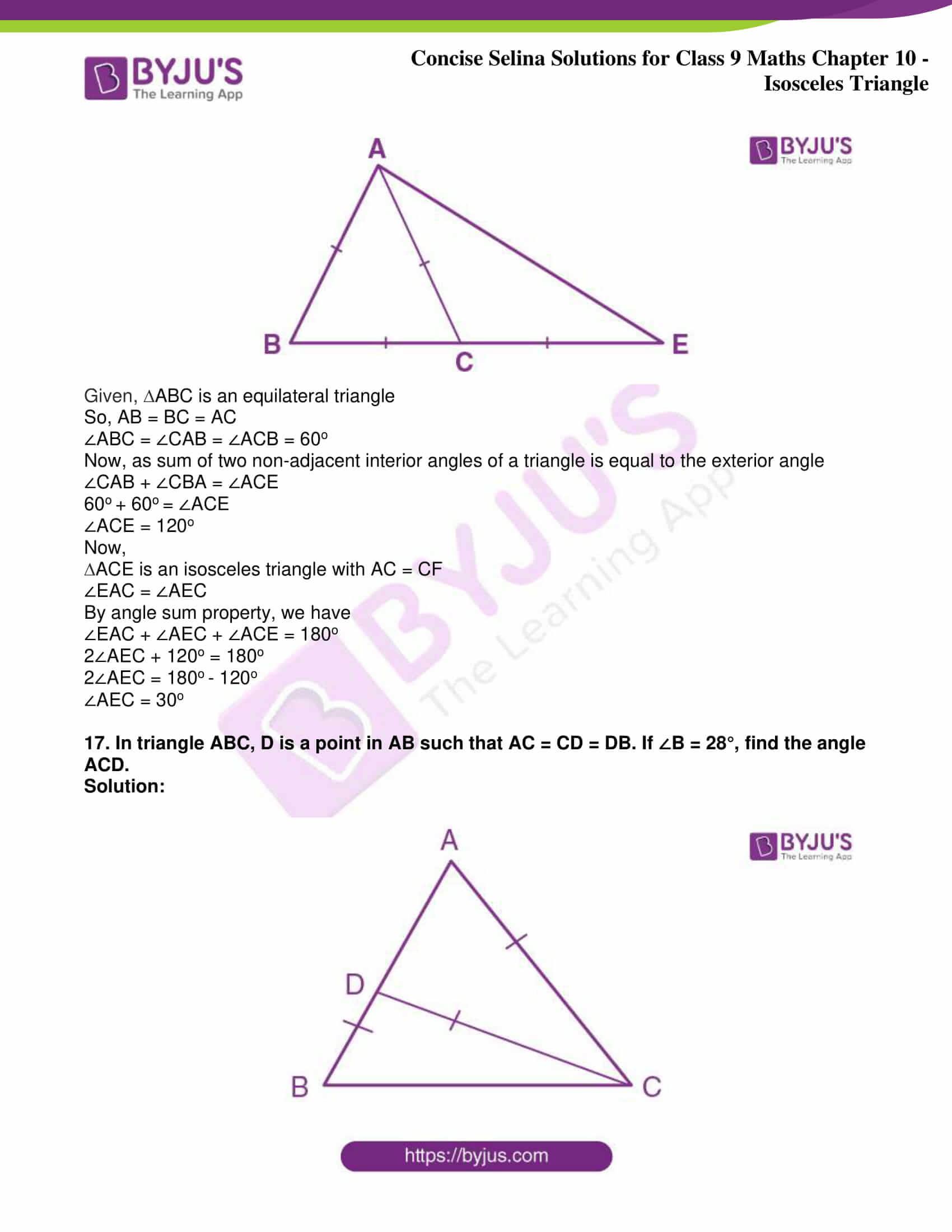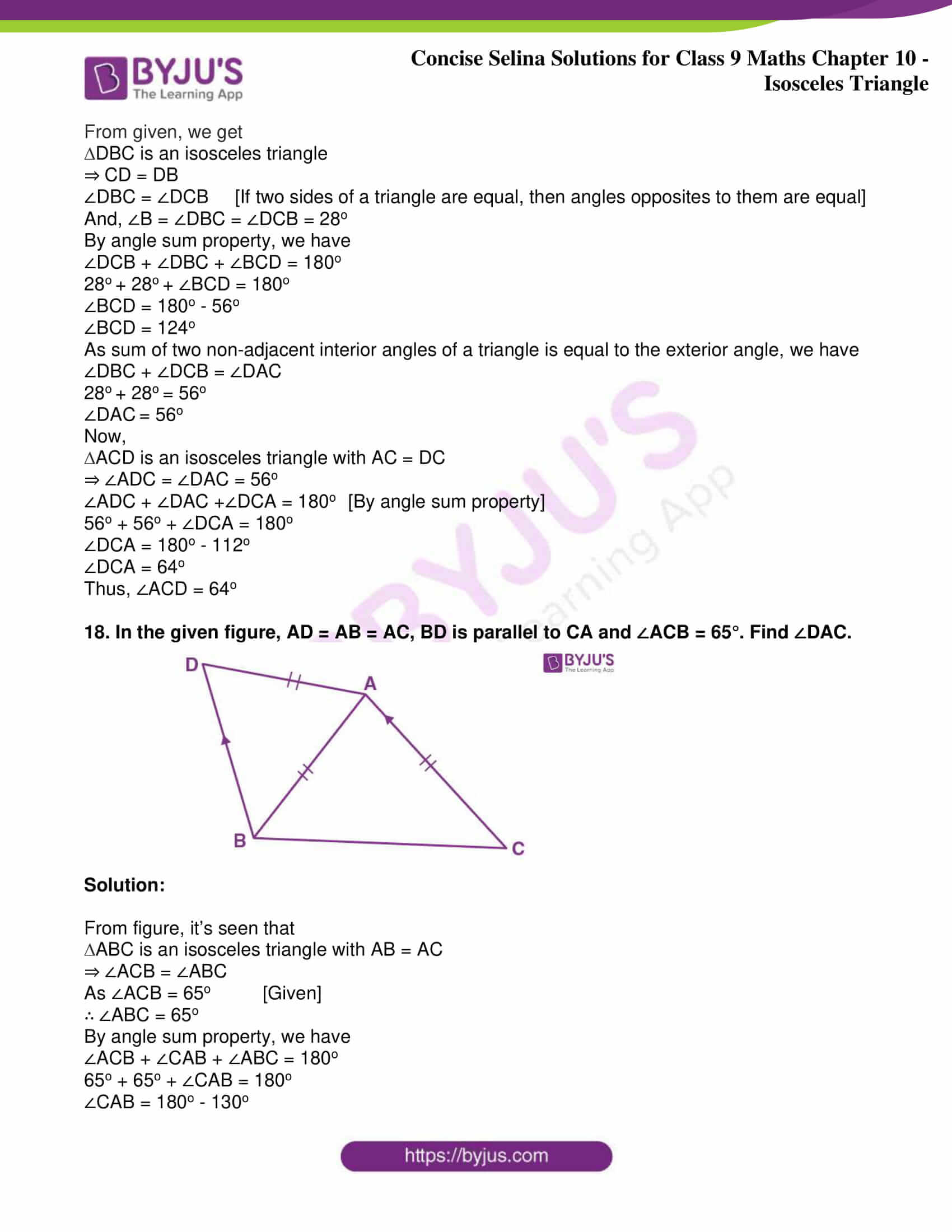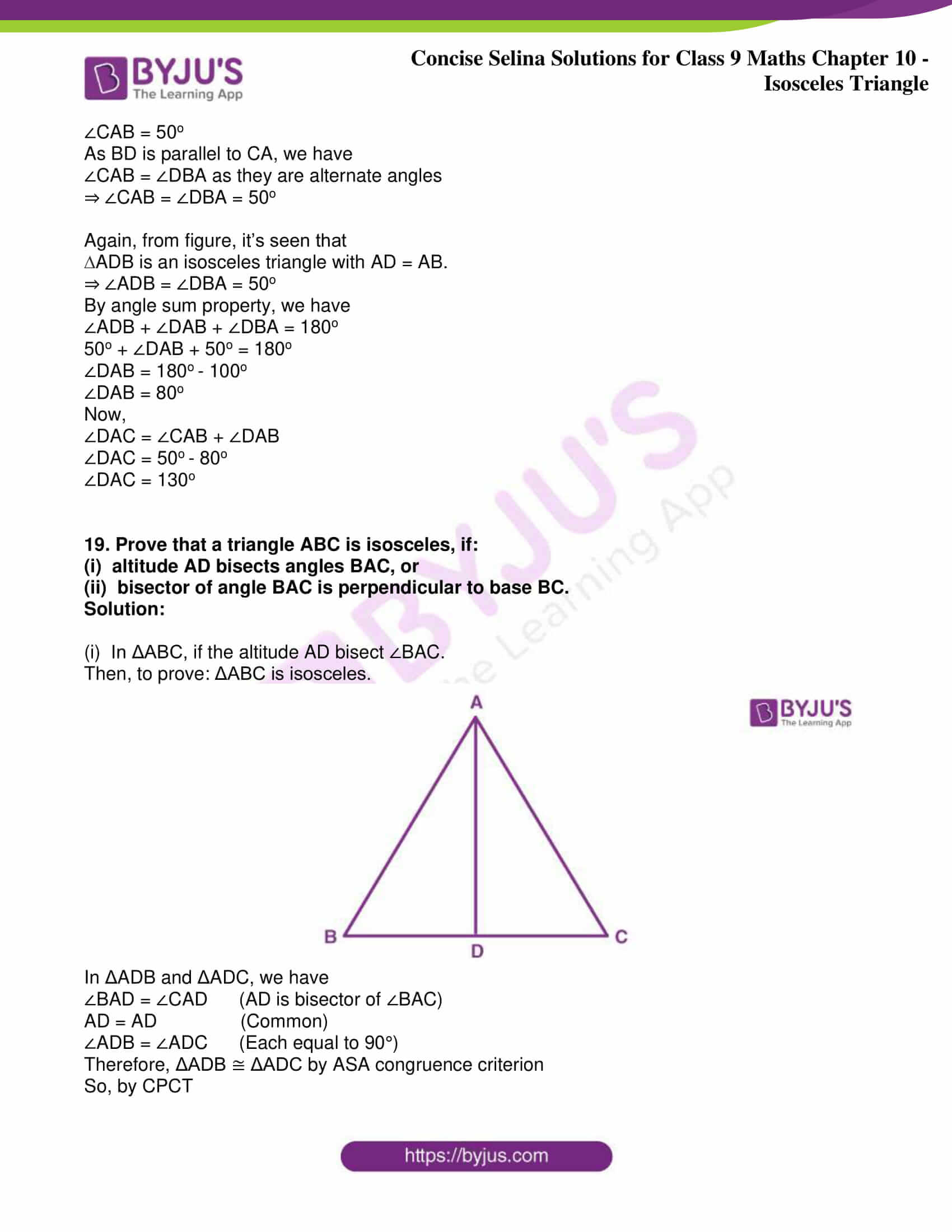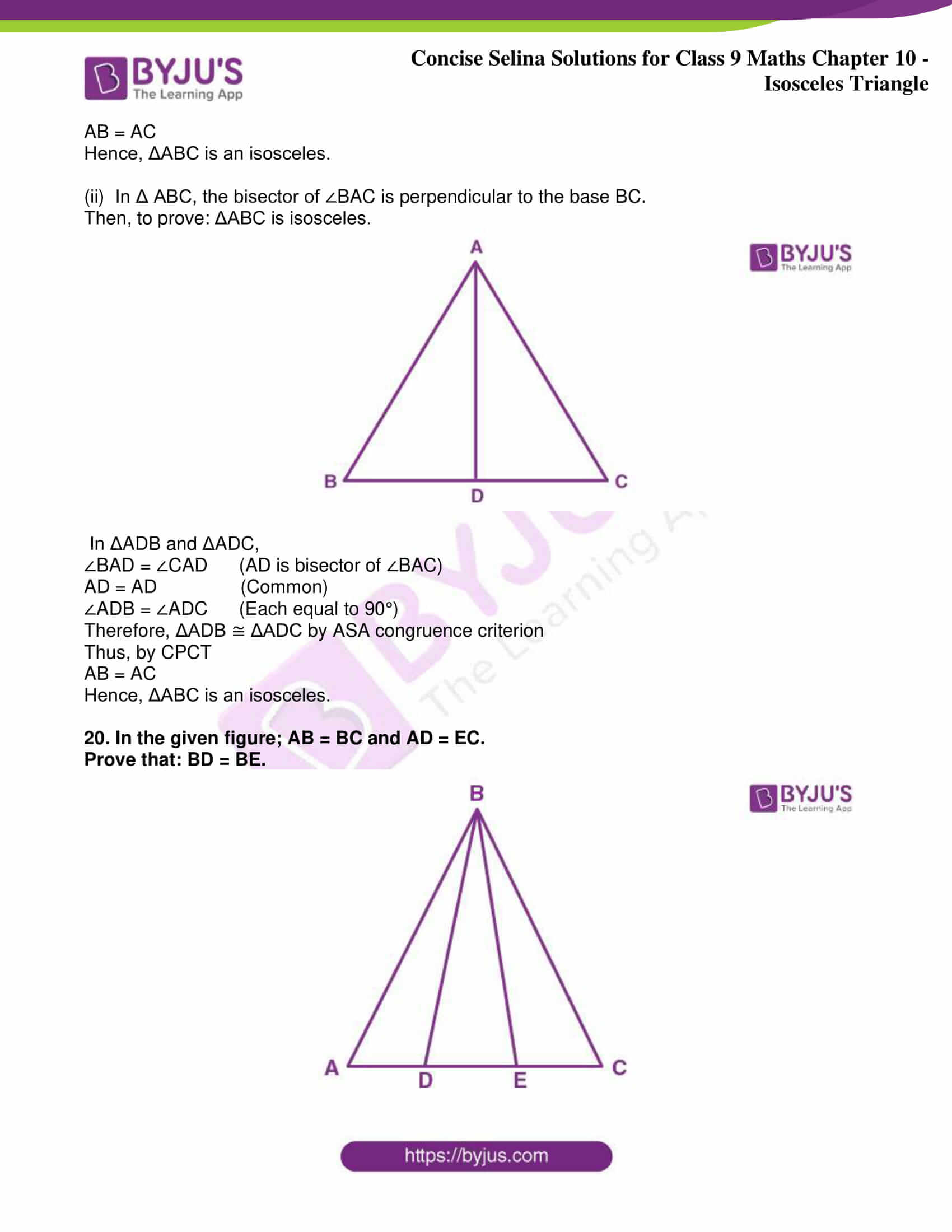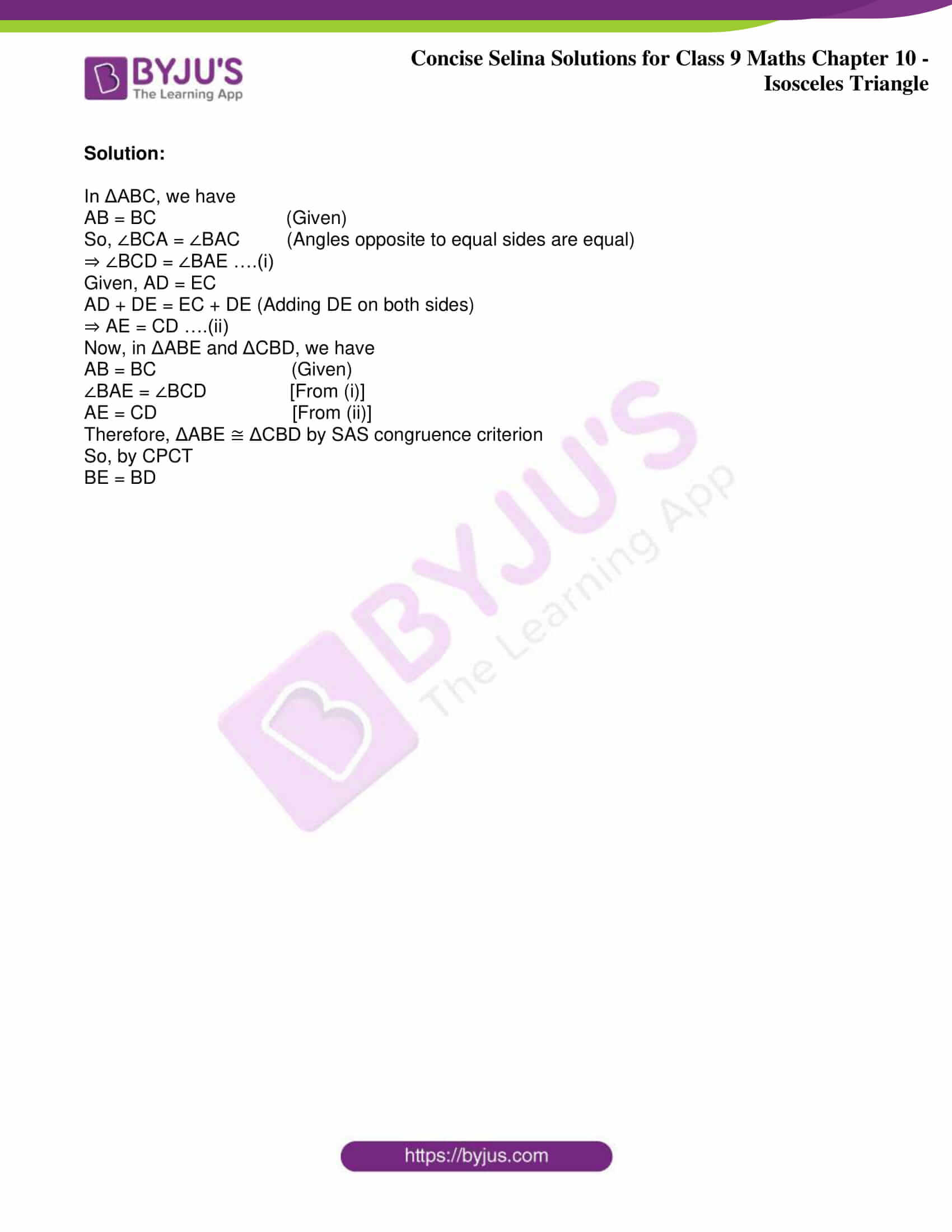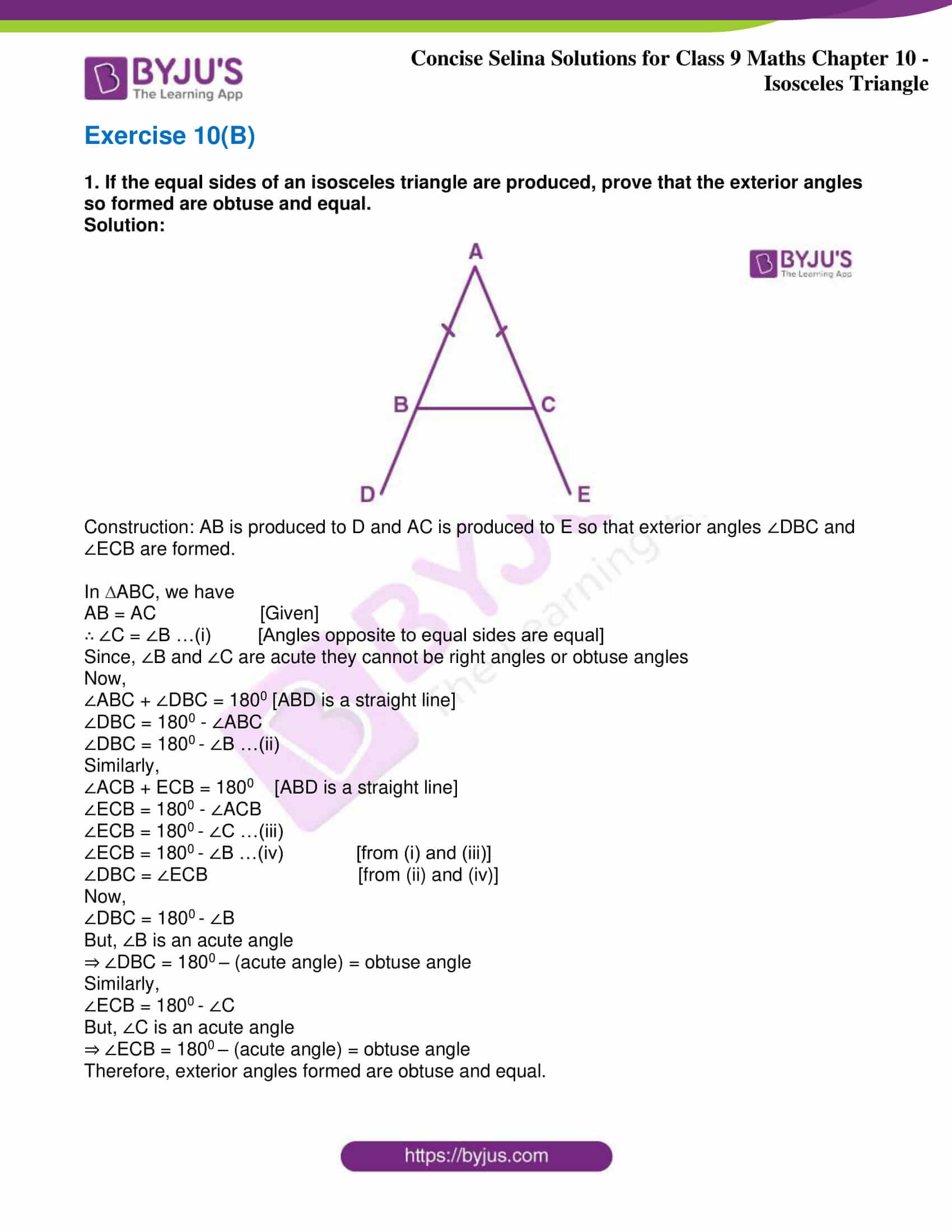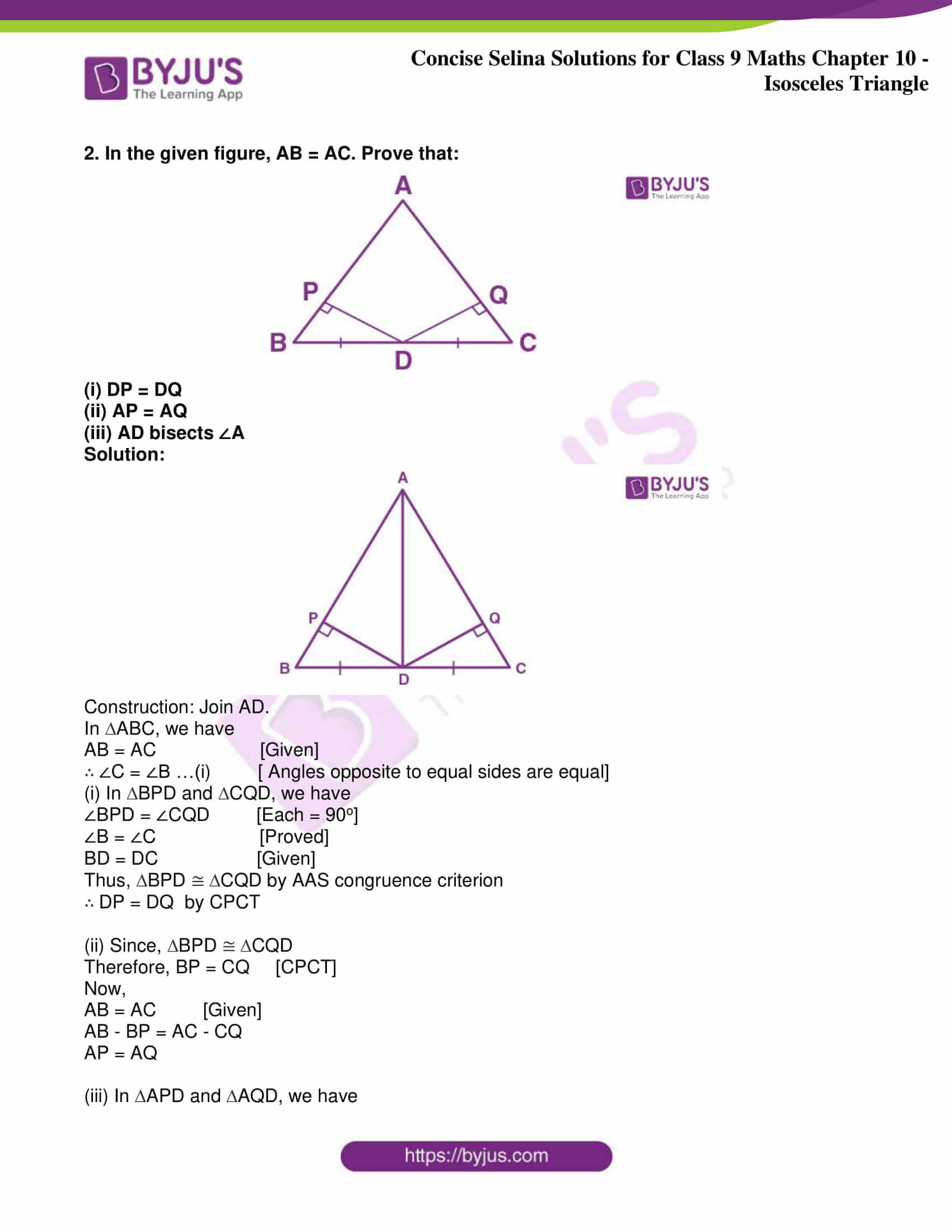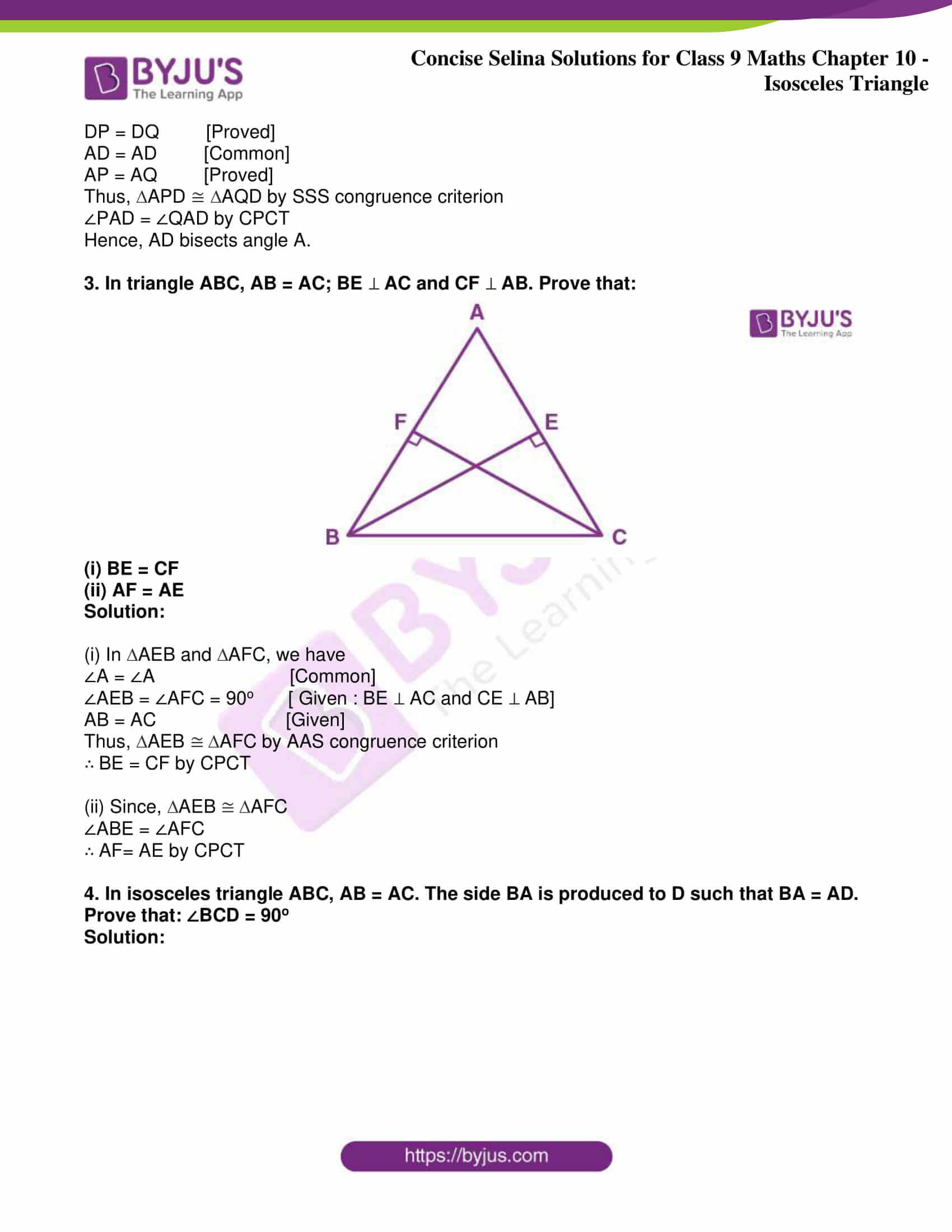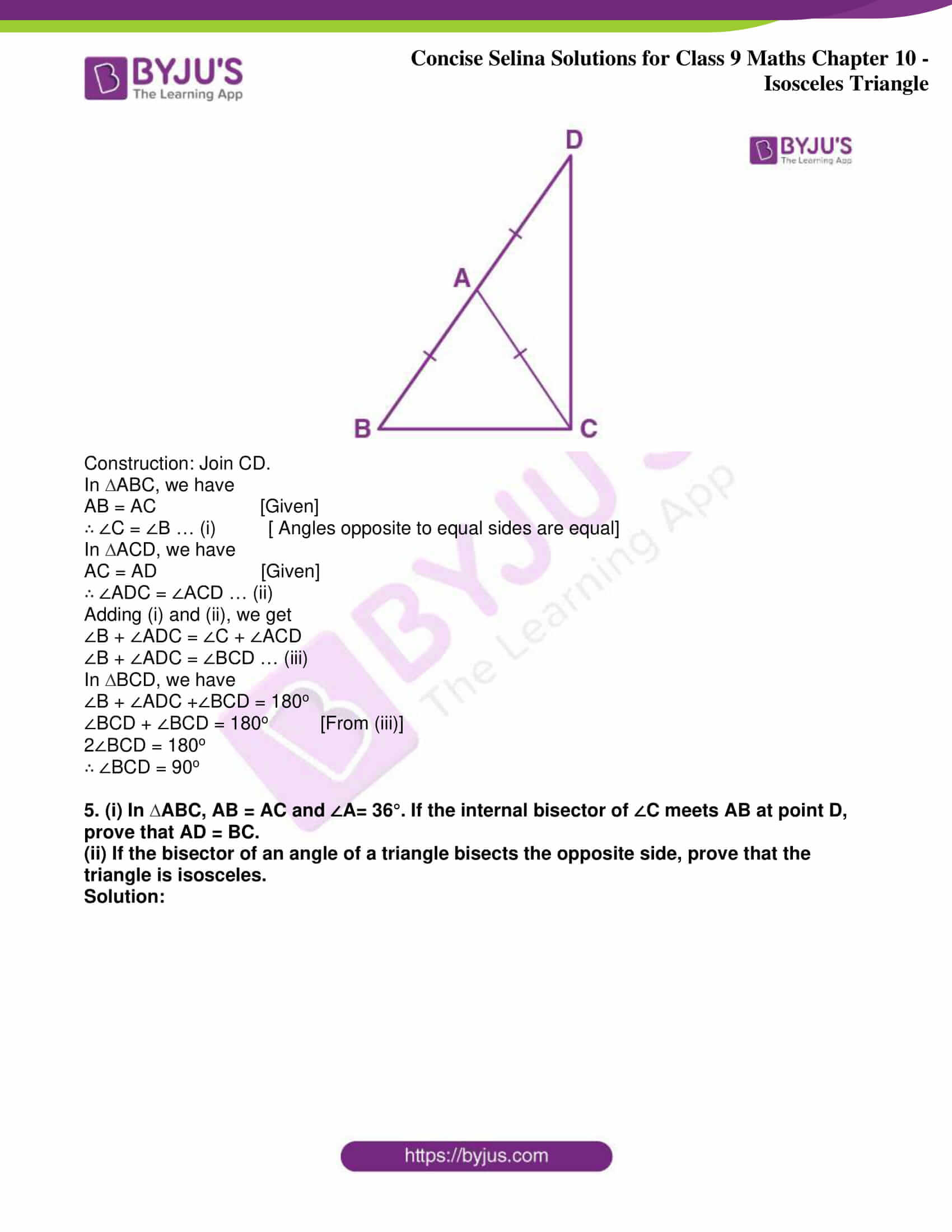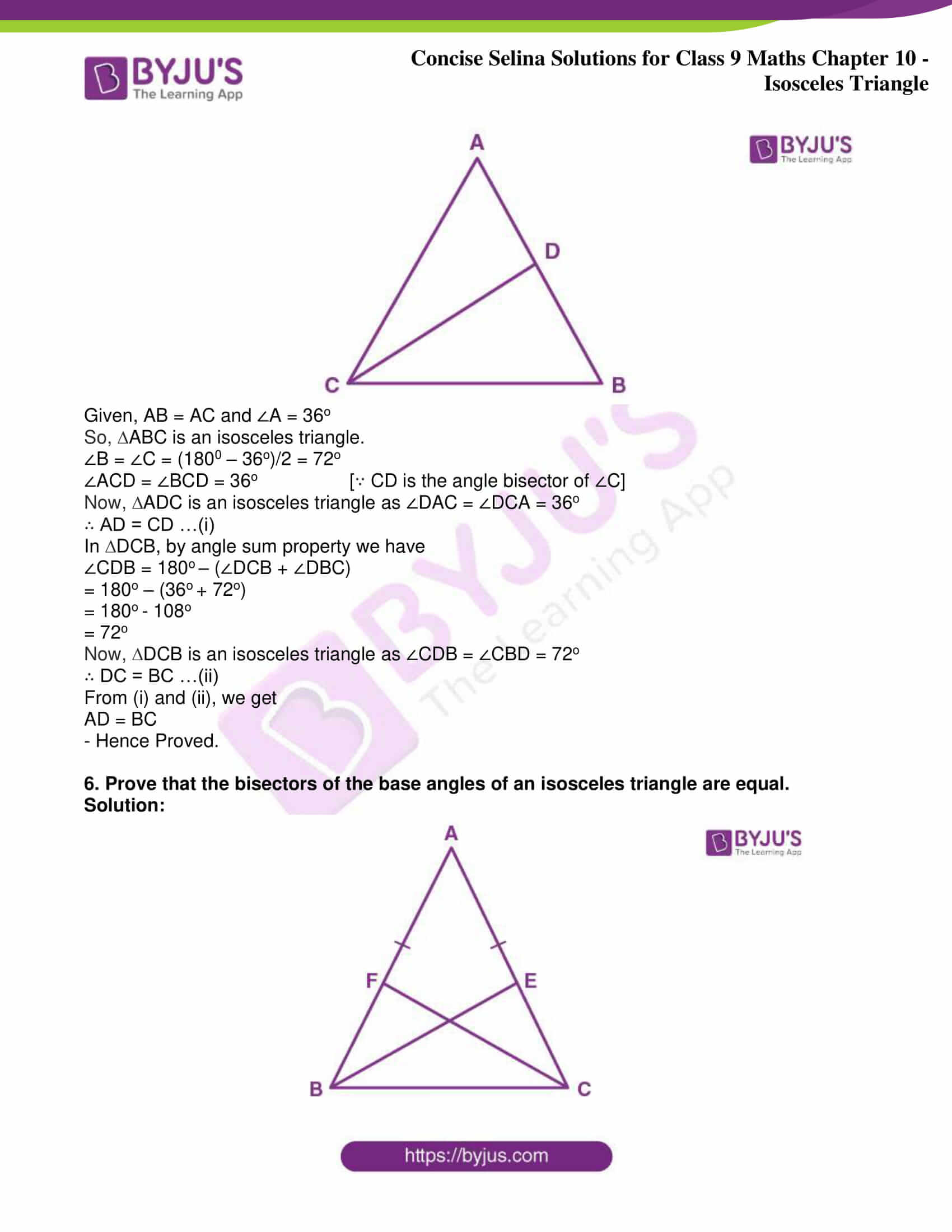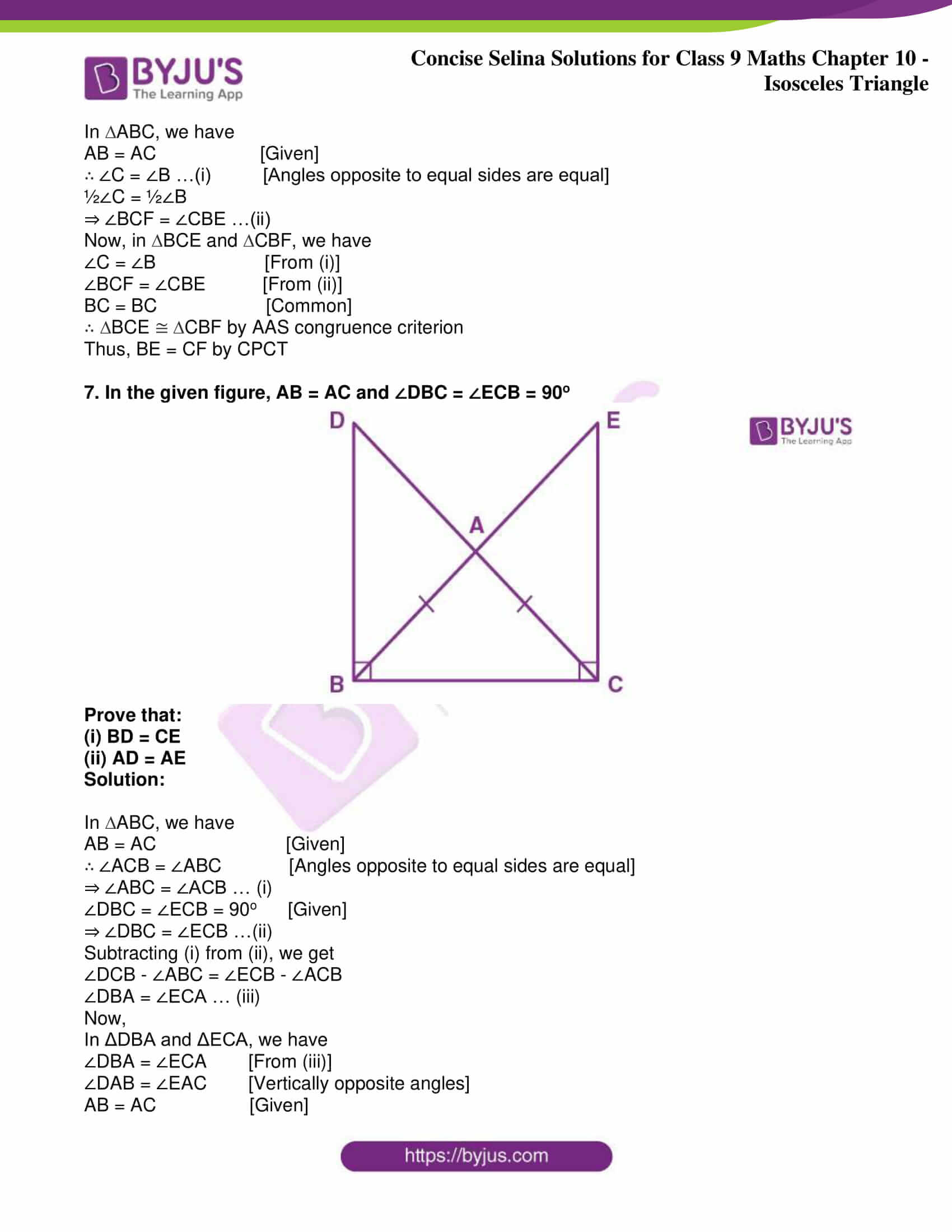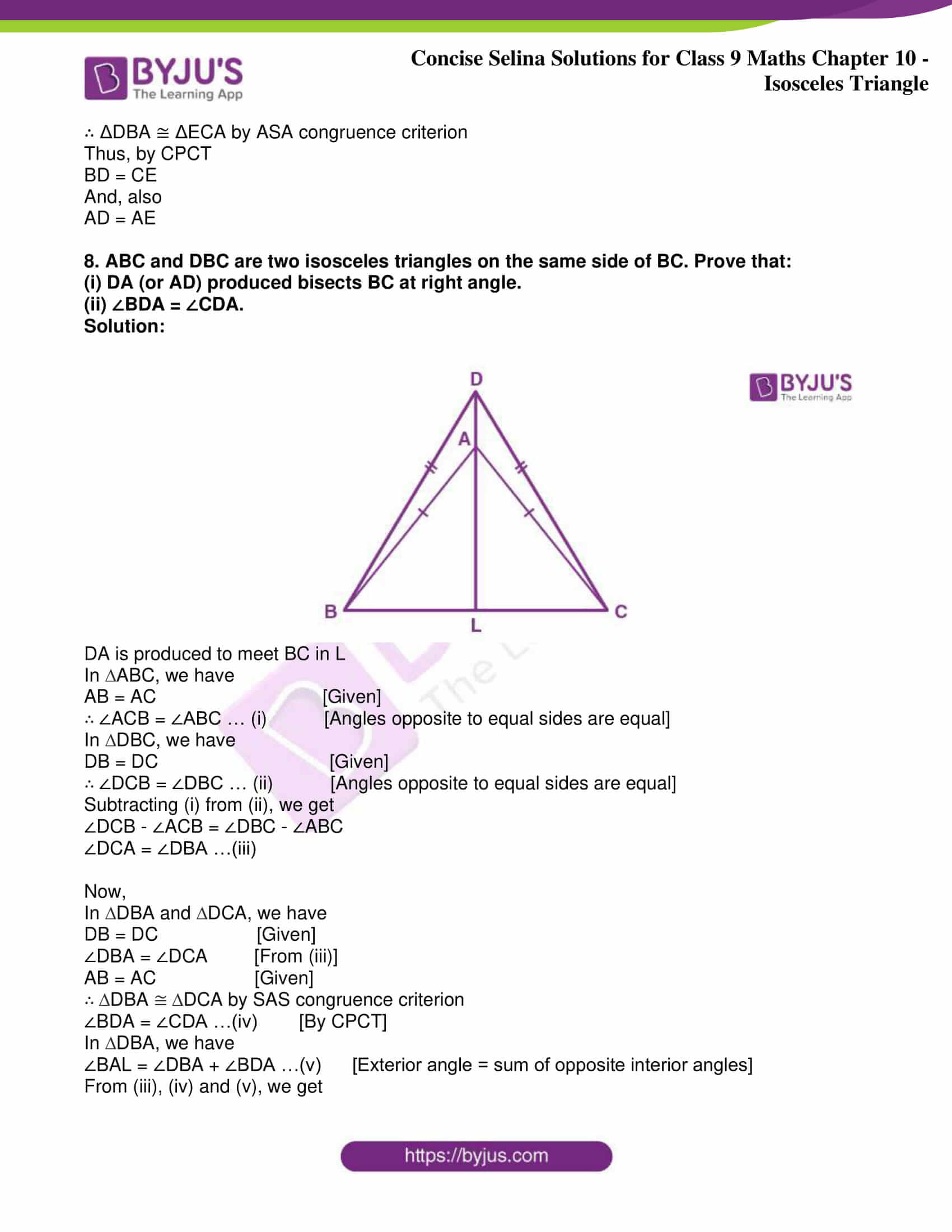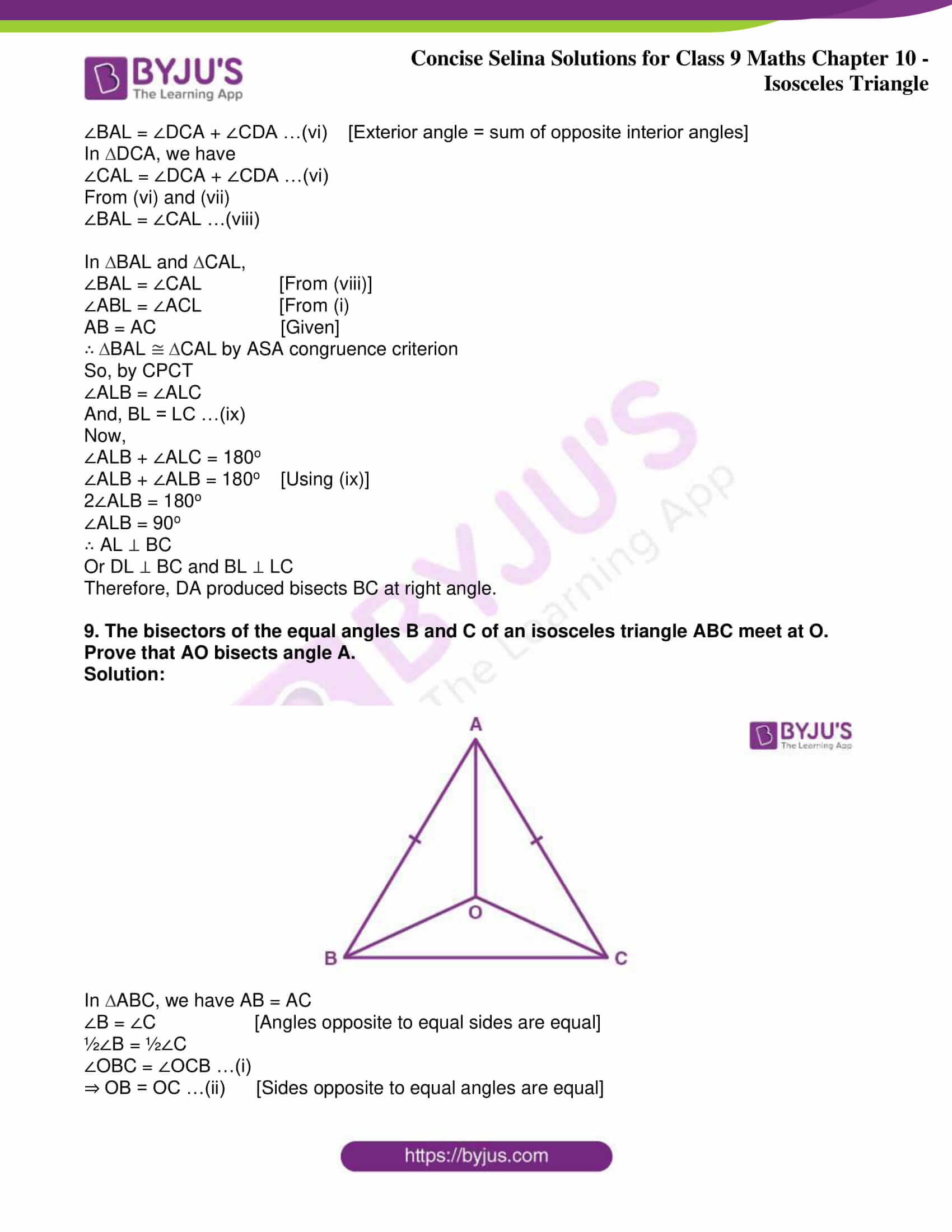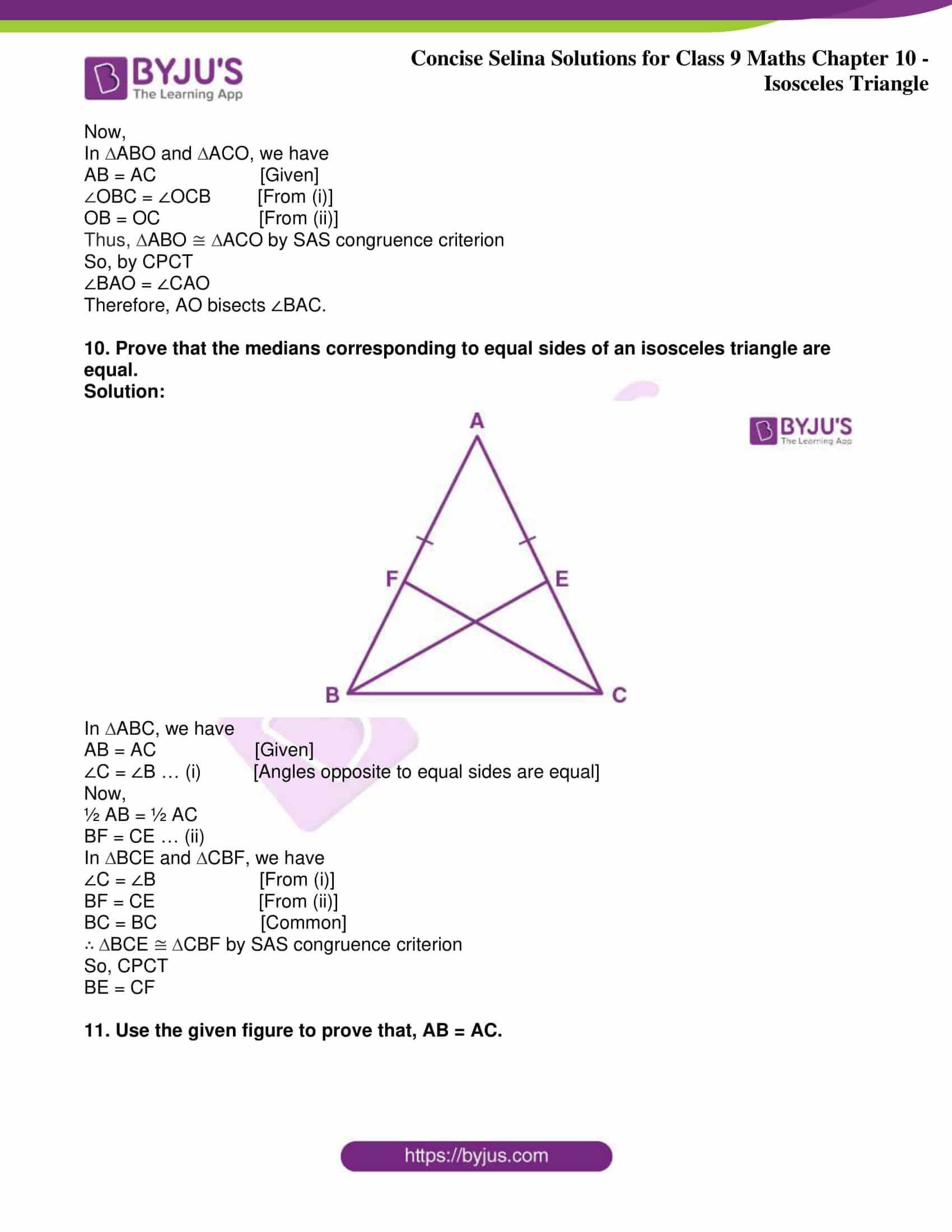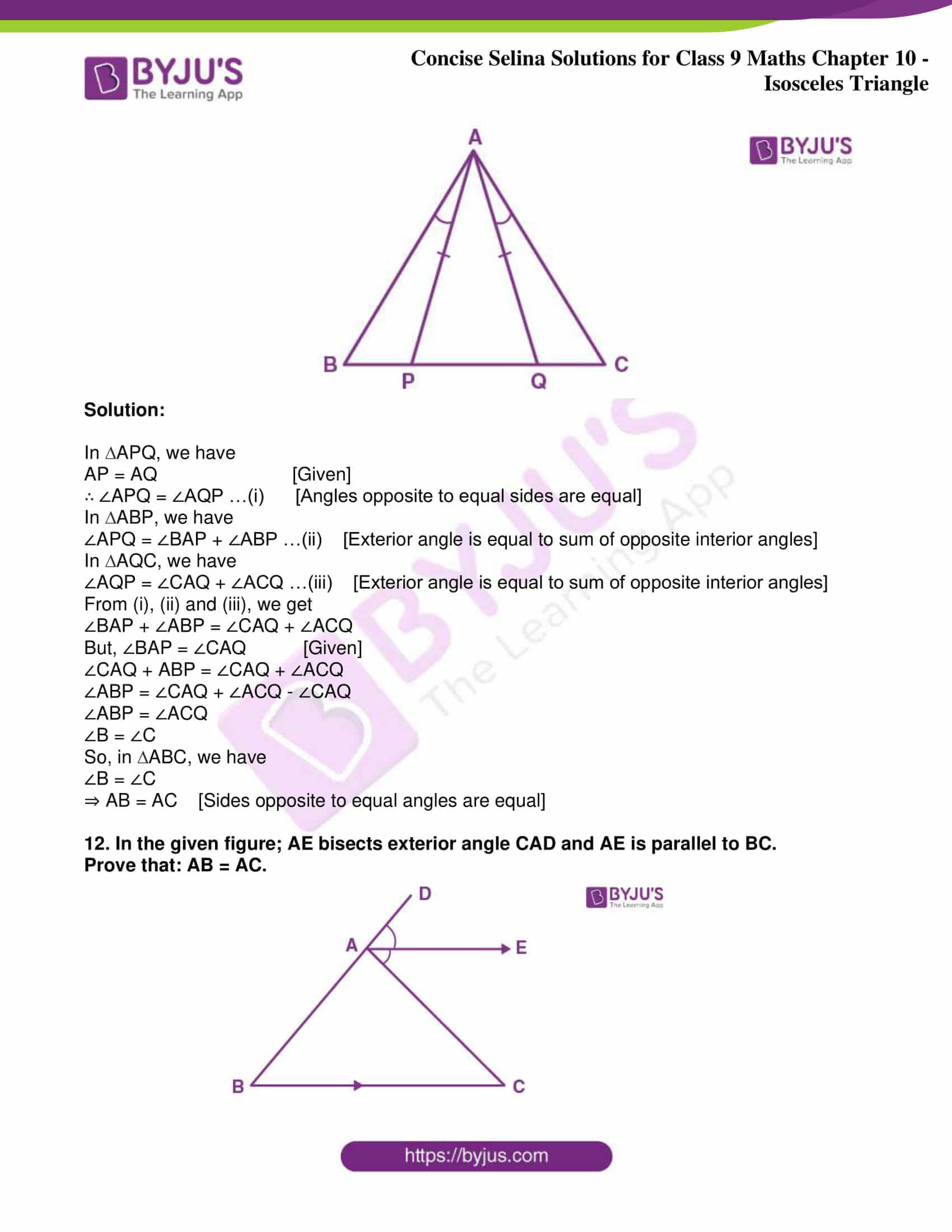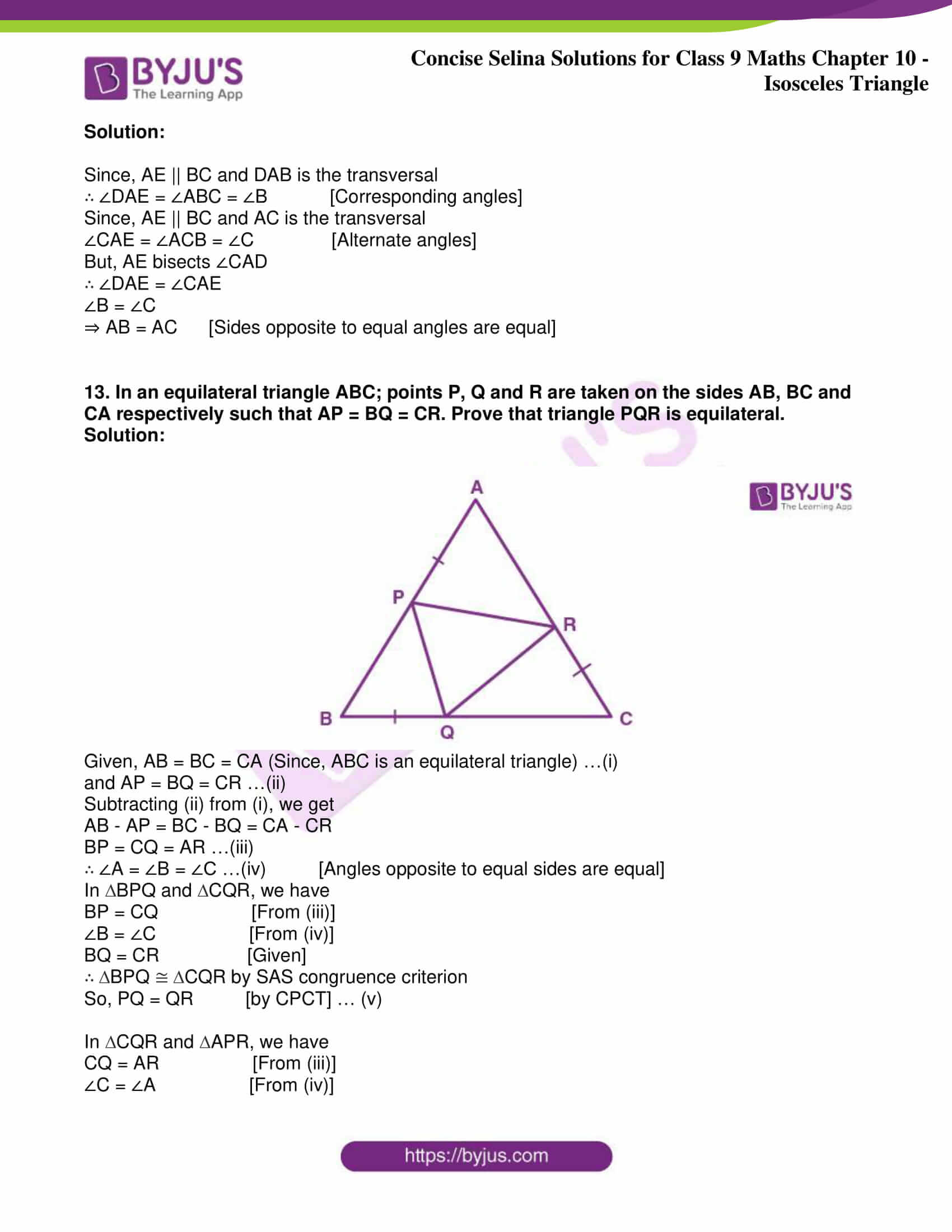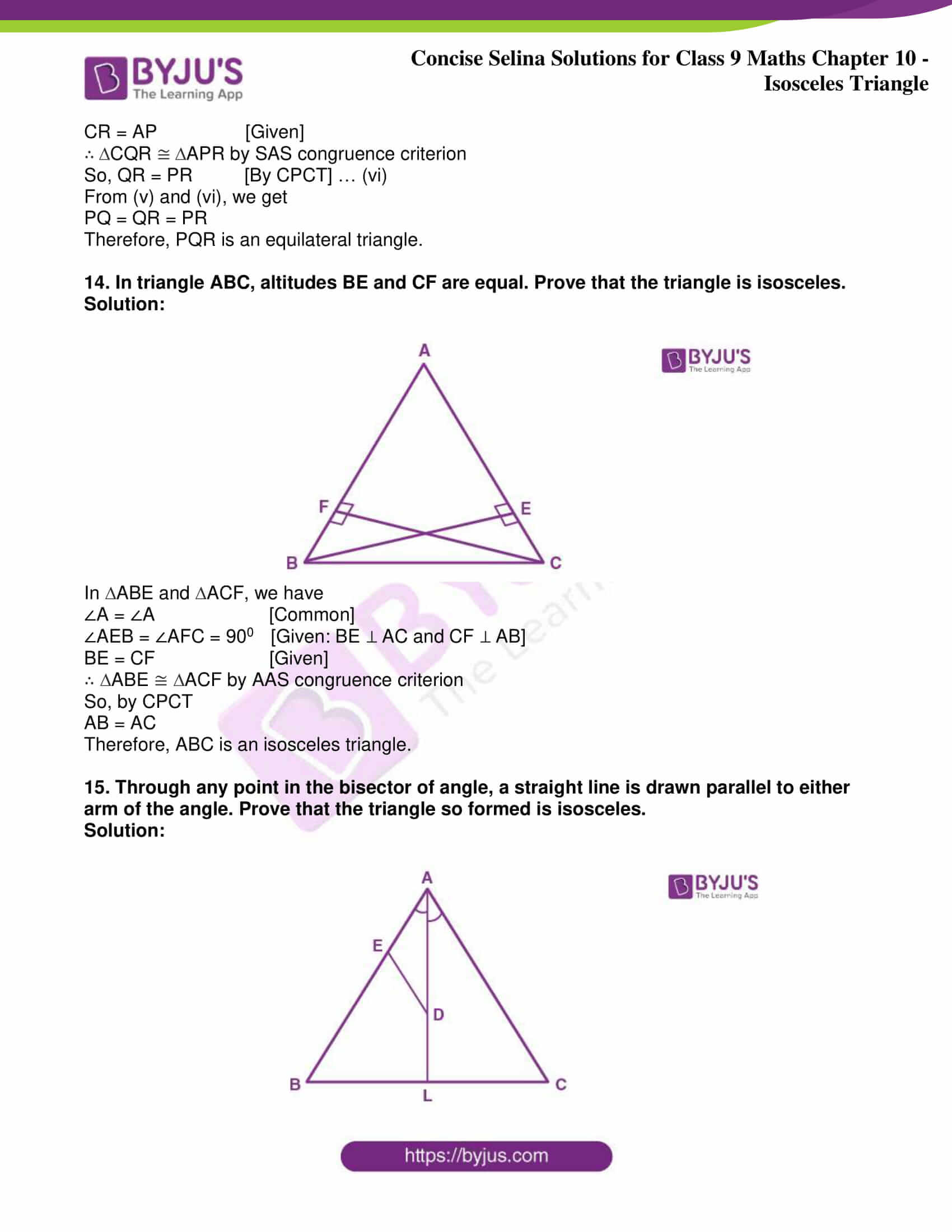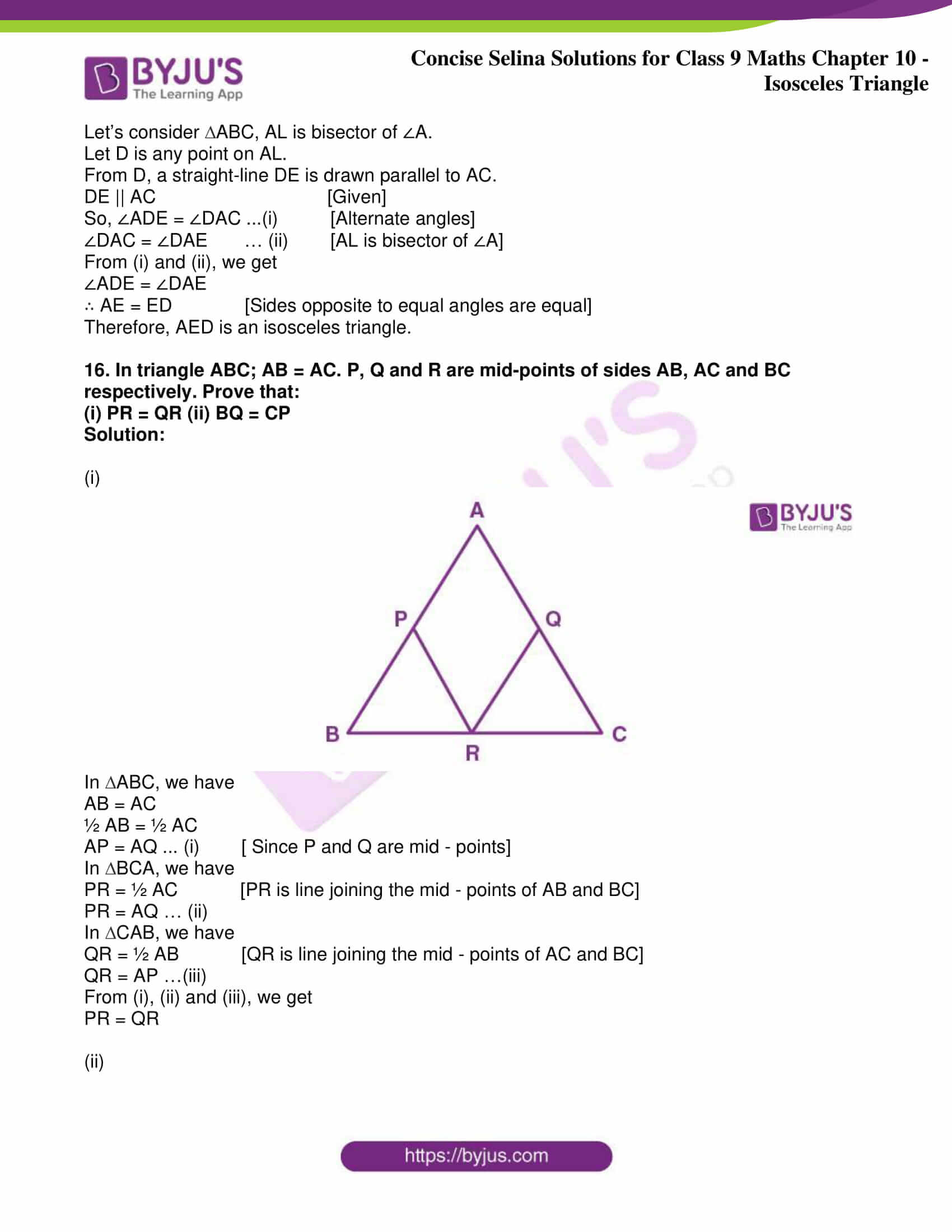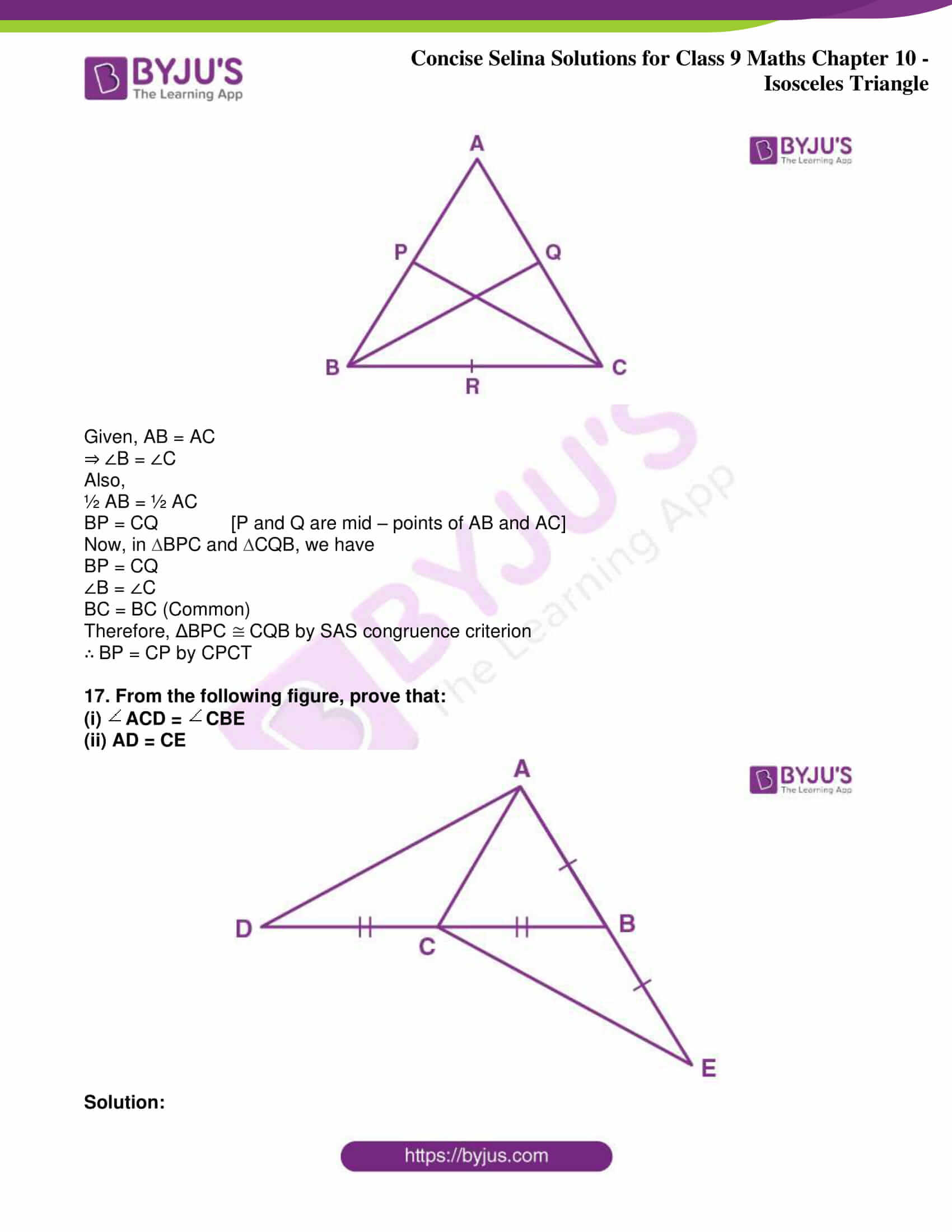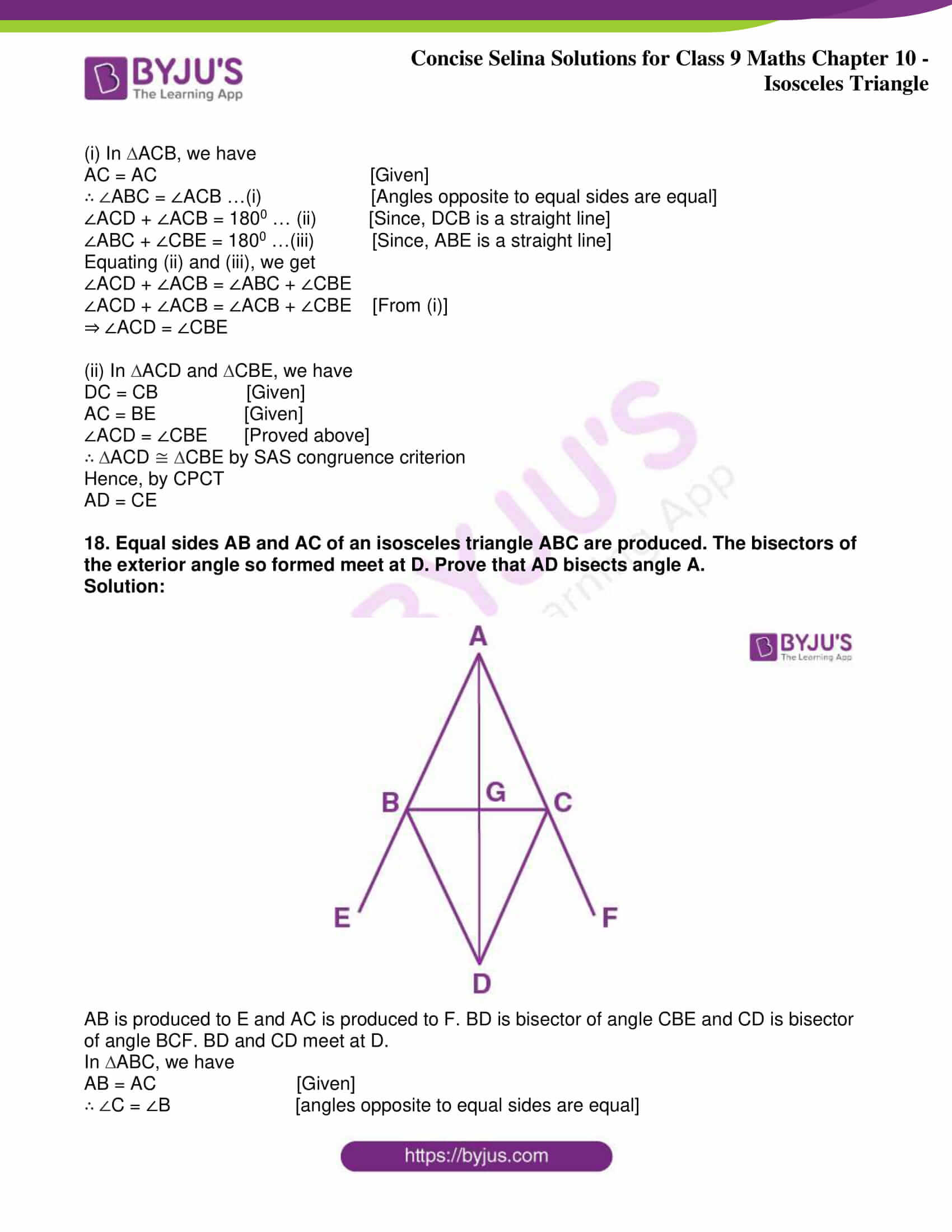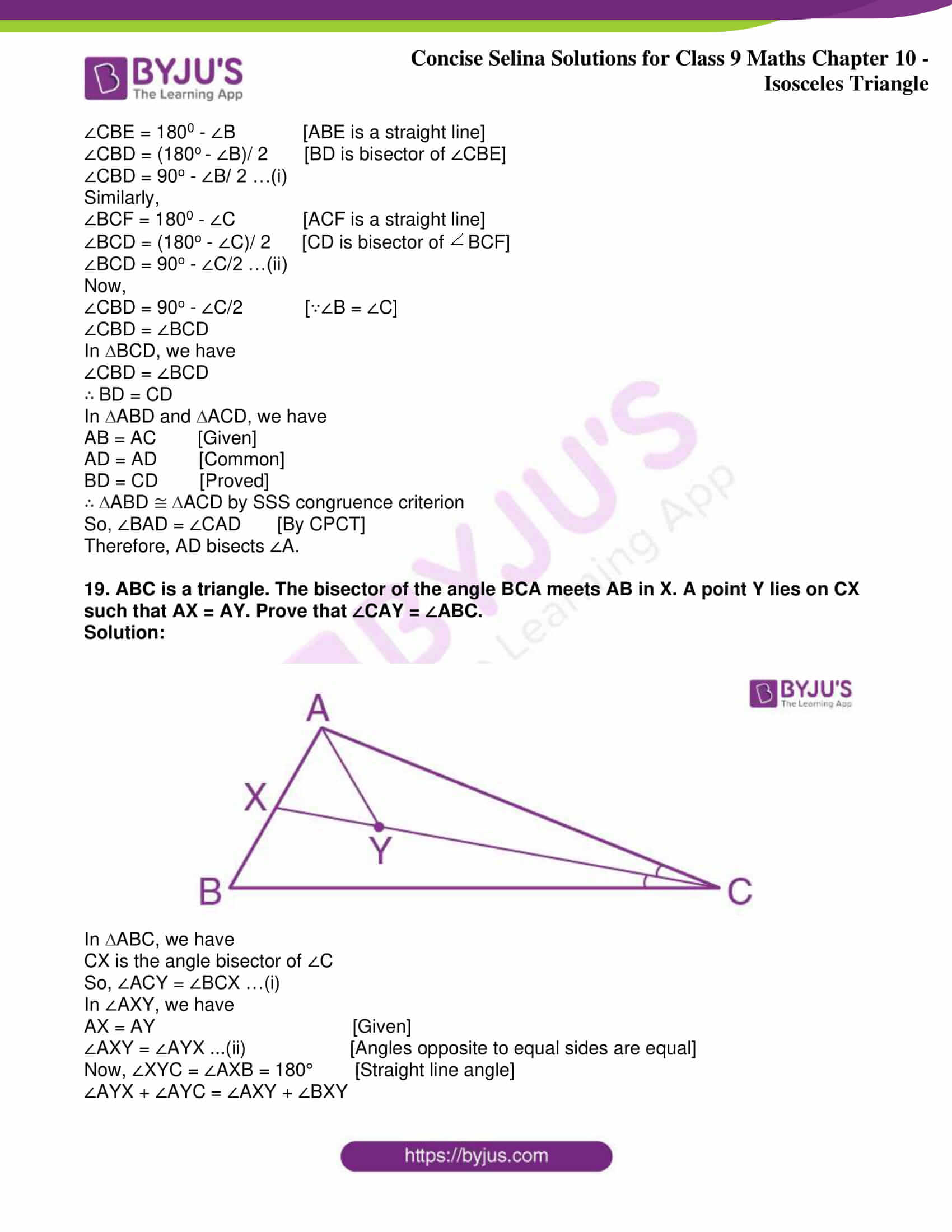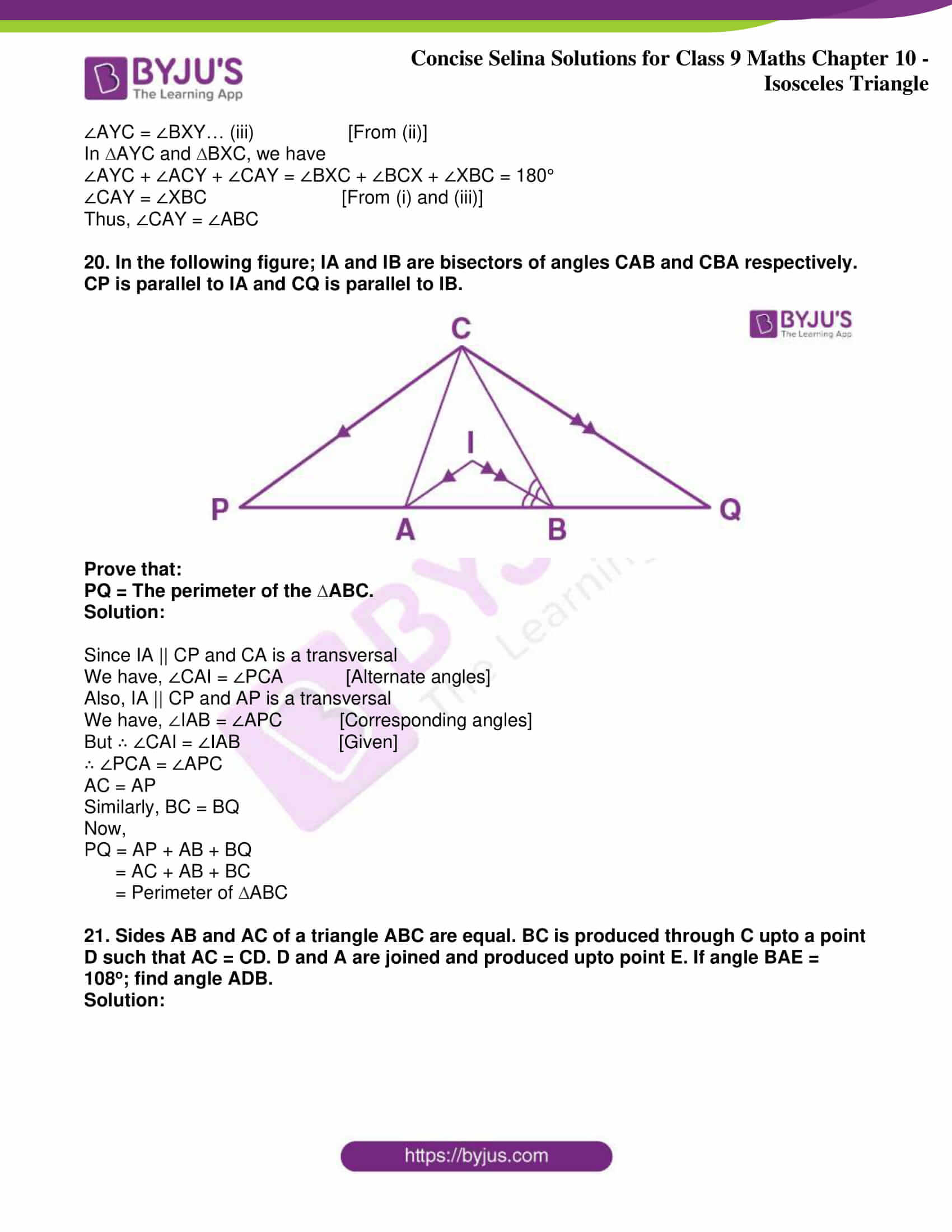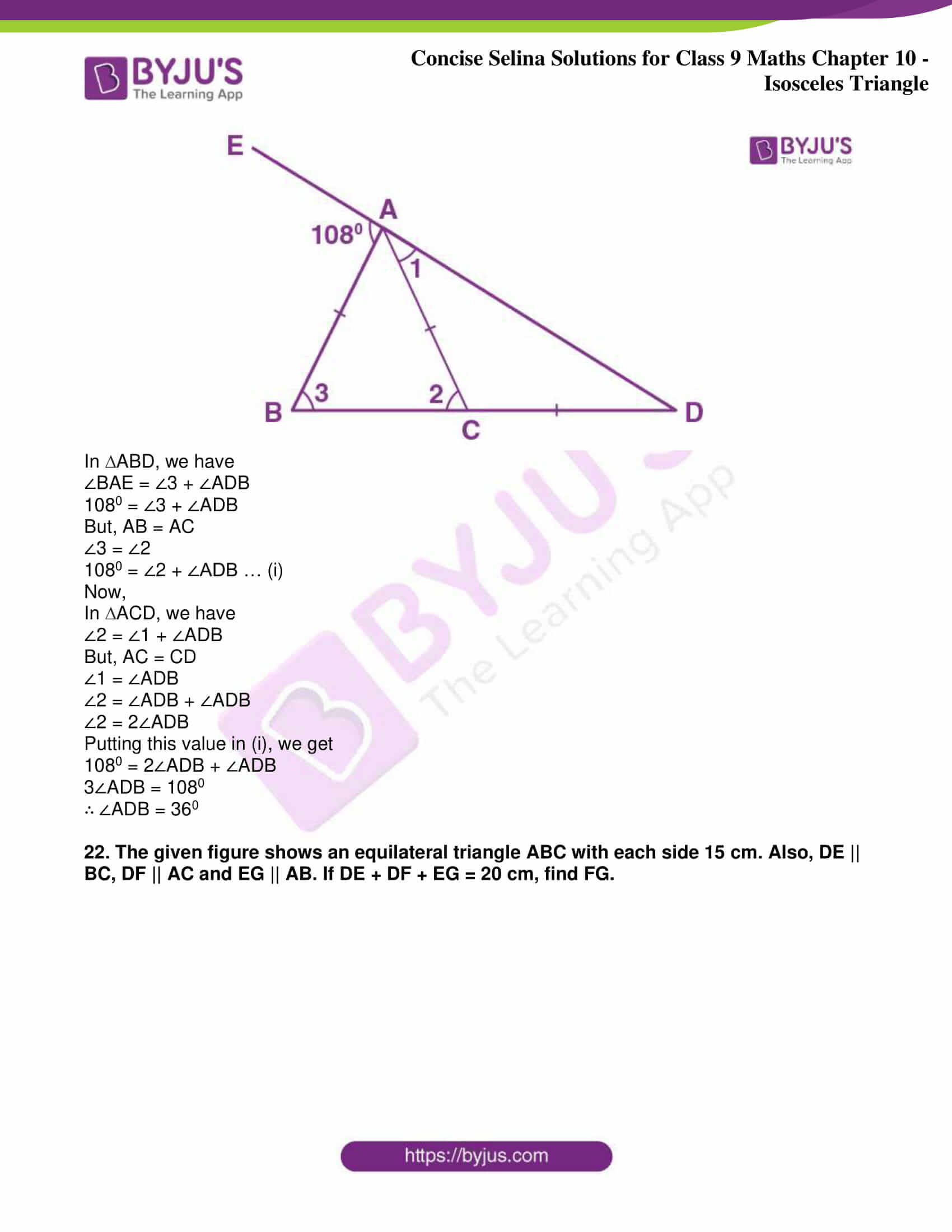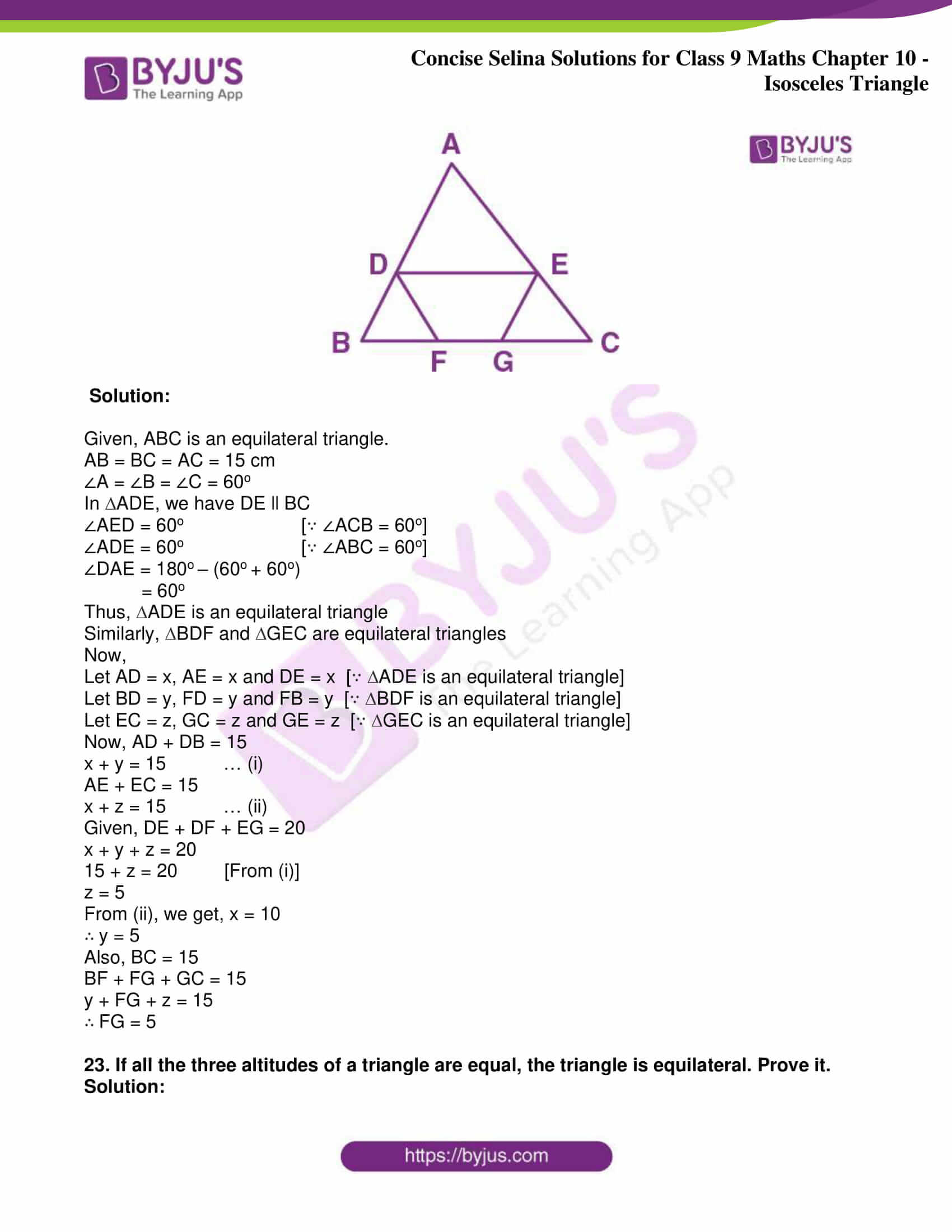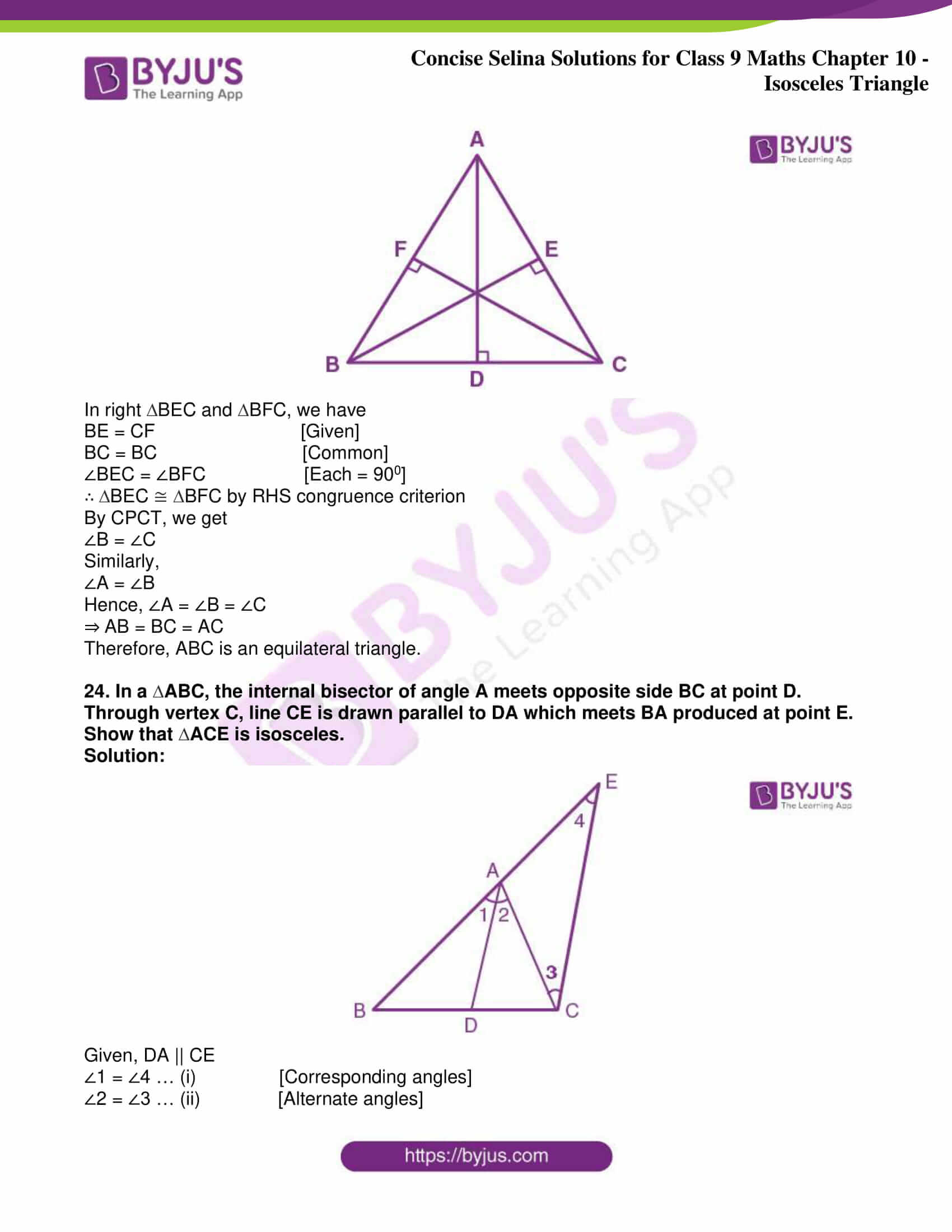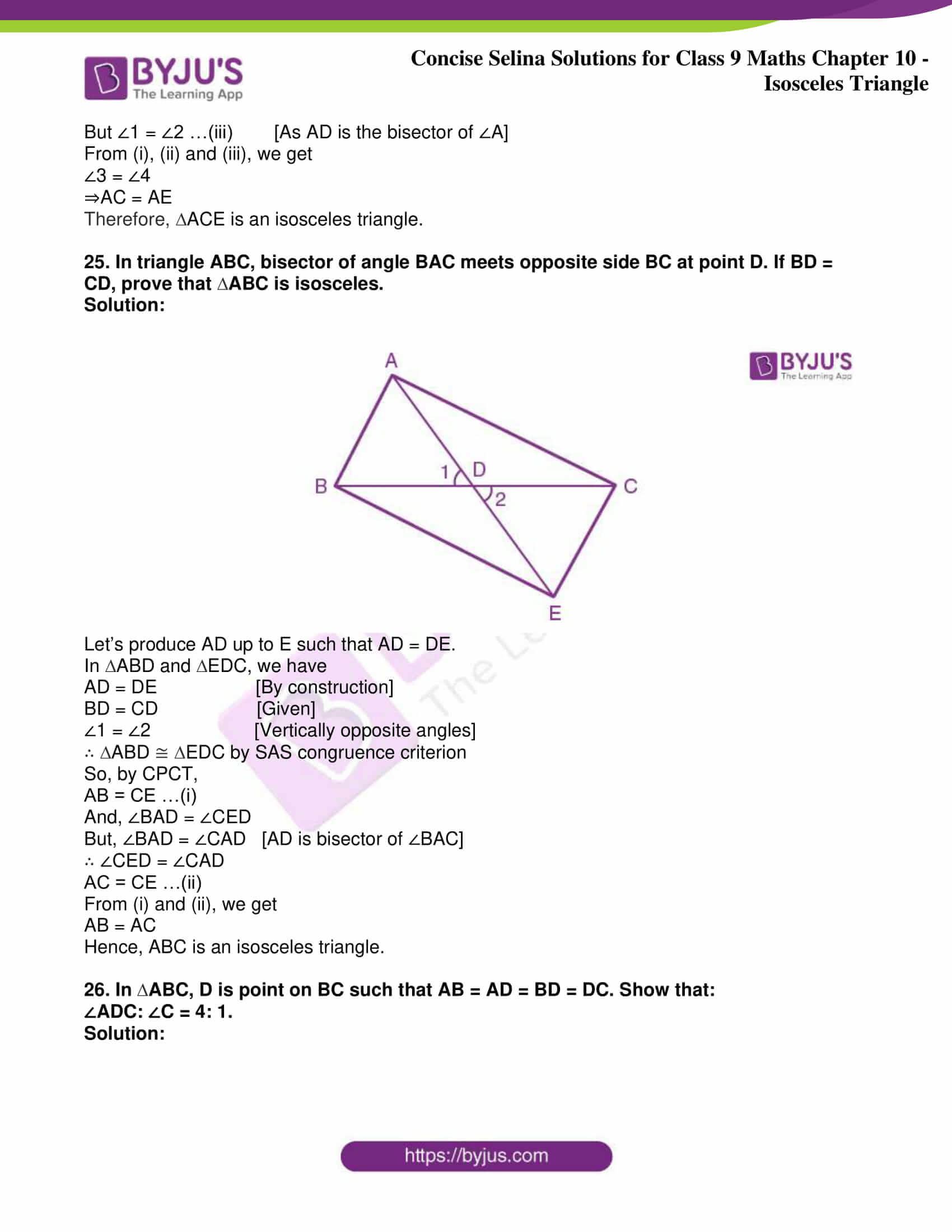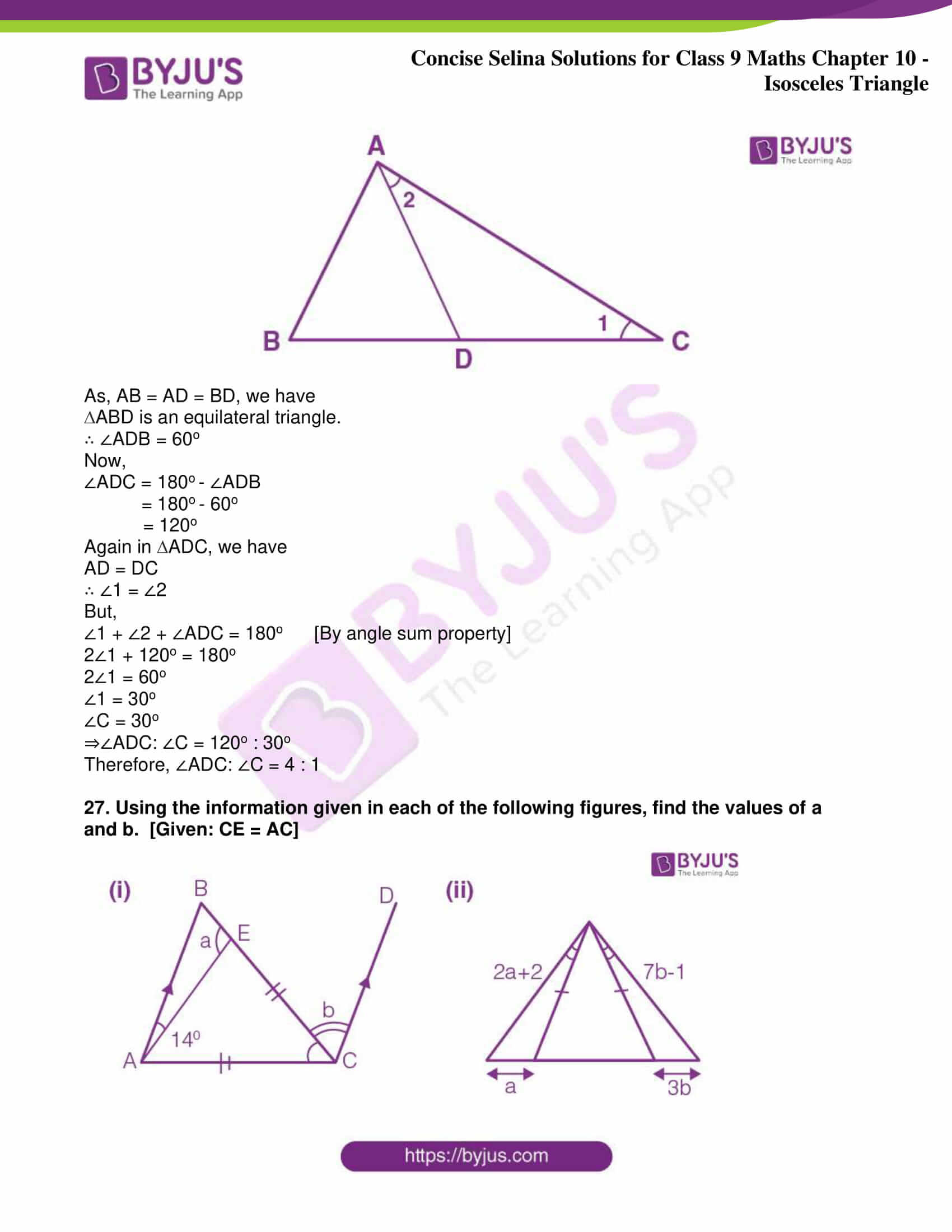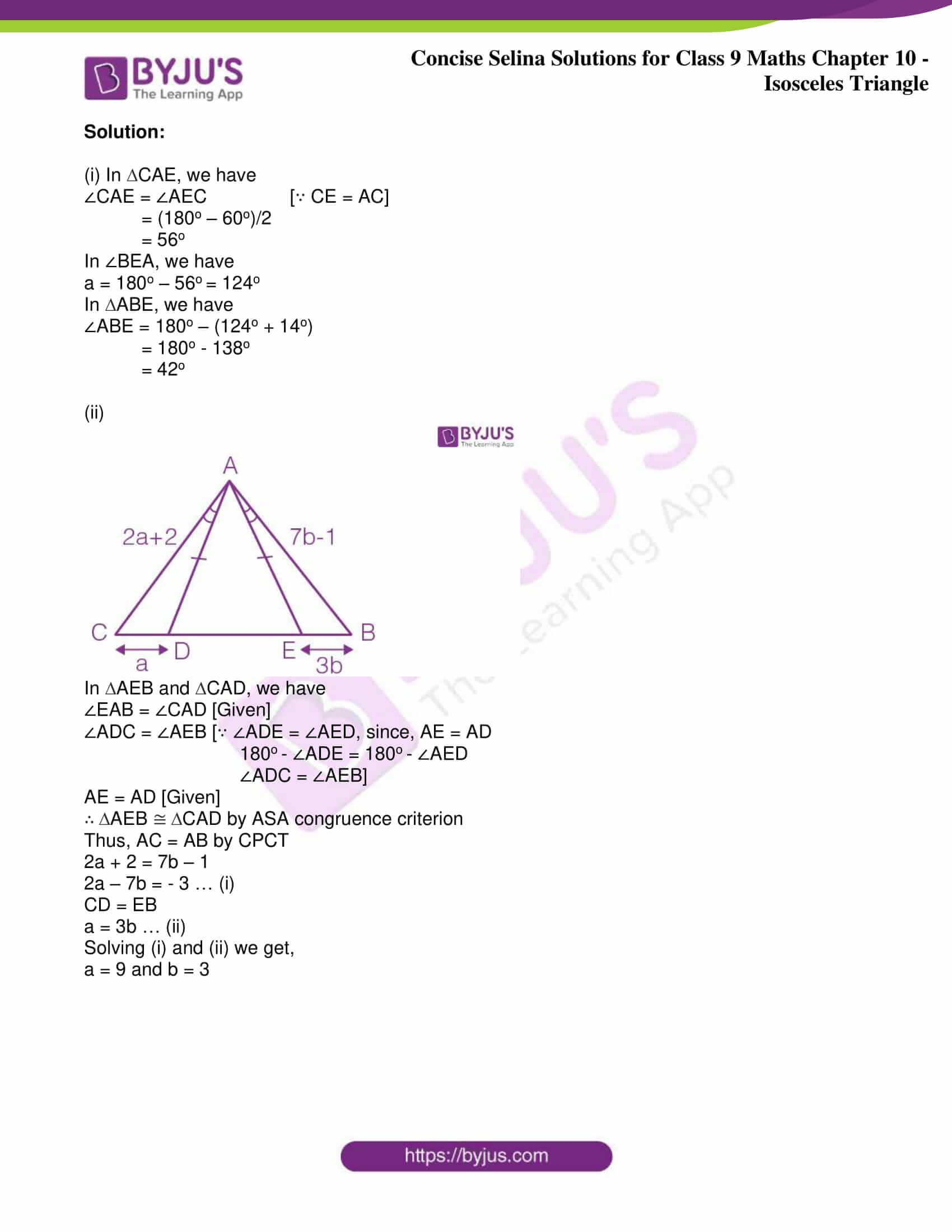Exercise 10(A)

1. In the figure alongside,AB = AC

∠A = 48o and

∠ACD = 18o.

Show that BC = CD.

Solution:

In ∆ABC, we have

BAC + ACB + ABC = 1800

480 + ACB + ABC = 1800

But, ACB = ABC  [Given, AB = AC]

2ABC = 1800 – 480

2ABC = 1320

ABC = 660 = ACB ……(i)

ACB = 660

ACD + DCB = 660

180 + DCB = 660

DCB = 480 ………(ii)

Now, In ∆DCB,

DBC = 660  [From (i), Since ABC = DBC]

DCB = 48[From (ii)]

BDC = 1800 – 480 – 660

BDC = 660

Since BDC = DBC

Therefore, BC = CD

Equal angles have equal sides opposite to them.

2. Calculate:

(ii) ∠ABC

(iii) ∠BAC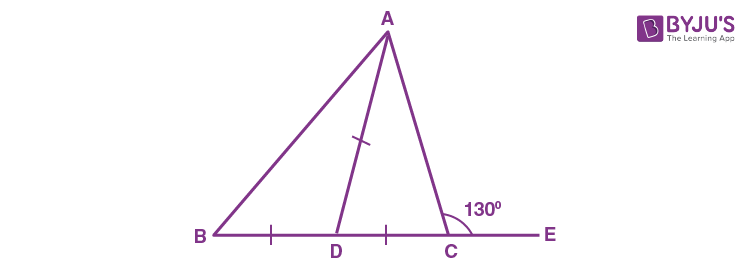Solution:

Given: ∠ACE = 1300; AD = BD = CD

Proof:

(i) ∠ACD + ∠ACE = 180o [DCE is a straight line]

∠ACD = 180o – 130o

∠ACD = 50o

Now,

∠ACD = ∠DAC = 50o … (i) [Since angles opposite to equal sides are equal]

∠ACD = ∠DAC = 50o

∠ACD + ∠DAC + ∠ADC = 180o

50o + 50o + ∠ADC = 180o

(ii) ∠ADC = ∠ABD + ∠DAB [Exterior angle is equal to sum of opposite interior angles]

∴ ∠DAB=∠ABD

80o = ∠ABD + ∠ABD

2∠BD = 80O

∠ABD = 40O = ∠DAB … (ii)

(iii) We have,

∠BAC = ∠DAB + ∠DAC

Substituting the values from (i) and (ii),

∠BAC = 40O + 50O

Hence, ∠BAC = 90O

3. In the following figure, AB = AC; BC = CD and DE is parallel to BC. Calculate:

(i) ∠CDE

(ii) ∠DCESolution:

Given, ∠FAB = 128O

∠BAC + ∠FAB = 180O [As FAC is a straight line]

∠BAC = 180O – 128O

∠BAC = 52O

In ∆ABC, we have

∠A = 52O

∠B = ∠C [Given AB = AC and angles opposite to equal sides are equal]

Now, by angle sum property

∠A + ∠B + ∠C =180O

∠A + ∠B + ∠B = 180O

52O+ 2∠B = 180O

2∠B = 128O

∠B = 64O = ∠C… (i)

∠B = ∠ADE [Given DE ll BC]

(i) Now, ∠ADE + ∠CDE + ∠B = 180O [As ADB is a straight line]

64O + ∠CDE + 64O= 180O

∠CDE = 180O+ 128O

∠CDE = 52O

(ii) Given DE ll BC and DC is the transversal

∠CDE = ∠DCB = 52o… (ii)

Also, ∠ECB = 64o… [From (i)]

But,

∠ECB = ∠DCE +∠DCB

64o = ∠DCE + 52o

∠DCE = 64o – 52o

∠DCE = 12o

4. Calculate x: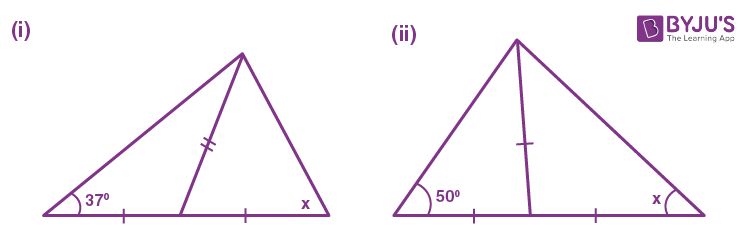Solution:

(i) Let the triangle be ABC and the altitude be AD.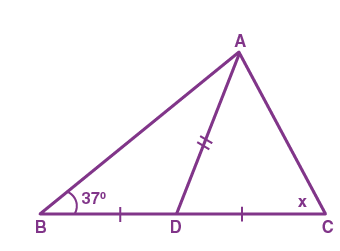In ∆ABD, we have

∠DBA = ∠DAB = 37o [Given BD = AD and angles opposite to equal sides are equal]

Now,

∠CDA = ∠DBA + ∠DAB [Exterior angle is equal to the sum of opposite interior angles]

∠CDA = 37o + 37o

∴ ∠CDA = 74o

∠CDA = ∠CAD = 74o [Given CD = AC and angels opposite to equal sides are equal]

Now, by angle sum property

∠CAD + ∠CDA + ∠ACD = 180o

74o + 74o + x = 180o

x = 180o – 148o

x = 32o

(ii) Let triangle be ABC and altitude be AD.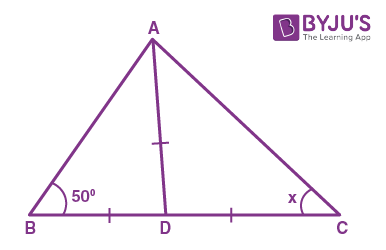In ∆ABD, we have

∠DBA = ∠DAB = 50o [Given BD = AD and angles opposite to equal sides are equal]

Now,

∠CDA = ∠DBA + ∠DAB [Exterior angle is equal to the sum of opposite interior angles]

∠CDA = 50o + 50o

∴ ∠CDA = 100o

∠DAC = ∠DCA = x [Given AD = DC and angels opposite to equal sides are equal]

So, by angle sum property

∠DAC + ∠DCA + ∠ADC = 180o

x + x + 100o = 180o

2x = 80o

x = 40o

5. In the figure, given below, AB = AC. Prove that: ∠BOC = ∠ACD.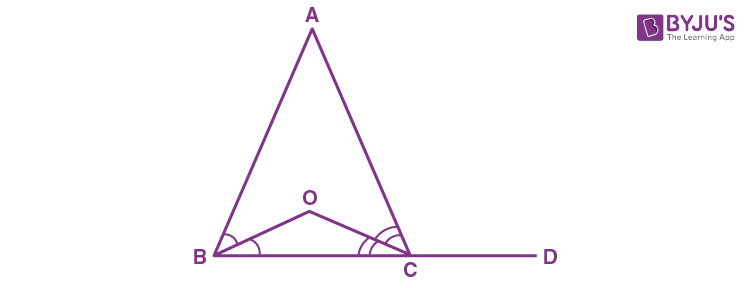Solution: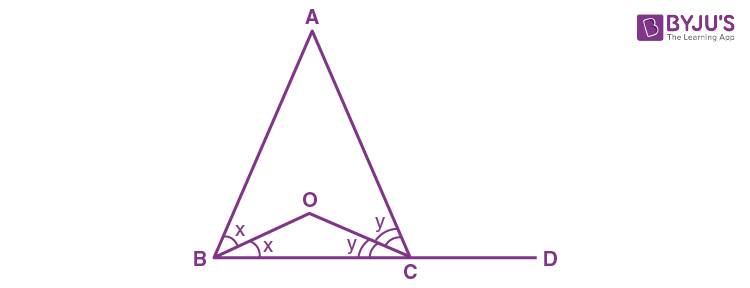Let’s assume ∠ABO = ∠OBC = x and ∠ACO = ∠OCB = y

In ABC, we have

∠BAC = 180o – 2x – 2y…(i)

As, ∠B = ∠C [Since, AB = AC]

½ ∠B = ½ ∠C

⇒ x = y

Now,

∠ACD = 2x + ∠BAC [Exterior angle is equal to sum of opposite interior angle]

= 2x + 180o – 2x – 2y [From (i)]

∠ACD = 180o – 2y… (ii)

In ∆OBC, we have

∠BOC = 180o – x – y

∠BOC = 180o – y – y [Since x = y]

∠BOC = 180o – 2y… (iii)

Thus, from (ii) and (iii) we get

∠BOC = ∠ACD

6. In the figure given below, LM = LN; ∠PLN = 110o. Calculate: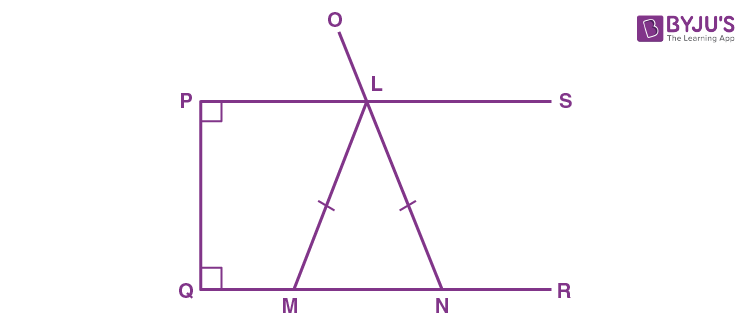(i) ∠LMN

(ii) ∠MLN

Solution:

Given, LM = LN and ∠PLN = 110o

(i) We know that the sum of the measure of all the angles of a quadrilateral is 360o.

∠QPL + ∠PLN +LNQ + ∠NQP = 360o

90o + 110o + ∠LNQ + 90o = 360o

∠LNQ = 360o – 290o

∠LNQ = 70o

∠LNM = 70o… (i)

In ∆LMN, we have

LM = LN [Given]

⇒ ∠LNM = ∠LMN [ Angles opposite to equal sides are equal]

∠LMN = 70o…(ii) [From (i)]

(ii) In ∆LMN, we have

∠LMN + ∠LNM + ∠MLN = 180o

But, ∠LNM = ∠LMN = 70o [From (i) and (ii)]

⇒ 70o + 70o + ∠MLN = 180o

∠MLN = 180o – 140o

∴ ∠MLN = 40o

7. An isosceles triangle ABC has AC = BC. CD bisects AB at D and ∠CAB = 55o.

Find: (i) ∠DCB (ii) ∠CBD.

Solution: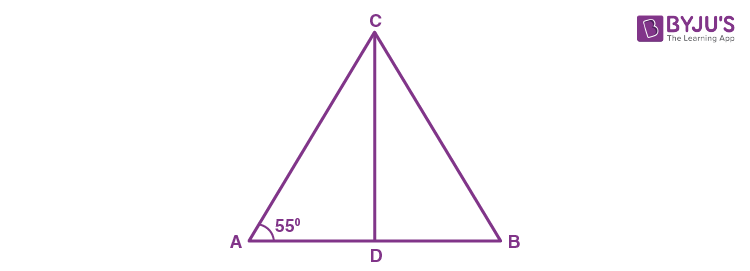In ∆ABC, we have

AC = BC [Given]

So, ∠CAB = ∠CBD [Angles opposite to equal sides are equal]

⇒ ∠CBD = 55o

In ∆ABC, we have

∠CBA + ∠CAB + ∠ACB = 180o

But, ∠CAB = ∠CBA = 55o

55o + 55o + ∠ACB = 180o

∠ACB = 180o – 110o

∠ACB = 70o

Now,

In ∆ACD and ∆BCD, we have

AC = BC [Given]

CD = CD [Common]

AD = BD [Given that CD bisects AB]

∴ ∆ACD ≅ ∆BCD

So, By CPCT

∠DCA = ∠DCB

∠DCB = ∠ACB/2 = 70o/2

Thus, ∠DCB = 35o

8. Find x: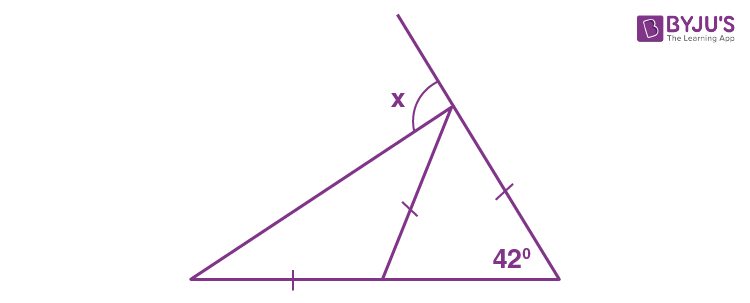Solution:

Let’s put markings to the figure as following: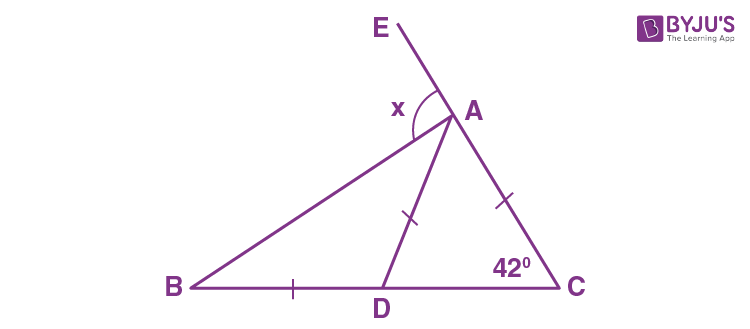In ∆ABC, we have

∴ ∠ADC = ∠ACD [Angles opposite to equal sides are equal]

Now,

∠ADC = ∠DAB + DBA [Exterior angle is equal to the sum of opposite interior angles]

But,

∠DAB = ∠DBA [Given: BD = DA]

2∠DBA = 42o

∠DBA = 21o

To find x:

x = ∠CBA + ∠BCA [Exterior angle is equal to the sum of opposite interior angles]

We know that,

∠CBA = 21o

∠BCA = 42o

⇒ x = 21o + 42o

∴ x = 63o

9. In the triangle ABC, BD bisects angle B and is perpendicular to AC. If the lengths of the sides of the triangle are expressed in terms of x and y as shown, find the values of x and y.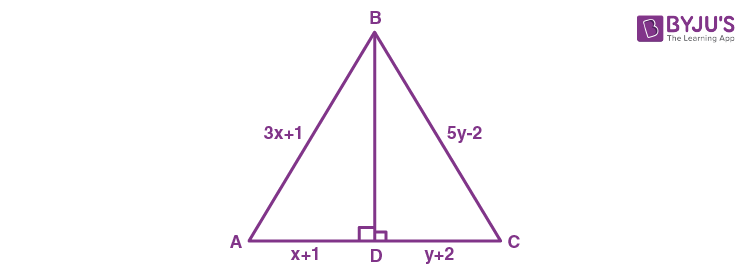Solution:

In ∆ABC and ∆DBC, we have

BD = BD [Common]

∠BDA = ∠BDC [Each equal to 90o]

∠ABD = ∠DBC [BD bisects ∠ABC]

∴ ∆ABD ≅ ∆DBC [ASA criterion]

Therefore, by CPCT

x + 1 = y + 2

x = y + 1… (i)

And, AB = BC

3x + 1 = 5y – 2

Substituting the value of x from (i), we get

3(y+1) + 1 = 5y – 2

3y + 3 + 1 = 5y – 2

3y + 4 = 5y – 2

2y = 6

y = 3

Putting y = 3 in (i), we get

x = 3 + 1

∴ x = 4

10. In the given figure; AE // BD, AC // ED and AB = AC. Find ∠a, ∠b and ∠c.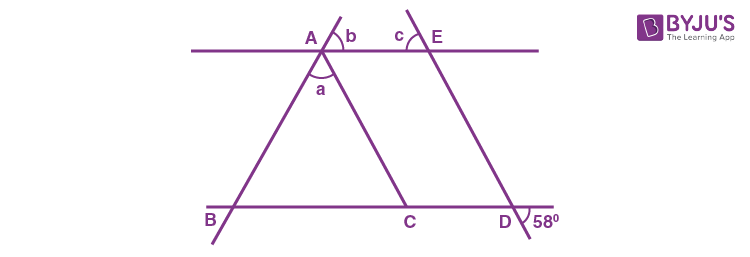Solution:

Let’s assume points P and Q as shown below: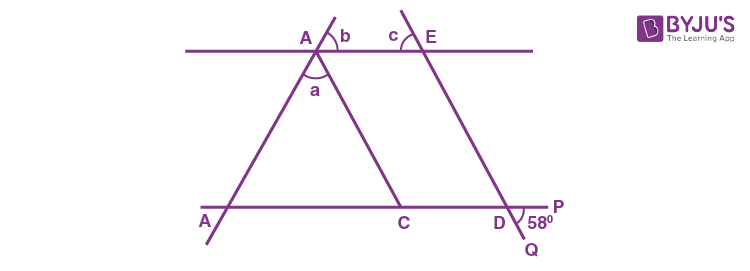Given, ∠PDQ = 58o

∠PDQ = ∠EDC = 58o [Vertically opposite angles]

∠EDC = ∠ACB = 58o [Corresponding angles ∵ AC ll ED]

In ∆ABC, we have

AB = AC [Given]

∴ ∠ACB = ∠ABC = 58o [Angles opposite to equal sides are equal]

Now,

∠ACB + ∠ABC + ∠BAC = 180o

58o + 58o + a = 180o

∠a = 180o – 116o

∠a = 64o

Since, AE ll BD and AC is the transversal

∠ABC = ∠b [Corrosponding angles]

∴ ∠b = 58o

Also, since AE ll BD and ED is the transversal

∠EDC = ∠c [Corrosponding angles]

∴ ∠c = 58o

11. In the following figure; AC = CD, AD = BD and ∠C = 58o.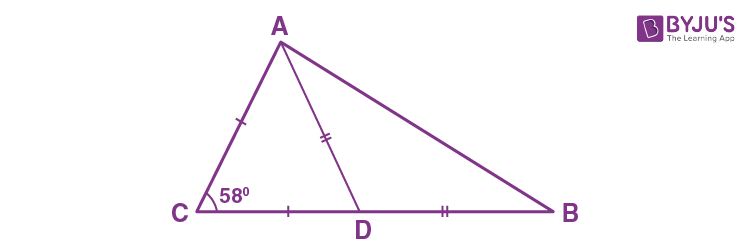Find ∠CAB.

Solution:

In ∆ACD, we have

AC = CD [Given]

∴ ∠CAD = ∠CDA [Angles opposite to equal sides are equal]

And,

∠ACD = 58o [Given]

By angle sum property, we have

∠ACD + ∠CDA + ∠CAD = 180o

∠CAD = ∠CDA = 61o… (i)

Now,

∠CDA = ∠DAB + ∠DBA [Exterior angles is equal to sum of opposite interior angles]

But,

∠DAB = ∠DBA [Given, AD = DB]

So, ∠DAB + ∠DAB = ∠CDA

2∠DAB = 61o

∠DAB = 30.5o… (ii)

In ∆ABC, we have

∠CAB = 61o + 30.5o [From (i) and (ii)]

∴ ∠CAB = 91.5 o

12. In the figure of Q.11 is given above, if AC = AD = CD = BD; find angle ABC.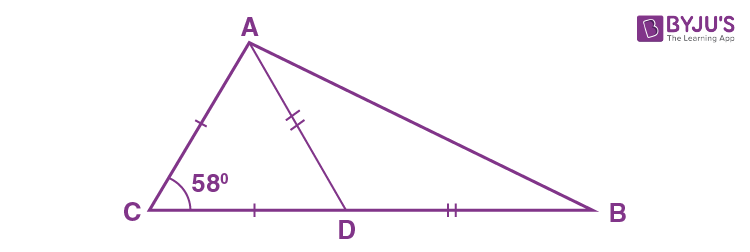Solution:

In ∆ACD, we have

AC = AD = CD [Given]

Hence, ACD is an equilateral triangle

∴ ∠ACD = ∠CDA = ∠CAD = 60o

Now,

∠CDA = ∠DAB + ∠ABD [Exterior angle is equal to sum of opposite interior angles]

But,

∠DAB = ∠ABD [Given, AD = DB]

So, ∠ABD + ∠ABD = ∠CDA

2∠ABD = 60o

∴ ∠ABD = ∠ABC = 30o

13. In ∆ABC; AB = AC and ∠A: ∠B = 8: 5; find ∠A.

Solution:Let, ∠A = 8x and ∠B = 5x

Given, In ∆ABC

AB = AC

So, ∠B = ∠C = 5x [Angles opp. to equal sides are equal]

Now, by angle sum property

∠A + ∠B +C = 180o

8x + 5x + 5x = 180o

18x = 180o

x = 10o

Thus, as ∠A = 8x

∠A = 8 × 10o

∴ ∠A = 80o

14. In triangle ABC; ∠A = 60o, ∠C = 40o, and bisector of angle ABC meets side AC at point P. Show that BP = CP.

Solution: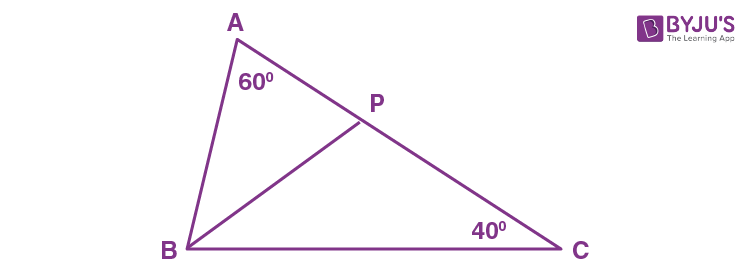In ∆ABC, we have

∠A = 60o

∠C = 40o

∴ ∠B = 180o – 60o – 40o [By angle sum property]

∠B = 80o

Now, as BP is the bisector of ∠ABC

∴ ∠PBC = ∠ABC/2

∠PBC = 40o

In ∆PBC, we have

∠PBC = ∠PCB = 40o

∴ BP = CP [Sides opposite to equal angles are equal]

15. In triangle ABC; angle ABC = 90o and P is a point on AC such that ∠PBC = ∠PCB. Show that: PA = PB.

Solution: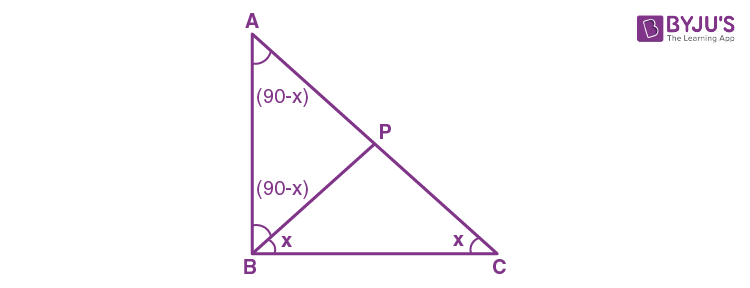Let’s assume ∠PBC = ∠PCB = x

In the right-angled triangle ABC,

∠ABC = 90o

∠ACB = x

∠BAC = 180o – (90o + x) [By angle sum property]

∠BAC = (90o – x) …(i)

And

∠ABP = ∠ABC – ∠PBC

∠ABP = 90o – x …(ii)

Thus, in the ∆ABP from (i) and (ii), we have

∠BAP = ∠ABP

Therefore, PA = PB  [sides opp. to equal angles are equal]

16. ABC is an equilateral triangle. Its side BC is produced upto point E such that C is mid-point of BE. Calculate the measure of angles ACE and AEC.

Solution: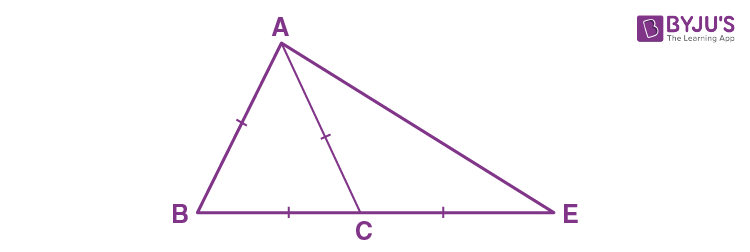Given, ∆ABC is an equilateral triangle

So, AB = BC = AC

∠ABC = ∠CAB = ∠ACB = 60o

Now, as sum of two non-adjacent interior angles of a triangle is equal to the exterior angle

∠CAB + ∠CBA = ∠ACE

60o + 60o = ∠ACE

∠ACE = 120o

Now,

∆ACE is an isosceles triangle with AC = CF

∠EAC = ∠AEC

By angle sum property, we have

∠EAC + ∠AEC + ∠ACE = 180o

2∠AEC + 120o = 180o

2∠AEC = 180o – 120o

∠AEC = 30o

17. In triangle ABC, D is a point in AB such that AC = CD = DB. If ∠B = 28°, find the angle ACD.

Solution: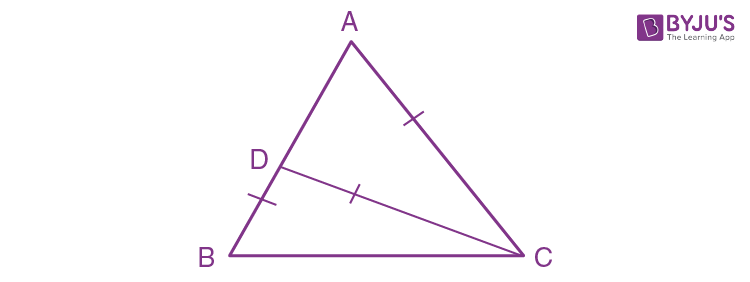From given, we get

∆DBC is an isosceles triangle

⇒ CD = DB

∠DBC = ∠DCB [If two sides of a triangle are equal, then angles opposites to them are equal]

And, ∠B = ∠DBC = ∠DCB = 28o

By angle sum property, we have

∠DCB + ∠DBC + ∠BCD = 180o

28o + 28o + ∠BCD = 180o

∠BCD = 180o – 56o

∠BCD = 124o

As sum of two non-adjacent interior angles of a triangle is equal to the exterior angle, we have

∠DBC + ∠DCB = ∠DAC

28o + 28o = 56o

∠DAC = 56o

Now,

∆ACD is an isosceles triangle with AC = DC

⇒ ∠ADC = ∠DAC = 56o

∠ADC + ∠DAC +∠DCA = 180o [By angle sum property]

56o + 56o + ∠DCA = 180o

∠DCA = 180o – 112o

∠DCA = 64o

Thus, ∠ACD = 64o

18. In the given figure, AD = AB = AC, BD is parallel to CA and ∠ACB = 65°. Find ∠DAC.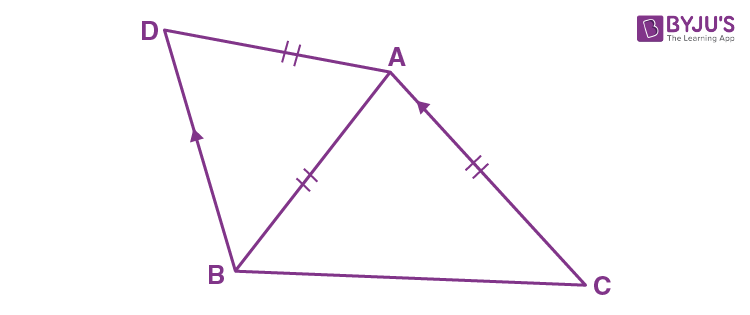Solution:

From figure, it’s seen that

∆ABC is an isosceles triangle with AB = AC

⇒ ∠ACB = ∠ABC

As ∠ACB = 65o [Given]

∴ ∠ABC = 65o

By angle sum property, we have

∠ACB + ∠CAB + ∠ABC = 180o

65o + 65o + ∠CAB = 180o

∠CAB = 180o – 130o

∠CAB = 50o

As BD is parallel to CA, we have

∠CAB = ∠DBA as they are alternate angles

⇒ ∠CAB = ∠DBA = 50o

Again, from figure, it’s seen that

⇒ ∠ADB = ∠DBA = 50o

By angle sum property, we have

∠ADB + ∠DAB + ∠DBA = 180o

50o + ∠DAB + 50o = 180o

∠DAB = 180o – 100o

∠DAB = 80o

Now,

∠DAC = ∠CAB + ∠DAB

∠DAC = 50o – 80o

∠DAC = 130o

19. Prove that a triangle ABC is isosceles, if:

(i)  altitude AD bisects angles BAC, or

(ii)  bisector of angle BAC is perpendicular to base BC.

Solution:

(i)  In
ΔABC, if the altitude AD bisect ∠BAC.

Then, to prove: ΔABC is isosceles.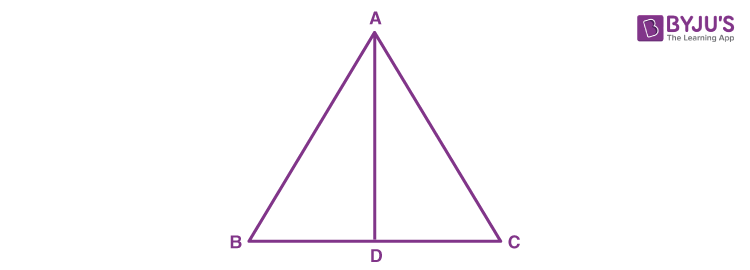In

So, by CPCT

AB = AC

Hence, ΔABC is an isosceles.

(ii)  In Δ ABC, the bisector of ∠BAC is perpendicular to the base BC.

Then, to prove: ΔABC is isosceles.Thus, by CPCT

AB = AC

Hence, ΔABC is an isosceles.

20. In the given figure; AB = BC and AD = EC.

Prove that: BD = BE.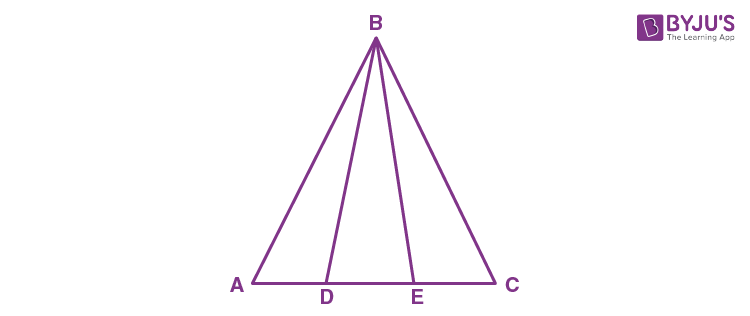Solution:

In ΔABC, we have

AB = BC  (Given)

So, ∠BCA = ∠BAC  (Angles opposite to equal sides are equal)

⇒ ∠BCD = ∠BAE ….(i)

AD + DE = EC + DE (Adding DE on both sides)

⇒ AE = CD ….(ii)

Now, in ΔABE and ΔCBD, we have

AB = BC  (Given)

∠BAE = ∠BCD  [From (i)]

AE = CD  [From (ii)]

Therefore, ΔABE ≅ ΔCBD by SAS congruence criterion

So, by CPCT

BE = BD

Exercise 10(B)

1. If the equal sides of an isosceles triangle are produced, prove that the exterior angles so formed are obtuse and equal.

Solution:Construction: AB is produced to D and AC is produced to E so that exterior angles ∠DBC and ∠ECB are formed.

In ∆ABC, we have

AB = AC [Given]

∴ ∠C = ∠B …(i) [Angles opposite to equal sides are equal]

Since, ∠B and ∠C are acute they cannot be right angles or obtuse angles

Now,

∠ABC + ∠DBC = 1800 [ABD is a straight line]

∠DBC = 1800 – ∠ABC

∠DBC = 1800 – ∠B …(ii)

Similarly,

∠ACB + ECB = 1800 [ABD is a straight line]

∠ECB = 1800 – ∠ACB

∠ECB = 1800 – ∠C …(iii)

∠ECB = 1800 – ∠B …(iv) [from (i) and (iii)]

∠DBC = ∠ECB [from (ii) and (iv)]

Now,

∠DBC = 1800 – ∠B

But, ∠B is an acute angle

⇒ ∠DBC = 1800 – (acute angle) = obtuse angle

Similarly,

∠ECB = 1800 – ∠C

But, ∠C is an acute angle

⇒ ∠ECB = 1800 – (acute angle) = obtuse angle

Therefore, exterior angles formed are obtuse and equal.

2. In the given figure, AB = AC. Prove that: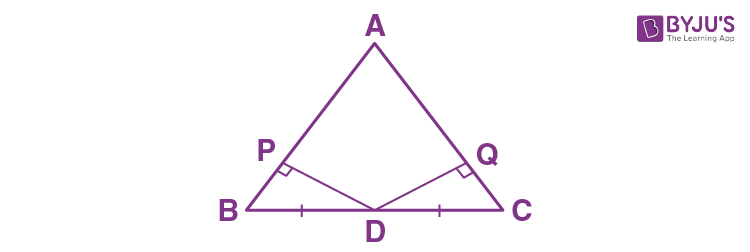(i) DP = DQ

(ii) AP = AQ

Solution: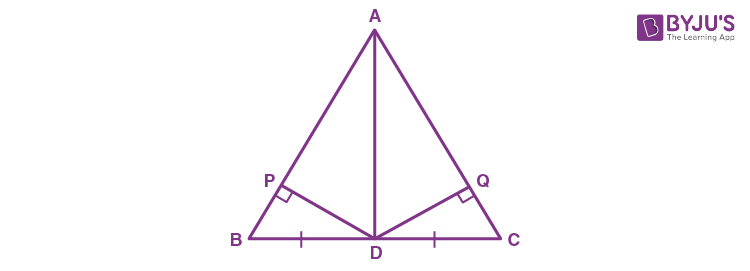In ∆ABC, we have

AB = AC [Given]

∴ ∠C = ∠B …(i) [ Angles opposite to equal sides are equal]

(i) In ∆BPD and ∆CQD, we have

∠BPD = ∠CQD [Each = 90o]

∠B = ∠C [Proved]

BD = DC [Given]

Thus, ∆BPD ≅ ∆CQD by AAS congruence criterion

∴ DP = DQ by CPCT

(ii) Since, ∆BPD ≅ ∆CQD

Therefore, BP = CQ [CPCT]

Now,

AB = AC [Given]

AB – BP = AC – CQ

AP = AQ

(iii) In ∆APD and ∆AQD, we have

DP = DQ [Proved]

AP = AQ [Proved]

Thus, ∆APD ≅ ∆AQD by SSS congruence criterion

3. In triangle ABC, AB = AC; BE AC and CF AB. Prove that: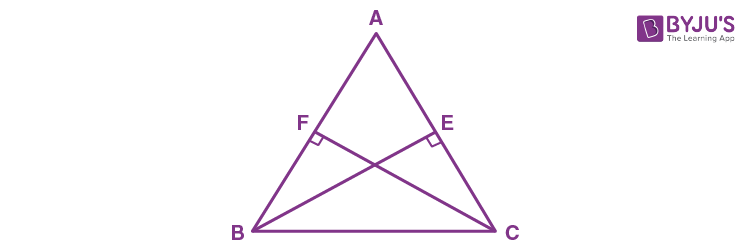(i) BE = CF

(ii) AF = AE

Solution:

(i) In ∆AEB and ∆AFC, we have

∠A = ∠A [Common]

∠AEB = ∠AFC = 90o [ Given : BE ⊥ AC and CE ⊥ AB]

AB = AC [Given]

Thus, ∆AEB ≅ ∆AFC by AAS congruence criterion

∴ BE = CF by CPCT

(ii) Since, ∆AEB ≅ ∆AFC

∠ABE = ∠AFC

∴ AF= AE by CPCT

4. In isosceles triangle ABC, AB = AC. The side BA is produced to D such that BA = AD. Prove that: ∠BCD = 90o

Solution: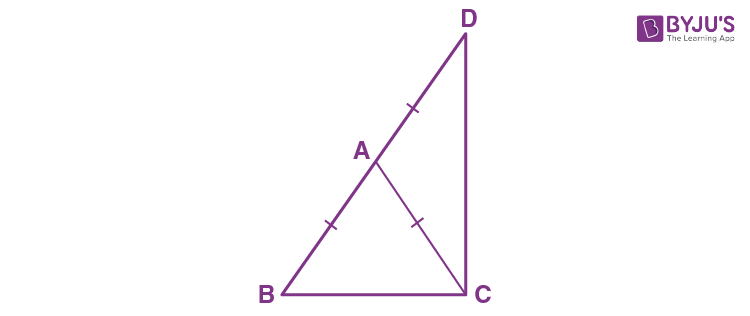Construction: Join CD.

In ∆ABC, we have

AB = AC [Given]

∴ ∠C = ∠B … (i) [ Angles opposite to equal sides are equal]

In ∆ACD, we have

∴ ∠ADC = ∠ACD … (ii)

Adding (i) and (ii), we get

∠B + ∠ADC = ∠C + ∠ACD

∠B + ∠ADC = ∠BCD … (iii)

In ∆BCD, we have

∠B + ∠ADC +∠BCD = 180o

∠BCD + ∠BCD = 180o [From (iii)]

2∠BCD = 180o

∴ ∠BCD = 90o

5. (i) In ∆ABC, AB = AC and ∠A= 36°. If the internal bisector of ∠C meets AB at point D, prove that AD = BC.

(ii) If the bisector of an angle of a triangle bisects the opposite side, prove that the triangle is isosceles.

Solution: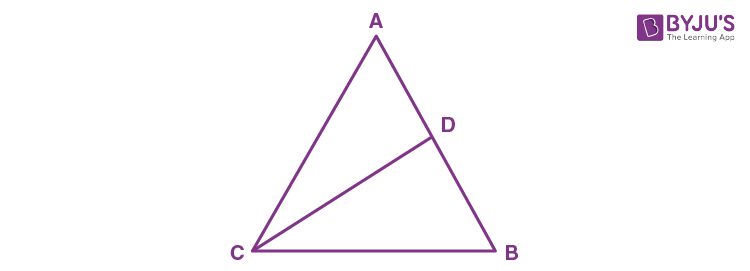Given, AB = AC and ∠A = 36o

So, ∆ABC is an isosceles triangle.

∠B = ∠C = (1800 – 36o)/2 = 72o

∠ACD = ∠BCD = 36o [∵ CD is the angle bisector of ∠C]

Now, ∆ADC is an isosceles triangle as ∠DAC = ∠DCA = 36o

In ∆DCB, by angle sum property we have

∠CDB = 180o – (∠DCB + ∠DBC)

= 180o – (36o + 72o)

= 180o – 108o

= 72o

Now, ∆DCB is an isosceles triangle as ∠CDB = ∠CBD = 72o

∴ DC = BC …(ii)

From (i) and (ii), we get

– Hence Proved.

6. Prove that the bisectors of the base angles of an isosceles triangle are equal.

Solution: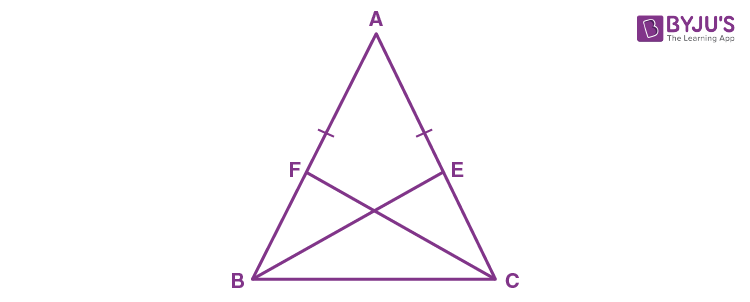In ∆ABC, we have

AB = AC [Given]

∴ ∠C = ∠B …(i) [Angles opposite to equal sides are equal]

½∠C = ½∠B

⇒ ∠BCF = ∠CBE …(ii)

Now, in ∆BCE and ∆CBF, we have

∠C = ∠B [From (i)]

∠BCF = ∠CBE [From (ii)]

BC = BC [Common]

∴ ∆BCE ≅ ∆CBF by AAS congruence criterion

Thus, BE = CF by CPCT

7. In the given figure, AB = AC and ∠DBC = ∠ECB = 90o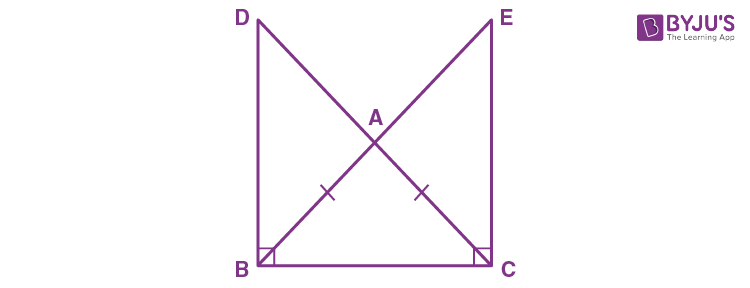Prove that:

(i) BD = CE

Solution:

In ∆ABC, we have

AB = AC [Given]

∴ ∠ACB = ∠ABC [Angles opposite to equal sides are equal]

⇒ ∠ABC = ∠ACB … (i)

∠DBC = ∠ECB = 90o [Given]

⇒ ∠DBC = ∠ECB …(ii)

Subtracting (i) from (ii), we get

∠DCB – ∠ABC = ∠ECB – ∠ACB

∠DBA = ∠ECA … (iii)

Now,

In ΔDBA and ΔECA, we have

∠DBA = ∠ECA [From (iii)]
∠DAB = ∠EAC [Vertically opposite angles]
AB = AC [Given]
∴ ΔDBA ≅ ΔECA by ASA congruence criterion

Thus, by CPCT

BD = CE

And, also

8. ABC and DBC are two isosceles triangles on the same side of BC. Prove that:

(i) DA (or AD) produced bisects BC at right angle.

(ii) ∠BDA = ∠CDA.

Solution: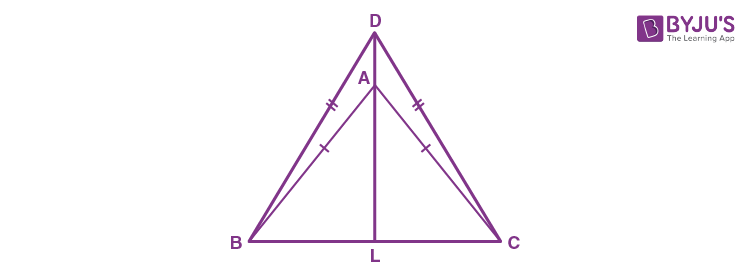DA is produced to meet BC in L

In ∆ABC, we have

AB = AC [Given]

∴ ∠ACB = ∠ABC … (i) [Angles opposite to equal sides are equal]

In ∆DBC, we have

DB = DC [Given]

∴ ∠DCB = ∠DBC … (ii) [Angles opposite to equal sides are equal]

Subtracting (i) from (ii), we get

∠DCB – ∠ACB = ∠DBC – ∠ABC

∠DCA = ∠DBA …(iii)

Now,

In ∆DBA and ∆DCA, we have

DB = DC [Given]

∠DBA = ∠DCA [From (iii)]

AB = AC [Given]

∴ ∆DBA ≅ ∆DCA by SAS congruence criterion

∠BDA = ∠CDA …(iv) [By CPCT]

In ∆DBA, we have

∠BAL = ∠DBA + ∠BDA …(v) [Exterior angle = sum of opposite interior angles]

From (iii), (iv) and (v), we get

∠BAL = ∠DCA + ∠CDA …(vi) [Exterior angle = sum of opposite interior angles]

In ∆DCA, we have

∠CAL = ∠DCA + ∠CDA …(vi)

From (vi) and (vii)

∠BAL = ∠CAL …(viii)

In ∆BAL and ∆CAL,

∠BAL = ∠CAL [From (viii)]

∠ABL = ∠ACL [From (i)

AB = AC [Given]

∴ ∆BAL ≅ ∆CAL by ASA congruence criterion

So, by CPCT

∠ALB = ∠ALC

And, BL = LC …(ix)

Now,

∠ALB + ∠ALC = 180o

∠ALB + ∠ALB = 180o [Using (ix)]

2∠ALB = 180o

∠ALB = 90o

∴ AL ⊥ BC

Or DL ⊥ BC and BL ⊥ LC

Therefore, DA produced bisects BC at right angle.

9. The bisectors of the equal angles B and C of an isosceles triangle ABC meet at O. Prove that AO bisects angle A.

Solution: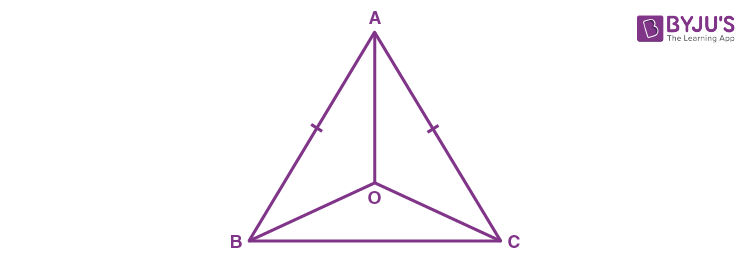In ∆ABC, we have AB = AC

∠B = ∠C [Angles opposite to equal sides are equal]

½∠B = ½∠C

∠OBC = ∠OCB …(i)

⇒ OB = OC …(ii) [Sides opposite to equal angles are equal]

Now,

In ∆ABO and ∆ACO, we have

AB = AC  [Given]

∠OBC = ∠OCB  [From (i)]

OB = OC  [From (ii)]

Thus, ∆ABO ≅ ∆ACO by SAS congruence criterion

So, by CPCT

∠BAO = ∠CAO

Therefore, AO bisects ∠BAC.

10. Prove that the medians corresponding to equal sides of an isosceles triangle are equal.

Solution:In ∆ABC, we have

AB = AC [Given]

∠C = ∠B … (i) [Angles opposite to equal sides are equal]

Now,

½ AB = ½ AC

BF = CE … (ii)

In ∆BCE and ∆CBF, we have

∠C = ∠B [From (i)]

BF = CE [From (ii)]

BC = BC [Common]

∴ ∆BCE ≅ ∆CBF by SAS congruence criterion

So, CPCT

BE = CF

11. Use the given figure to prove that, AB = AC.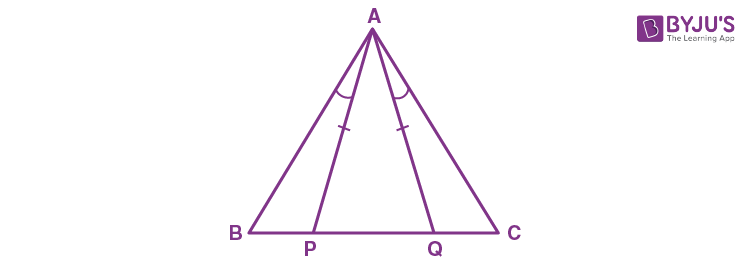Solution:

In ∆APQ, we have

AP = AQ [Given]

∴ ∠APQ = ∠AQP …(i) [Angles opposite to equal sides are equal]

In ∆ABP, we have

∠APQ = ∠BAP + ∠ABP …(ii) [Exterior angle is equal to sum of opposite interior angles]

In ∆AQC, we have

∠AQP = ∠CAQ + ∠ACQ …(iii) [Exterior angle is equal to sum of opposite interior angles]

From (i), (ii) and (iii), we get

∠BAP + ∠ABP = ∠CAQ + ∠ACQ

But, ∠BAP = ∠CAQ [Given]

∠CAQ + ABP = ∠CAQ + ∠ACQ

∠ABP = ∠CAQ + ∠ACQ – ∠CAQ

∠ABP = ∠ACQ

∠B = ∠C

So, in ∆ABC, we have

∠B = ∠C

⇒ AB = AC [Sides opposite to equal angles are equal]

12. In the given figure; AE bisects exterior angle CAD and AE is parallel to BC.

Prove that: AB = AC.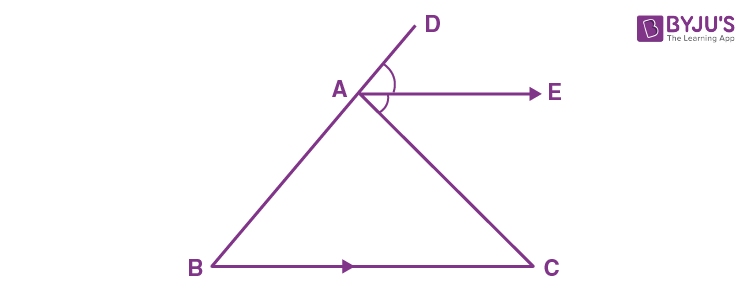Solution:

Since, AE || BC and DAB is the transversal

∴ ∠DAE = ∠ABC = ∠B [Corresponding angles]

Since, AE || BC and AC is the transversal

∠CAE = ∠ACB = ∠C [Alternate angles]

∴ ∠DAE = ∠CAE

∠B = ∠C

⇒ AB = AC [Sides opposite to equal angles are equal]

13. In an equilateral triangle ABC; points P, Q and R are taken on the sides AB, BC and CA respectively such that AP = BQ = CR. Prove that triangle PQR is equilateral.

Solution: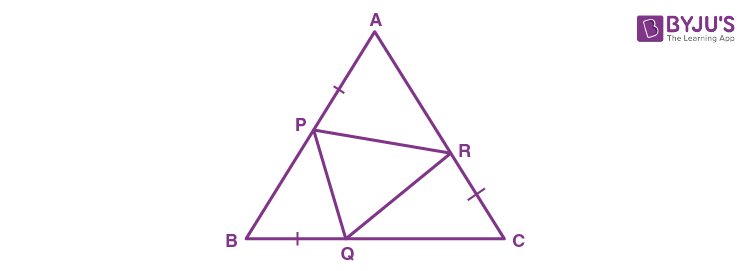Given, AB = BC = CA (Since, ABC is an equilateral triangle) …(i)

and AP = BQ = CR …(ii)

Subtracting (ii) from (i), we get

AB – AP = BC – BQ = CA – CR

BP = CQ = AR …(iii)

∴ ∠A = ∠B = ∠C …(iv) [Angles opposite to equal sides are equal]

In ∆BPQ and ∆CQR, we have

BP = CQ [From (iii)]

∠B = ∠C [From (iv)]

BQ = CR [Given]

∴ ∆BPQ ≅ ∆CQR by SAS congruence criterion

So, PQ = QR [by CPCT] … (v)

In ∆CQR and ∆APR, we have

CQ = AR [From (iii)]

∠C = ∠A [From (iv)]

CR = AP [Given]

∴ ∆CQR ≅ ∆APR by SAS congruence criterion

So, QR = PR [By CPCT] … (vi)

From (v) and (vi), we get

PQ = QR = PR

Therefore, PQR is an equilateral triangle.

14. In triangle ABC, altitudes BE and CF are equal. Prove that the triangle is isosceles.

Solution: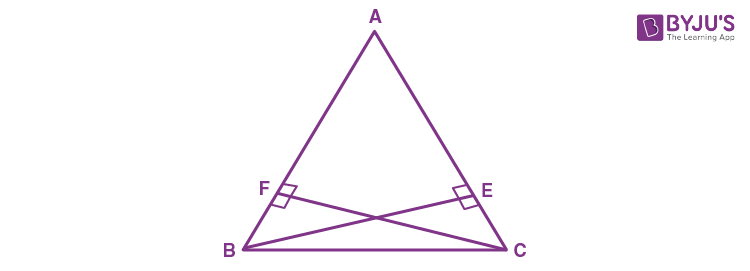In ∆ABE and ∆ACF, we have

∠A = ∠A [Common]

∠AEB = ∠AFC = 900 [Given: BE ⊥ AC and CF ⊥ AB]

BE = CF [Given]

∴ ∆ABE ≅ ∆ACF by AAS congruence criterion

So, by CPCT

AB = AC

Therefore, ABC is an isosceles triangle.

15. Through any point in the bisector of angle, a straight line is drawn parallel to either arm of the angle. Prove that the triangle so formed is isosceles.

Solution: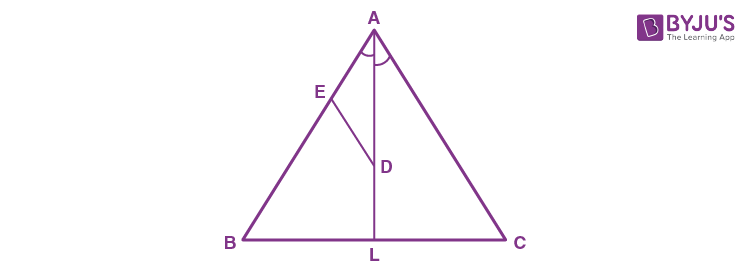Let’s consider ∆ABC, AL is bisector of ∠A.

Let D is any point on AL.

From D, a straight-line DE is drawn parallel to AC.

DE || AC  [Given]

So, ∠ADE = ∠DAC …(i)  [Alternate angles]

∠DAC = ∠DAE … (ii)  [AL is bisector of ∠A]

From (i) and (ii), we get

∴ AE = ED  [Sides opposite to equal angles are equal]

Therefore, AED is an isosceles triangle.

16. In triangle ABC; AB = AC. P, Q and R are mid-points of sides AB, AC and BC respectively. Prove that:

(i) PR = QR (ii) BQ = CP

Solution:

(i)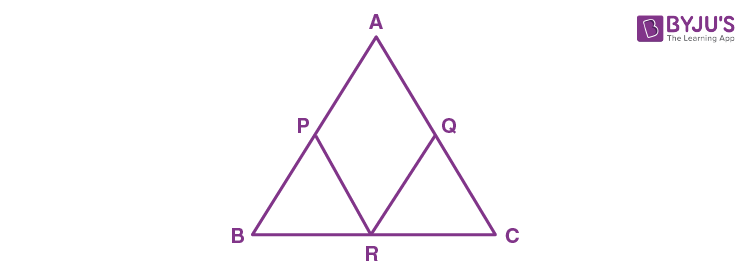In ∆ABC, we have

AB = AC

½ AB = ½ AC

AP = AQ … (i) [ Since P and Q are mid – points]

In ∆BCA, we have

PR = ½ AC [PR is line joining the mid – points of AB and BC]

PR = AQ … (ii)

In ∆CAB, we have

QR = ½ AB [QR is line joining the mid – points of AC and BC]

QR = AP …(iii)

From (i), (ii) and (iii), we get

PR = QR

(ii)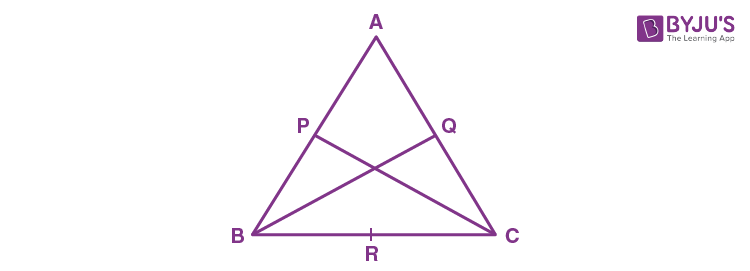Given, AB = AC

⇒ ∠B = ∠C

Also,

½ AB = ½ AC

BP = CQ [P and Q are mid – points of AB and AC]

Now, in ∆BPC and ∆CQB, we have

BP = CQ

∠B = ∠C

BC = BC (Common)

Therefore, ΔBPC ≅ CQB by SAS congruence criterion

∴ BP = CP by CPCT

17. From the following figure, prove that:

(i) ∠ACD = ∠CBE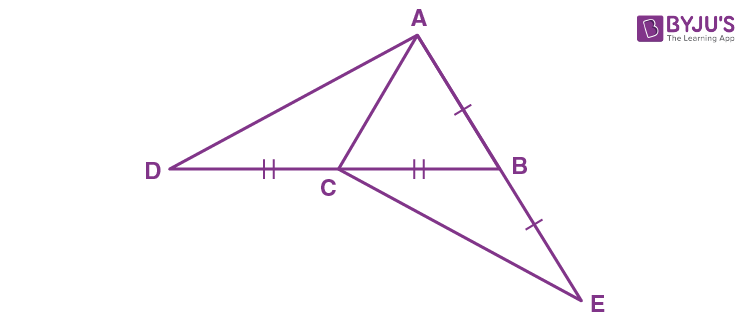Solution:

(i) In ∆ACB, we have

AC = AC [Given]

∴ ∠ABC = ∠ACB …(i) [Angles opposite to equal sides are equal]

∠ACD + ∠ACB = 1800 … (ii) [Since, DCB is a straight line]

∠ABC + ∠CBE = 1800 …(iii) [Since, ABE is a straight line]

Equating (ii) and (iii), we get

∠ACD + ∠ACB = ∠ABC + ∠CBE

∠ACD + ∠ACB = ∠ACB + ∠CBE [From (i)]

⇒ ∠ACD = ∠CBE

(ii) In ∆ACD and ∆CBE, we have

DC = CB [Given]

AC = BE [Given]

∠ACD = ∠CBE [Proved above]

∴ ∆ACD ≅ ∆CBE by SAS congruence criterion

Hence, by CPCT

18. Equal sides AB and AC of an isosceles triangle ABC are produced. The bisectors of the exterior angle so formed meet at D. Prove that AD bisects angle A.

Solution: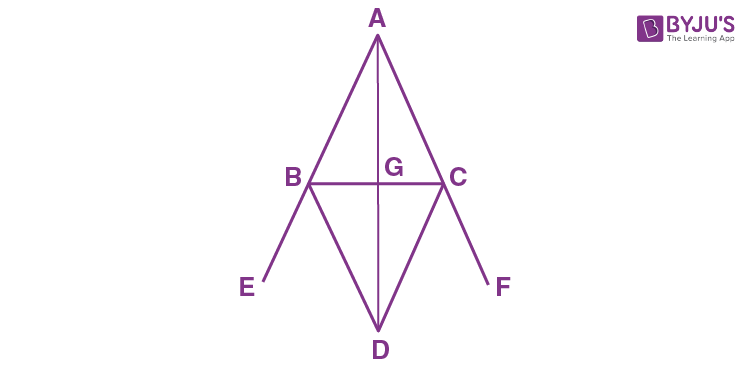AB is produced to E and AC is produced to F. BD is bisector of angle CBE and CD is bisector of angle BCF. BD and CD meet at D.

In ∆ABC, we have

AB = AC [Given]

∴ ∠C = ∠B [angles opposite to equal sides are equal]

∠CBE = 1800 – ∠B [ABE is a straight line]

∠CBD = (180o – ∠B)/ 2 [BD is bisector of ∠CBE]

∠CBD = 90o – ∠B/ 2 …(i)

Similarly,

∠BCF = 1800 – ∠C [ACF is a straight line]

∠BCD = (180o – ∠C)/ 2 [CD is bisector of ∠BCF]

∠BCD = 90o – ∠C/2 …(ii)

Now,

∠CBD = 90o – ∠C/2 [∵∠B = ∠C]

∠CBD = ∠BCD

In ∆BCD, we have

∠CBD = ∠BCD

∴ BD = CD

In ∆ABD and ∆ACD, we have

AB = AC [Given]

BD = CD [Proved]

∴ ∆ABD ≅ ∆ACD by SSS congruence criterion

19. ABC is a triangle. The bisector of the angle BCA meets AB in X. A point Y lies on CX such that AX = AY. Prove that ∠CAY = ∠ABC.

Solution: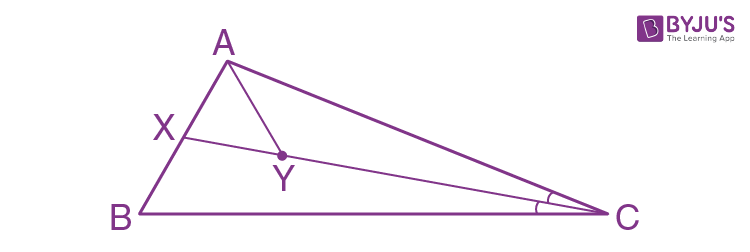In ∆ABC, we have

CX is the angle bisector of ∠C

So, ∠ACY = ∠BCX …(i)

In ∠AXY, we have

AX = AY  [Given]

∠AXY = ∠AYX …(ii)  [Angles opposite to equal sides are equal]

Now, ∠XYC = ∠AXB = 180°  [Straight line angle]

∠AYX + ∠AYC = ∠AXY + ∠BXY

∠AYC = ∠BXY… (iii)  [From (ii)]

In ∆AYC and ∆BXC, we have

∠AYC + ∠ACY + ∠CAY = ∠BXC + ∠BCX + ∠XBC = 180°

∠CAY = ∠XBC  [From (i) and (iii)]

Thus, ∠CAY = ∠ABC

20. In the following figure; IA and IB are bisectors of angles CAB and CBA respectively. CP is parallel to IA and CQ is parallel to IB.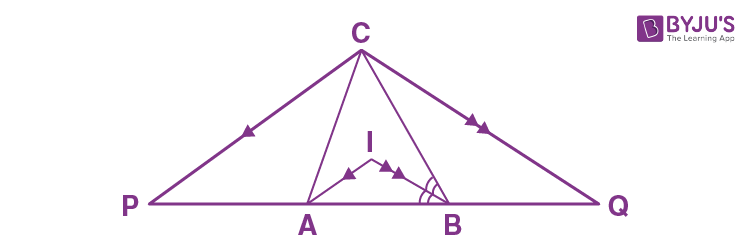Prove that:

PQ = The perimeter of the ABC.

Solution:

Since IA || CP and CA is a transversal

We have, ∠CAI = ∠PCA  [Alternate angles]

Also, IA || CP and AP is a transversal

We have, ∠IAB = ∠APC  [Corresponding angles]

But ∴ ∠CAI = ∠IAB  [Given]

∴ ∠PCA = ∠APC

AC = AP

Similarly, BC = BQ

Now,

PQ = AP + AB + BQ

= AC + AB + BC

= Perimeter of ∆ABC

21. Sides AB and AC of a triangle ABC are equal. BC is produced through C upto a point D such that AC = CD. D and A are joined and produced upto point E. If angle BAE = 108o; find angle ADB.

Solution: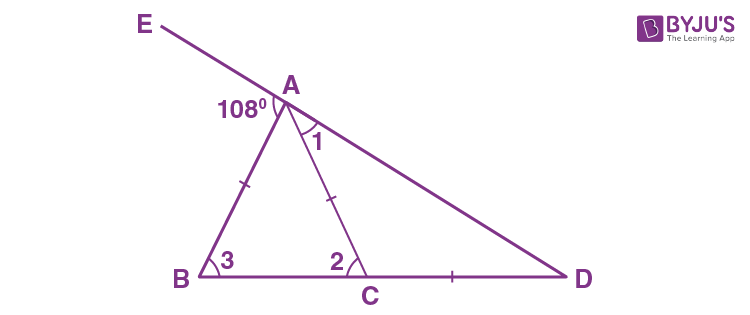In ∆ABD, we have

But, AB = AC

∠3 = ∠2

1080 = ∠2 + ∠ADB … (i)

Now,

In ∆ACD, we have

But, AC = CD

Putting this value in (i), we get

22. The given figure shows an equilateral triangle ABC with each side 15 cm. Also, DE || BC, DF || AC and EG || AB. If DE + DF + EG = 20 cm, find FG.Solution:

Given, ABC is an equilateral triangle.

AB = BC = AC = 15 cm

∠A = ∠B = ∠C = 60o

In ∆ADE, we have DE ll BC

∠AED = 60o [∵ ∠ACB = 60o]

∠ADE = 60o [∵ ∠ABC = 60o]

∠DAE = 180o – (60o + 60o)

= 60o

Thus, ∆ADE is an equilateral triangle

Similarly, ∆BDF and ∆GEC are equilateral triangles

Now,

Let AD = x, AE = x and DE = x [∵ ∆ADE is an equilateral triangle]

Let BD = y, FD = y and FB = y [∵ ∆BDF is an equilateral triangle]

Let EC = z, GC = z and GE = z [∵ ∆GEC is an equilateral triangle]

Now, AD + DB = 15

x + y = 15 … (i)

AE + EC = 15

x + z = 15 … (ii)

Given, DE + DF + EG = 20

x + y + z = 20

15 + z = 20 [From (i)]

z = 5

From (ii), we get, x = 10

∴ y = 5

Also, BC = 15

BF + FG + GC = 15

y + FG + z = 15

∴ FG = 5

23. If all the three altitudes of a triangle are equal, the triangle is equilateral. Prove it.

Solution: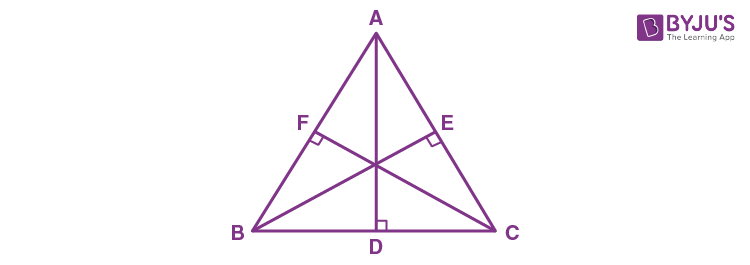In right ∆BEC and ∆BFC, we have

BE = CF [Given]

BC = BC [Common]

∠BEC = ∠BFC [Each = 900]

∴ ∆BEC ≅ ∆BFC by RHS congruence criterion

By CPCT, we get

∠B = ∠C

Similarly,

∠A = ∠B

Hence, ∠A = ∠B = ∠C

⇒ AB = BC = AC

Therefore, ABC is an equilateral triangle.

24. In a ABC, the internal bisector of angle A meets opposite side BC at point D. Through vertex C, line CE is drawn parallel to DA which meets BA produced at point E. Show that ACE is isosceles.

Solution: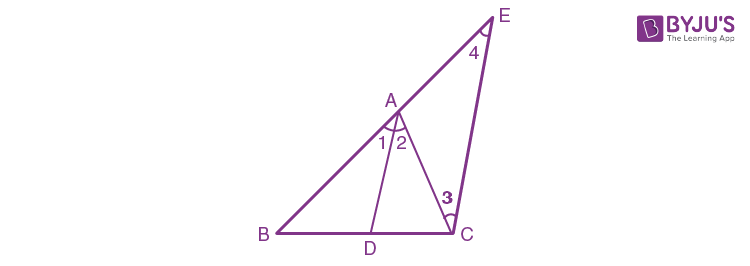Given, DA || CE

∠1 = ∠4 … (i) [Corresponding angles]

∠2 = ∠3 … (ii) [Alternate angles]

But ∠1 = ∠2 …(iii) [As AD is the bisector of ∠A]

From (i), (ii) and (iii), we get

∠3 = ∠4

⇒AC = AE

Therefore, ∆ACE is an isosceles triangle.

25. In triangle ABC, bisector of angle BAC meets opposite side BC at point D. If BD = CD, prove that ABC is isosceles.

Solution: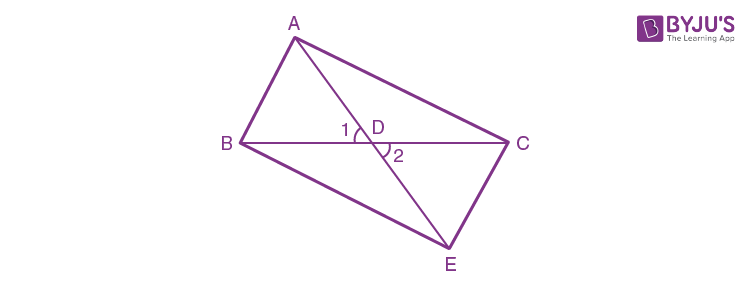In ∆ABD and ∆EDC, we have

BD = CD [Given]

∠1 = ∠2 [Vertically opposite angles]

∴ ∆ABD ≅ ∆EDC by SAS congruence criterion

So, by CPCT,

AB = CE …(i)

AC = CE …(ii)

From (i) and (ii), we get

AB = AC

Hence, ABC is an isosceles triangle.

26. In ABC, D is point on BC such that AB = AD = BD = DC. Show that:

Solution: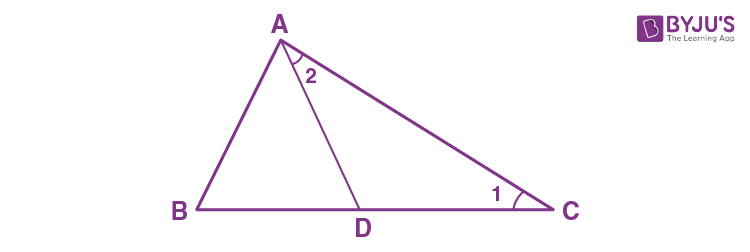As, AB = AD = BD, we have

∆ABD is an equilateral triangle.

Now,

= 180o – 60o

= 120o

∴ ∠1 = ∠2

But,

∠1 + ∠2 + ∠ADC = 180o [By angle sum property]

2∠1 + 120o = 180o

2∠1 = 60o

∠1 = 30o

∠C = 30o

⇒∠ADC: ∠C = 120o : 30o

Therefore, ∠ADC: ∠C = 4 : 1

27. Using the information given in each of the following figures, find the values of a and b.  [Given: CE = AC]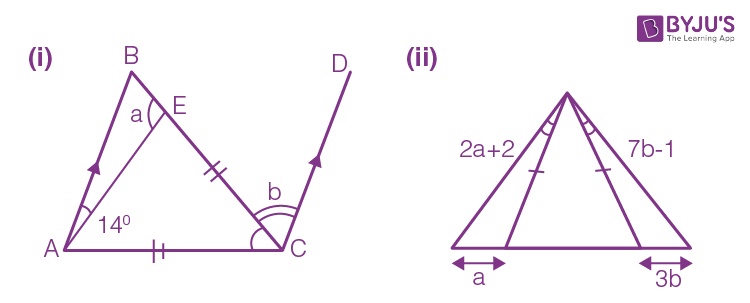Solution:

(i) In ∆CAE, we have

∠CAE = ∠AEC [∵ CE = AC]

= (180o – 60o)/2

= 56o

In ∠BEA, we have

a = 180o – 56o = 124o

In ∆ABE, we have

∠ABE = 180o – (124o + 14o)

= 180o – 138o

= 42o

(ii)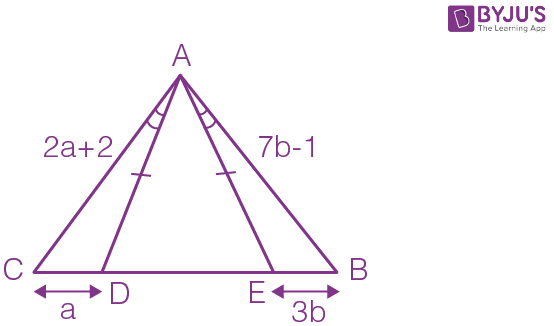In ∆AEB and ∆CAD, we have

180o – ∠ADE = 180o – ∠AED

∴ ∆AEB ≅ ∆CAD by ASA congruence criterion

Thus, AC = AB by CPCT

2a + 2 = 7b – 1

2a – 7b = – 3 … (i)

CD = EB

a = 3b … (ii)

Solving (i) and (ii) we get,

a = 9 and b = 3

## Selina Solutions for Class 9 Maths Chapter 10-Isosceles Triangle

The Chapter 10, Isosceles Triangle, contains 2 exercises and the Selina Solutions given here contains the answers for all the questions present in these exercises. Let us have a look at some of the topics that are being discussed in this chapter.

10.1 Introduction

10.2 Theorems:

1. If two sides of a triangle are equal, the angles opposite to them are also equal.
2. If two angles of a triangle are equal, the sides opposite to them are also equal.

## Selina Solutions for Class 9 Maths Chapter 10-Isosceles Triangle

An isosceles triangle is a triangle with (at least) two equal sides. This property is equal to two angles of the triangle being equal. Thus, we can say that an isosceles triangle has two equal sides as well as two equal angles. The Chapter 10 of class 9 gives the students an overview of the different properties and problems related to the Isosceles Triangle. Read and learn the Chapter 10 of Selina textbook to learn more about Isosceles Triangle along with the concepts covered in it. Solve the Selina Solutions for Class 9 effectively to score high in the examination.